# RS Aggarwal Solutions for Class 9 Chapter 15: Volume and Surface Area of Solids

The subject experts at BYJU’S have accurately designed exercise wise answers based on the understanding ability of the students. RS Aggarwal Solutions for Class 9 is an advantageous reference material for the students to clear doubts spontaneously. The faculties prepare the solutions in a precise manner having a concept based approach which is important from exam point of view.

The solutions are well structured in order to improve the concept based knowledge among the students. The chapter wise solutions are available in PDF format which is accessible by the students. RS Aggarwal Solutions for Class 9 Chapter 15 Volume and Surface Area of Solids are provided here.

## RS Aggarwal Solutions for Class 9 Chapter 15: Volume and Surface Area of Solids Download PDF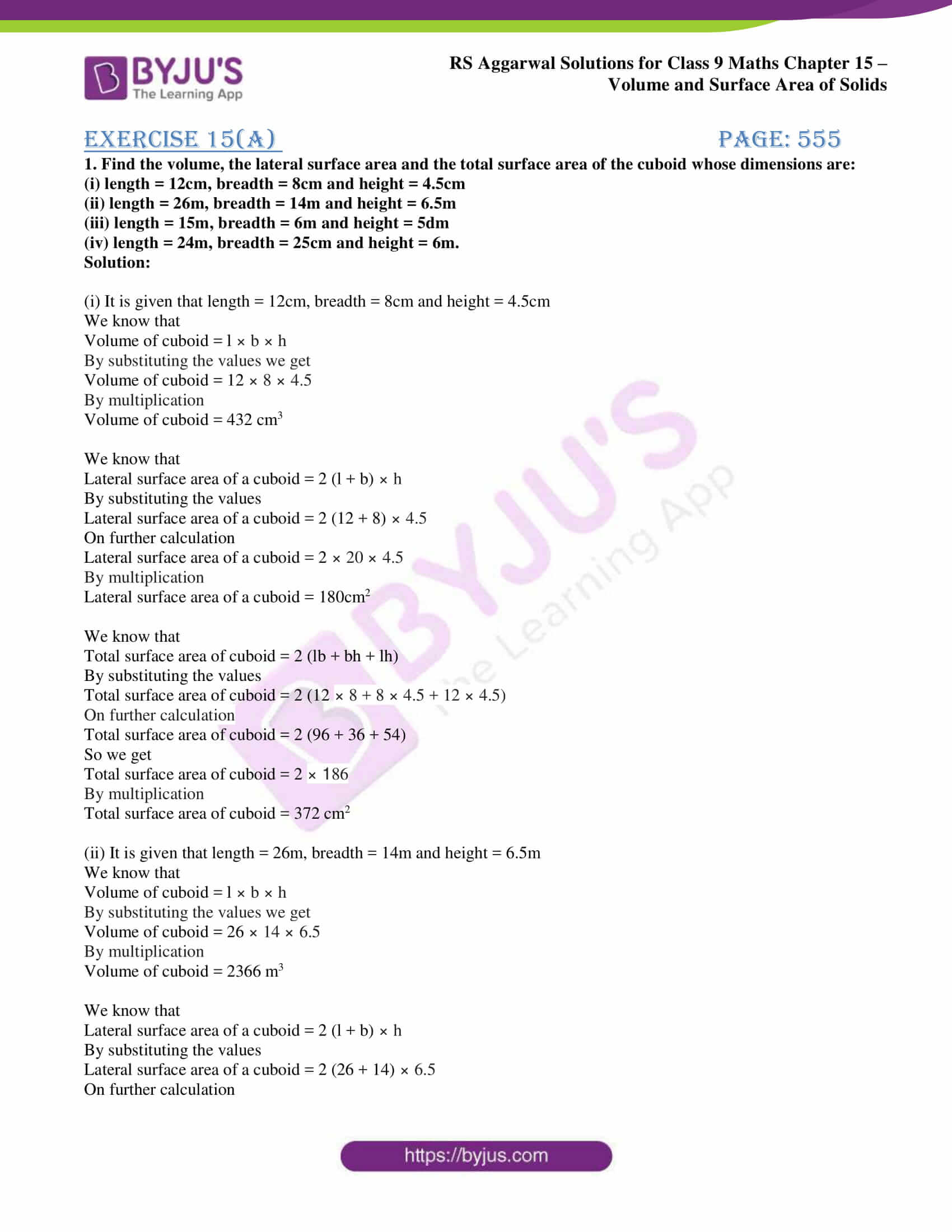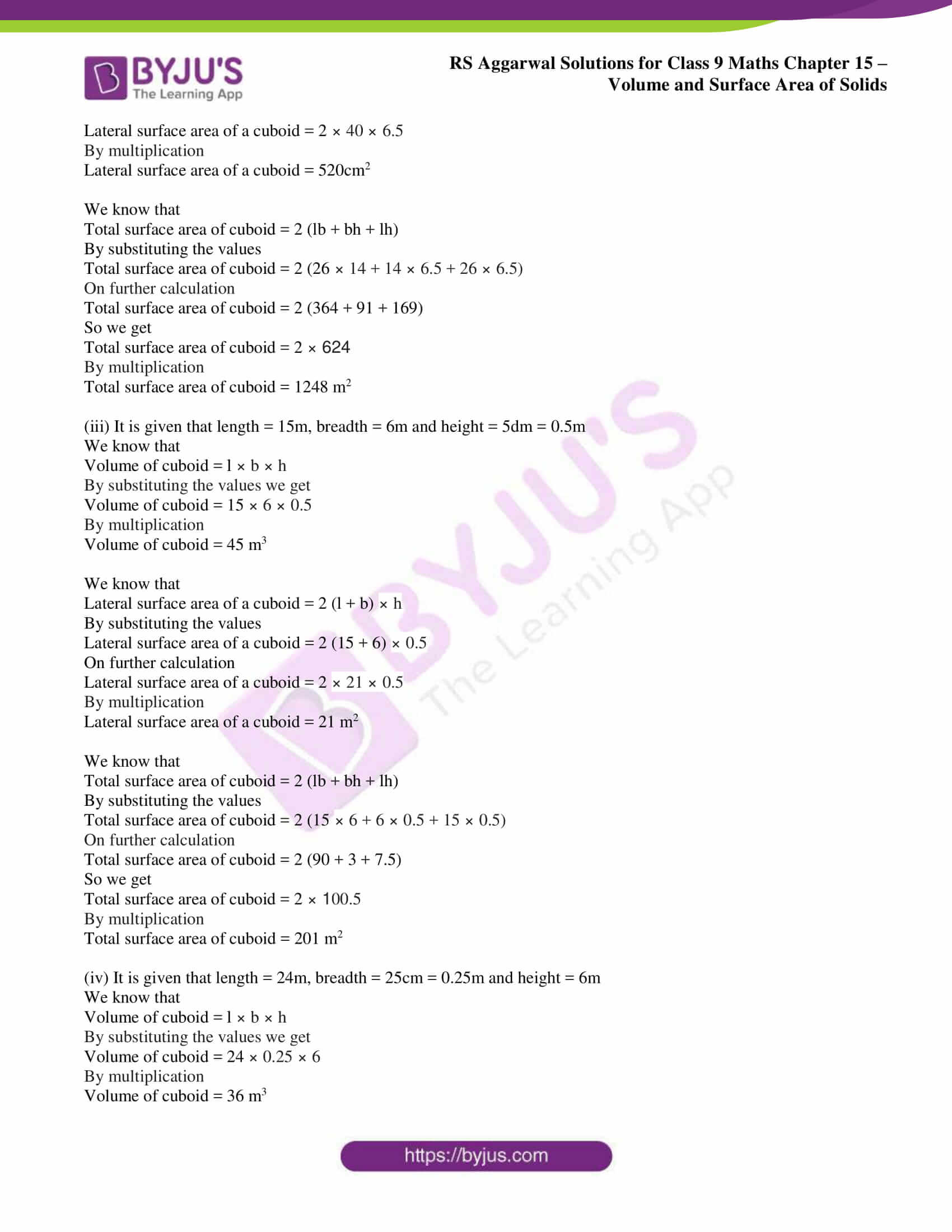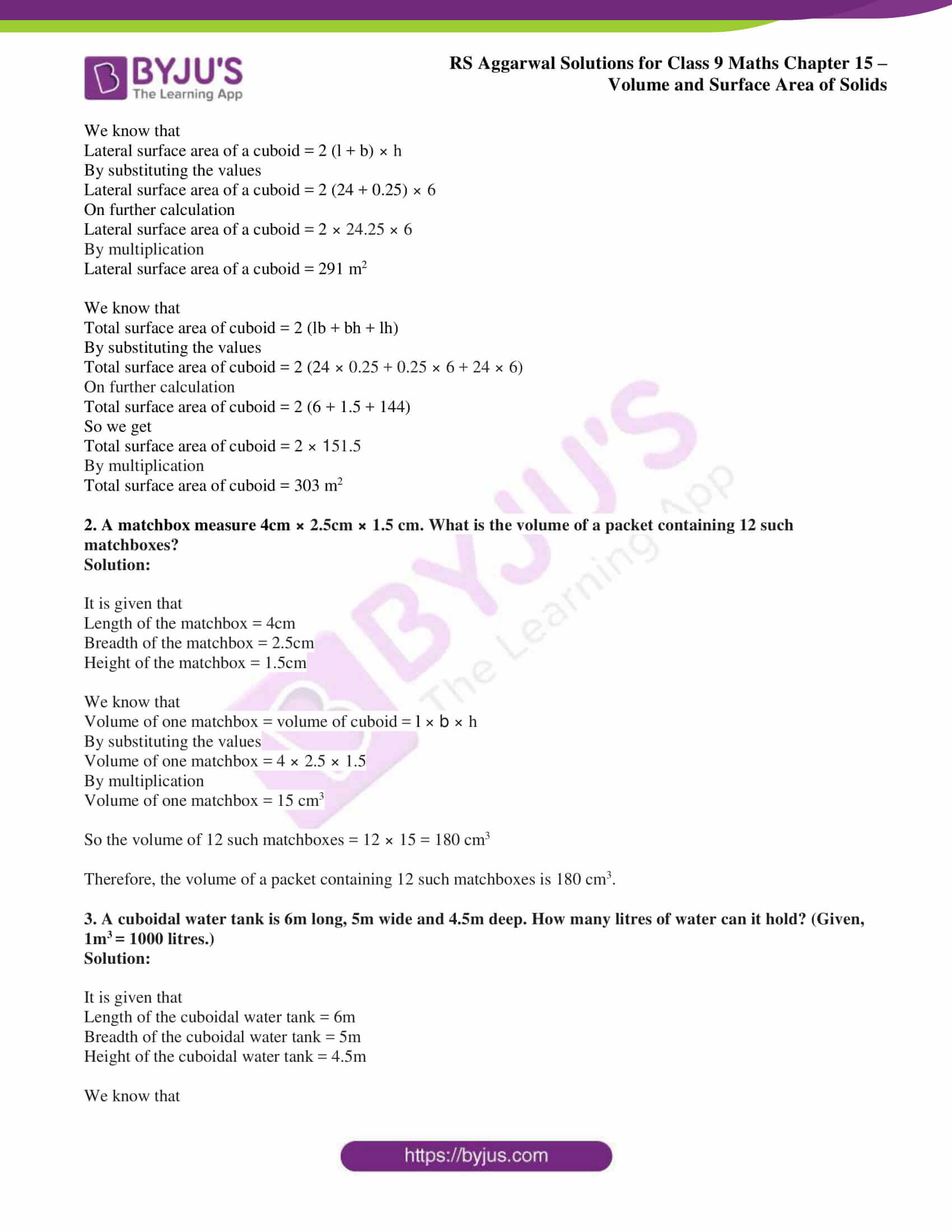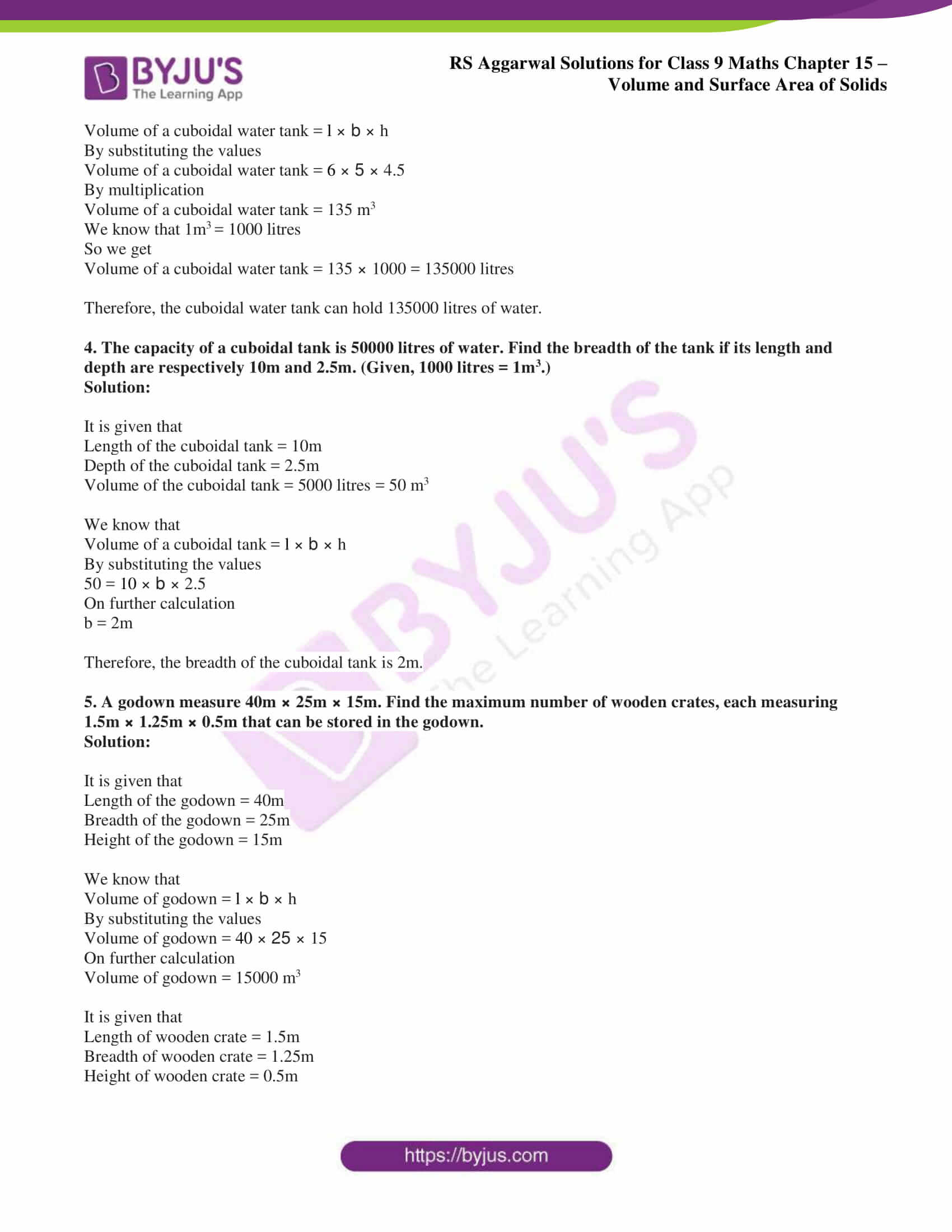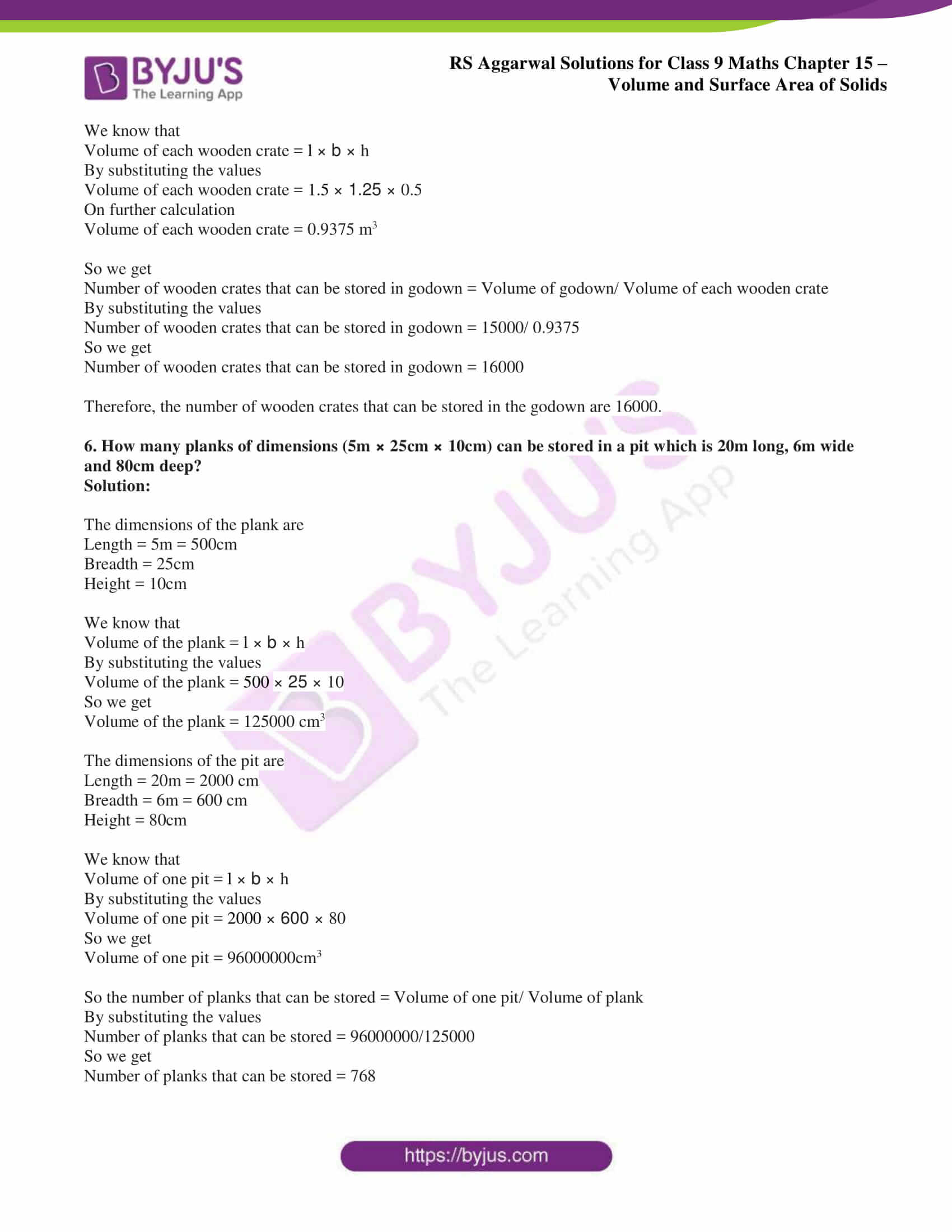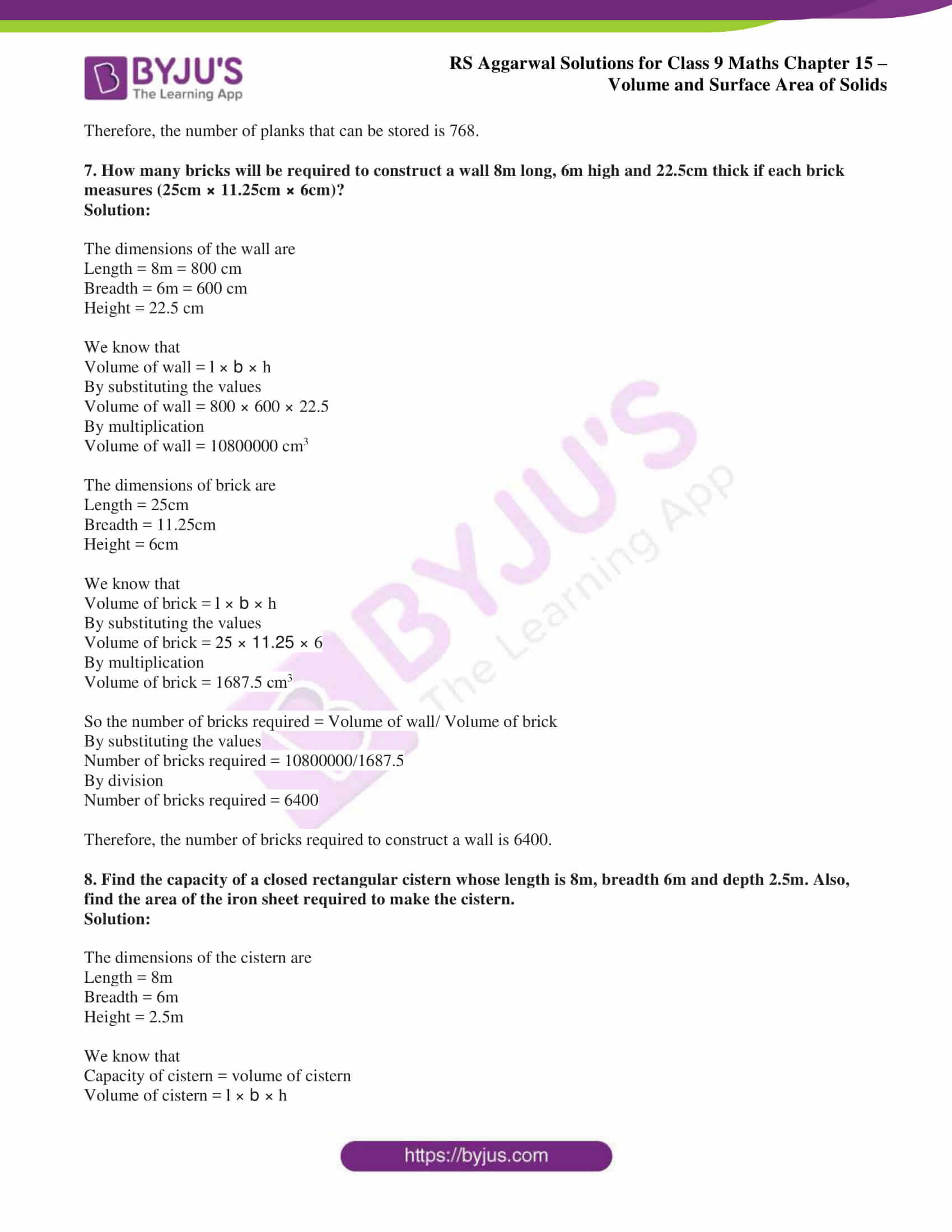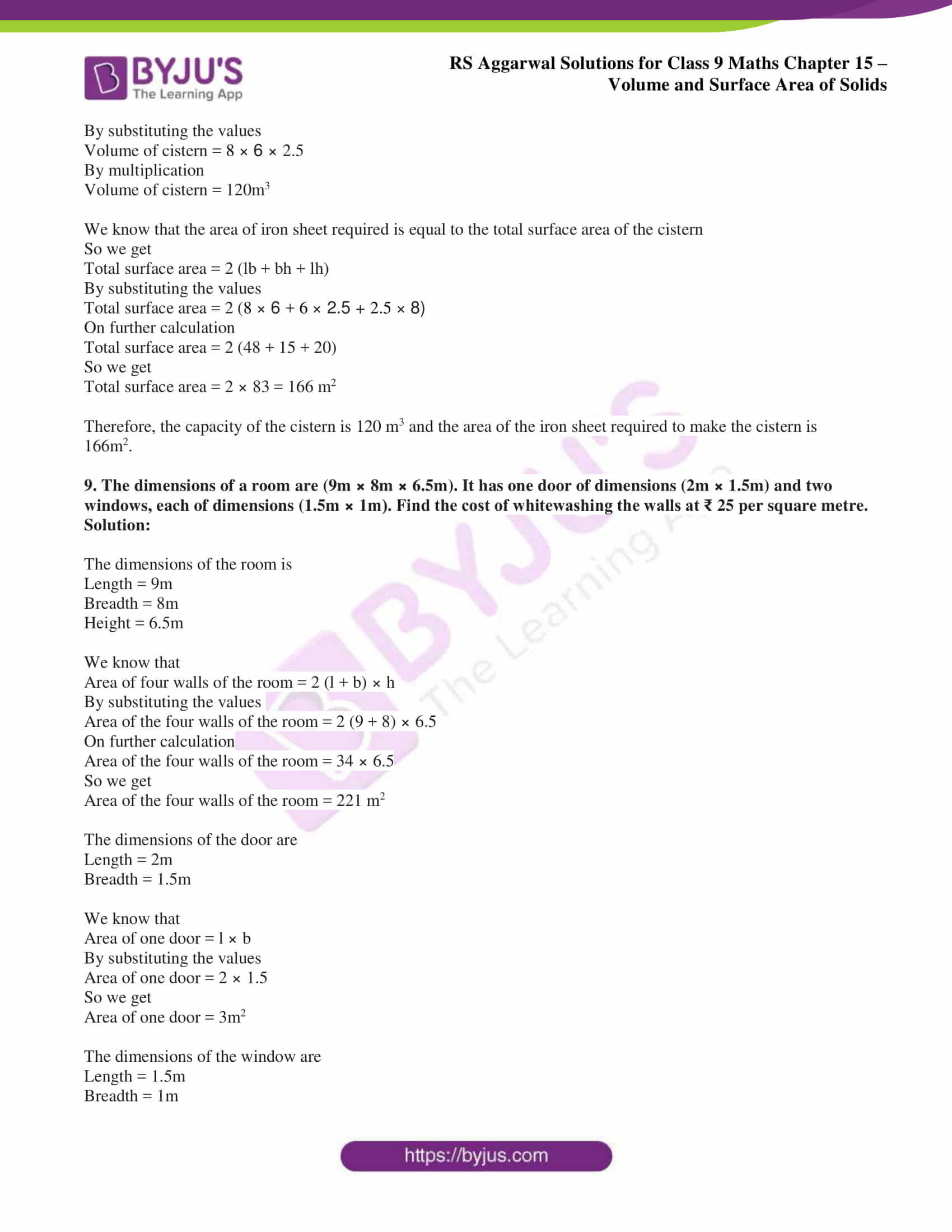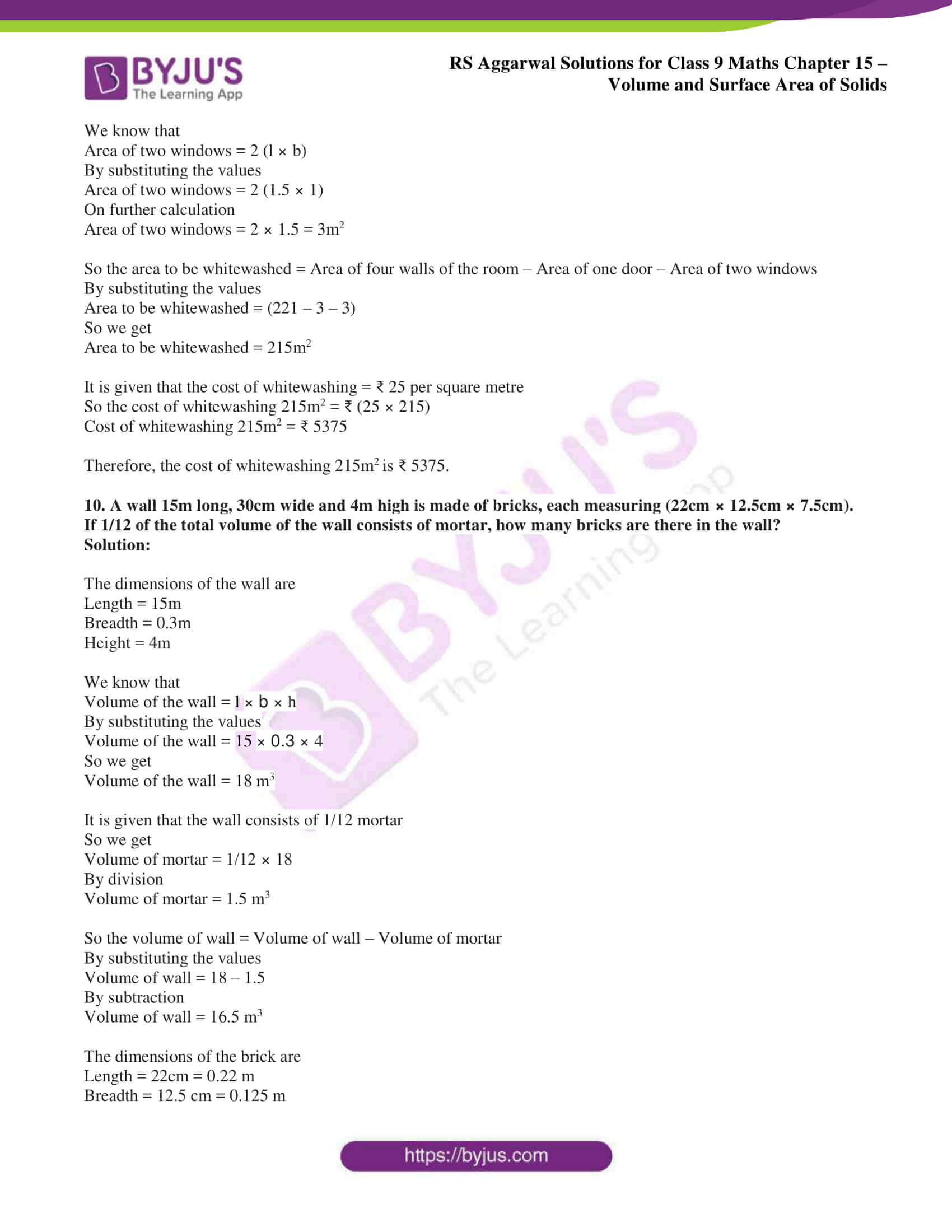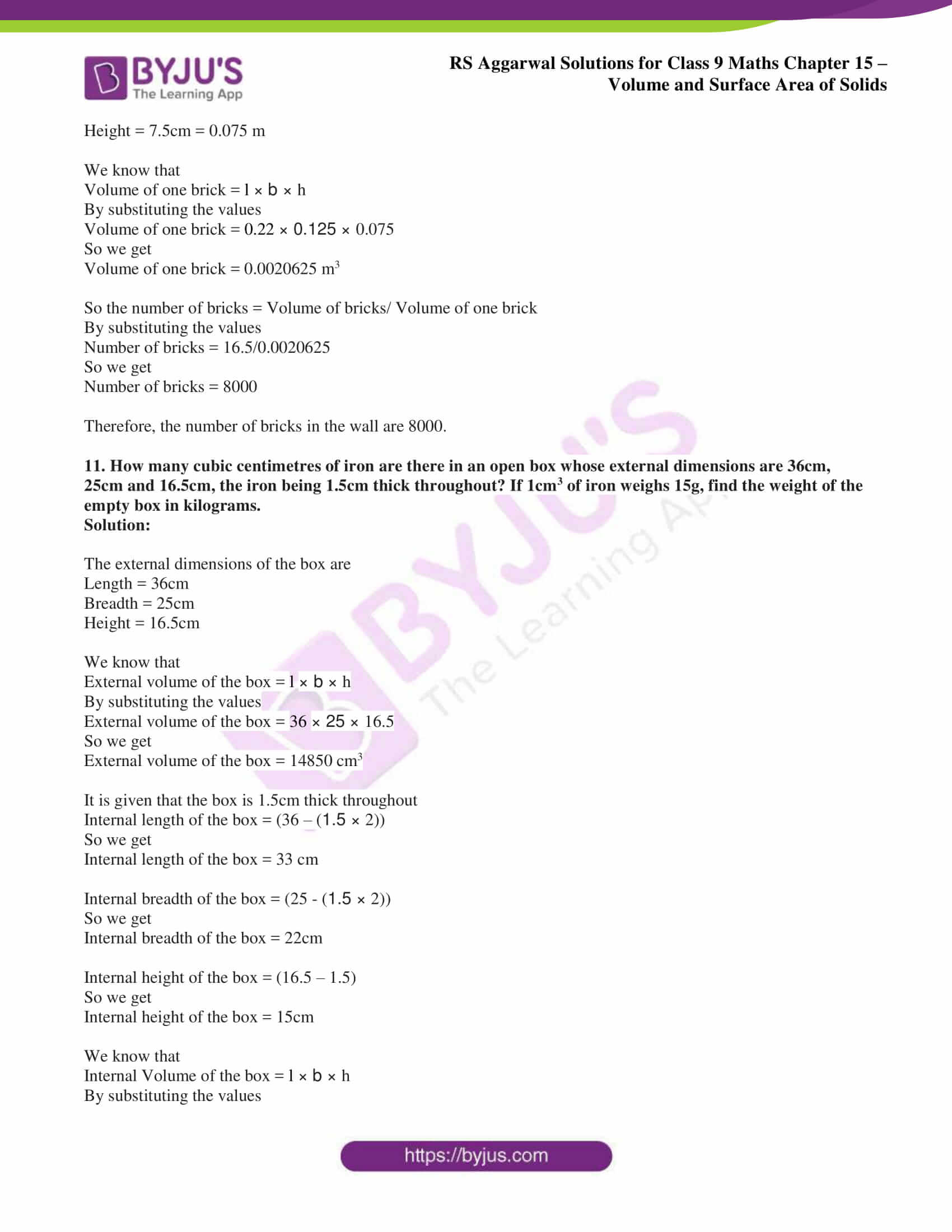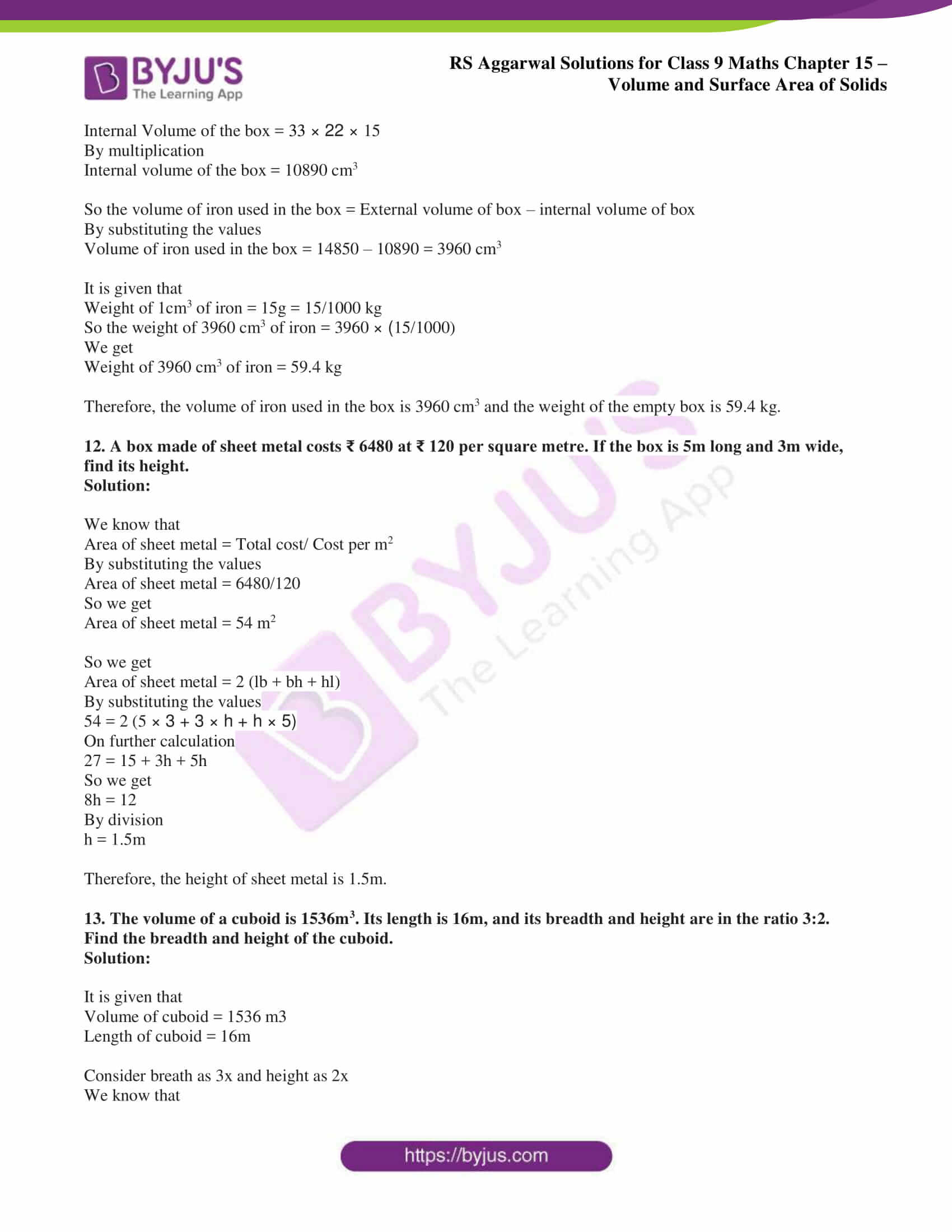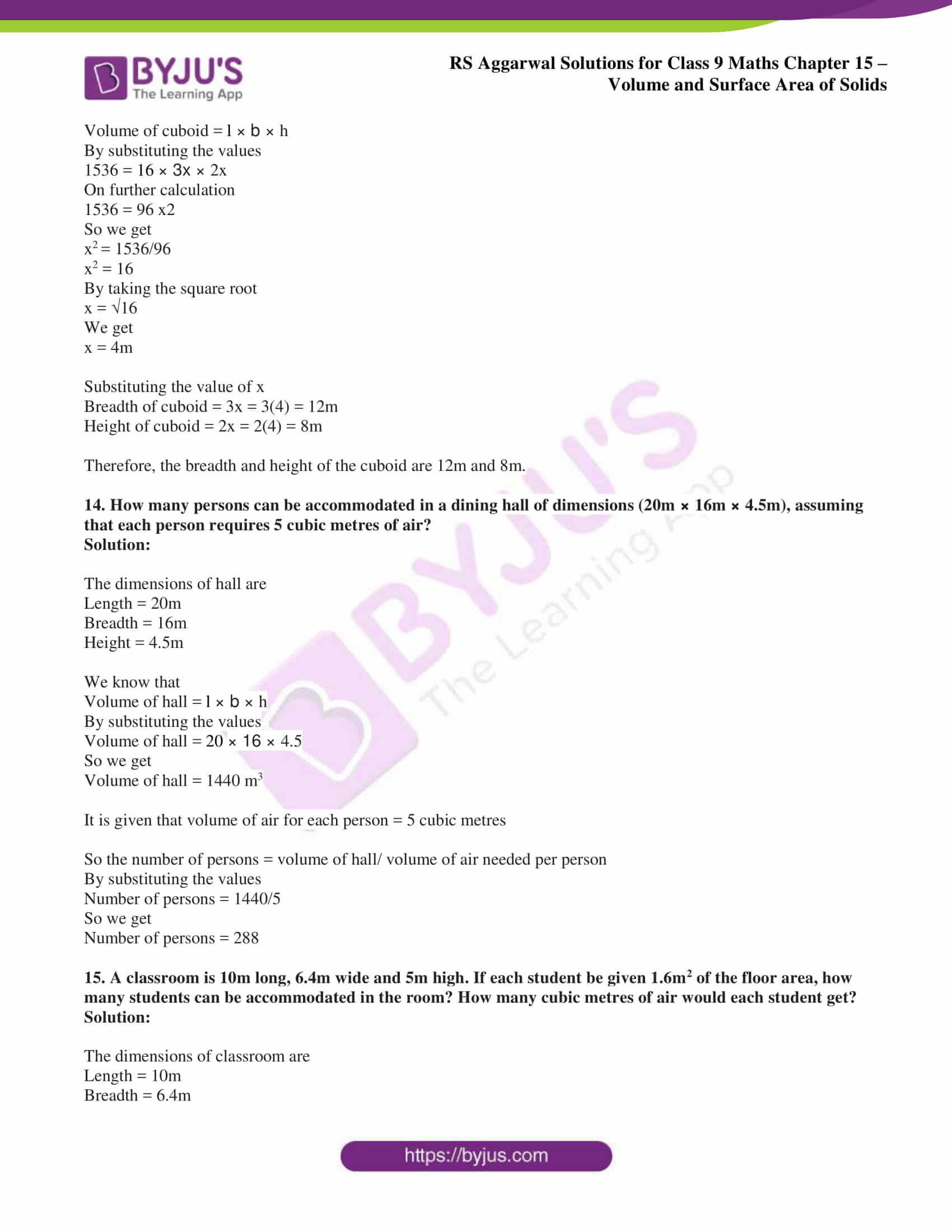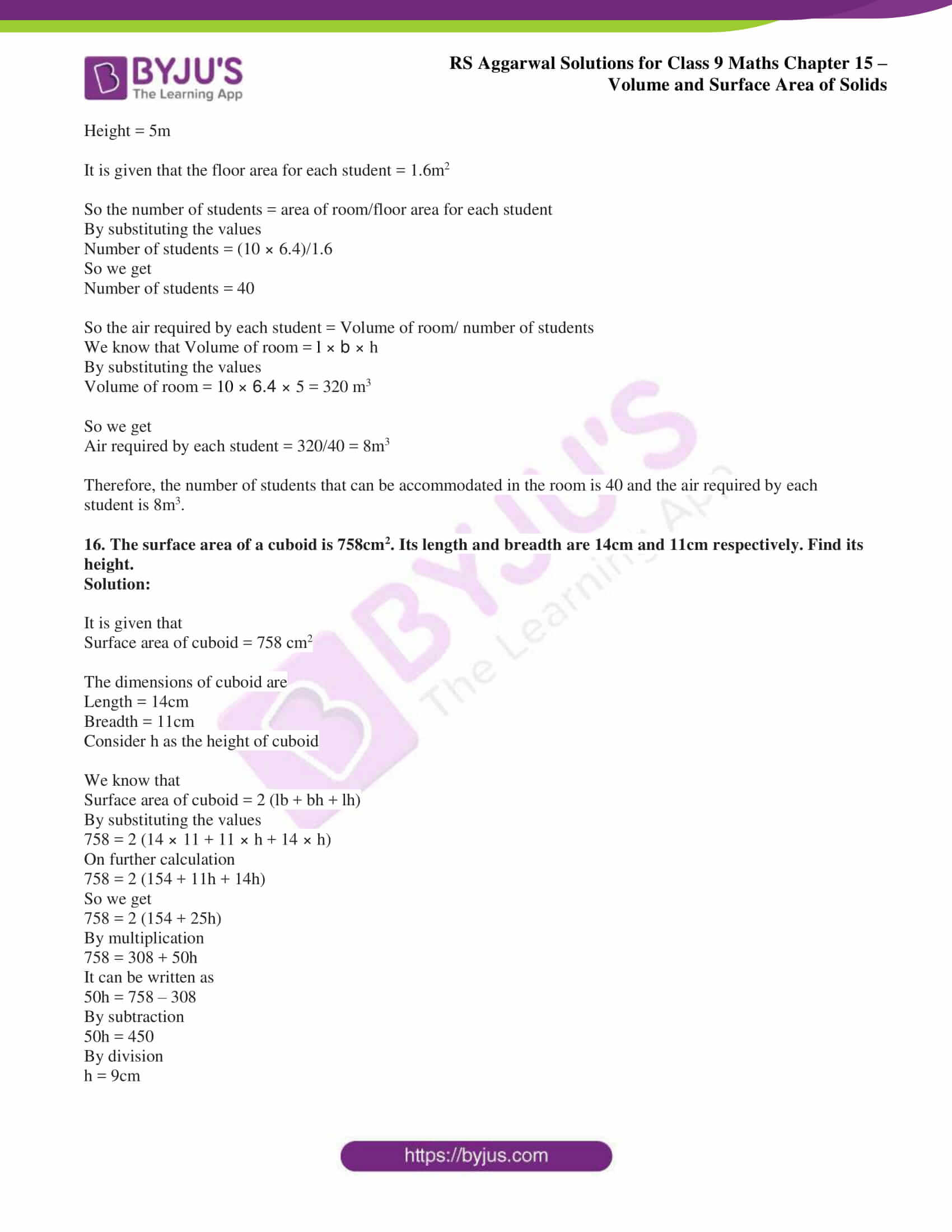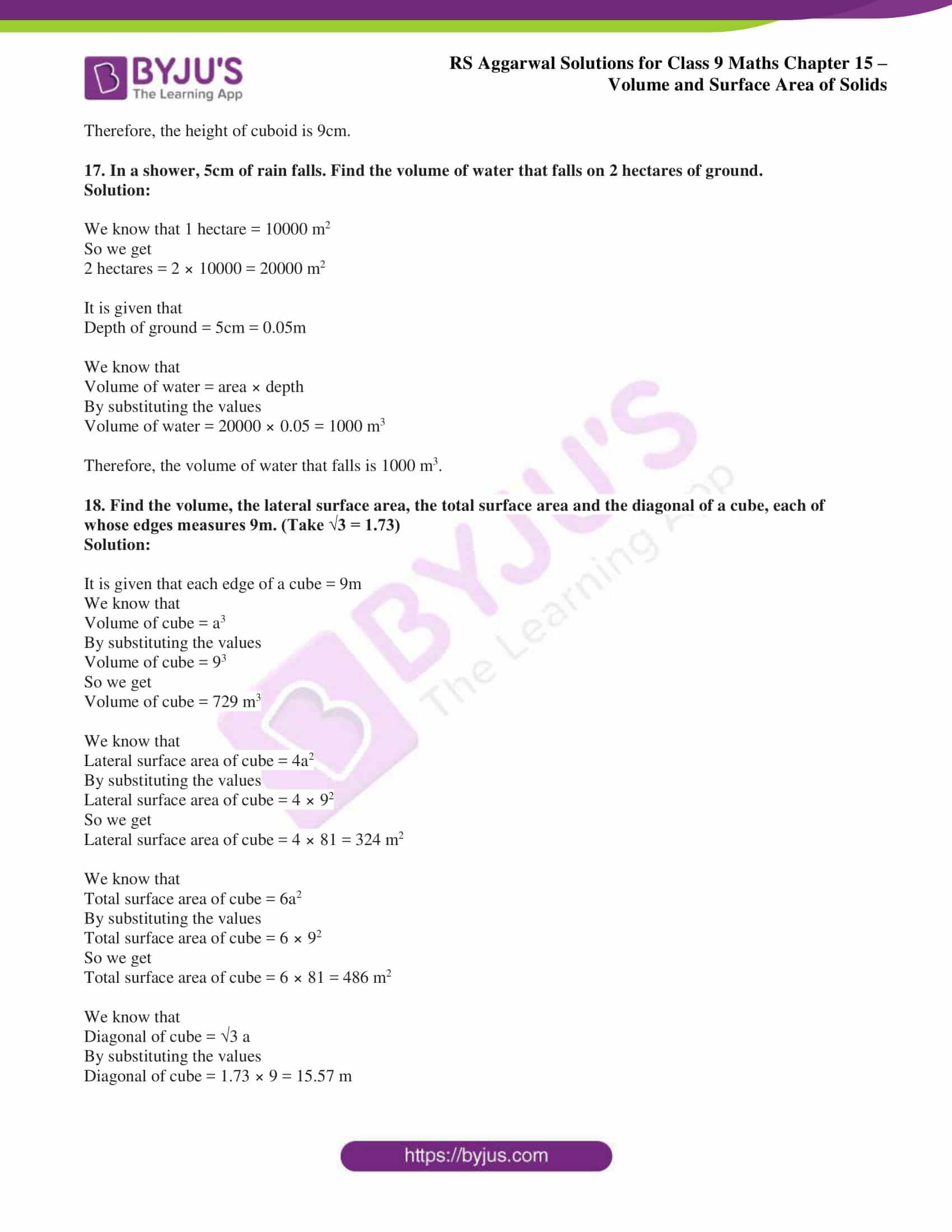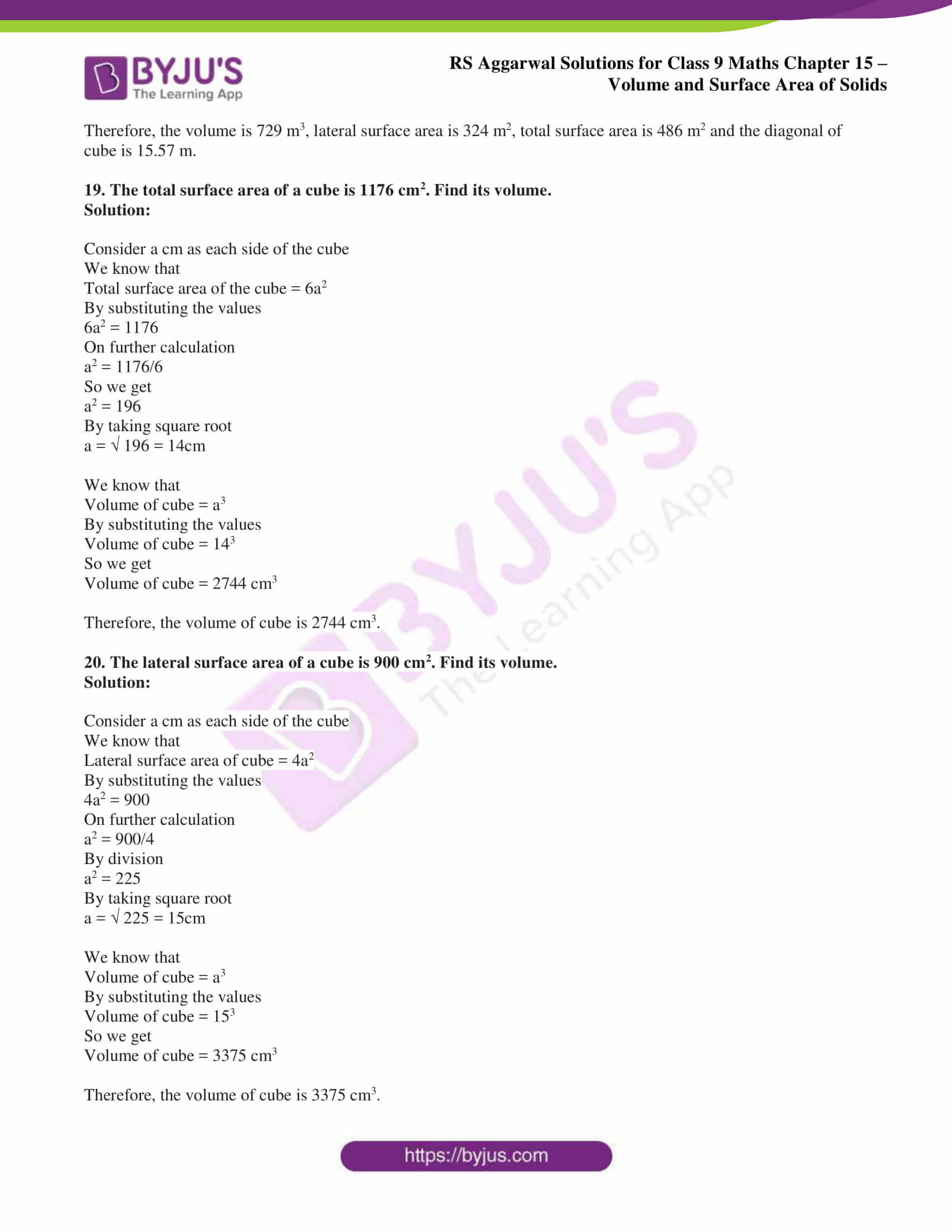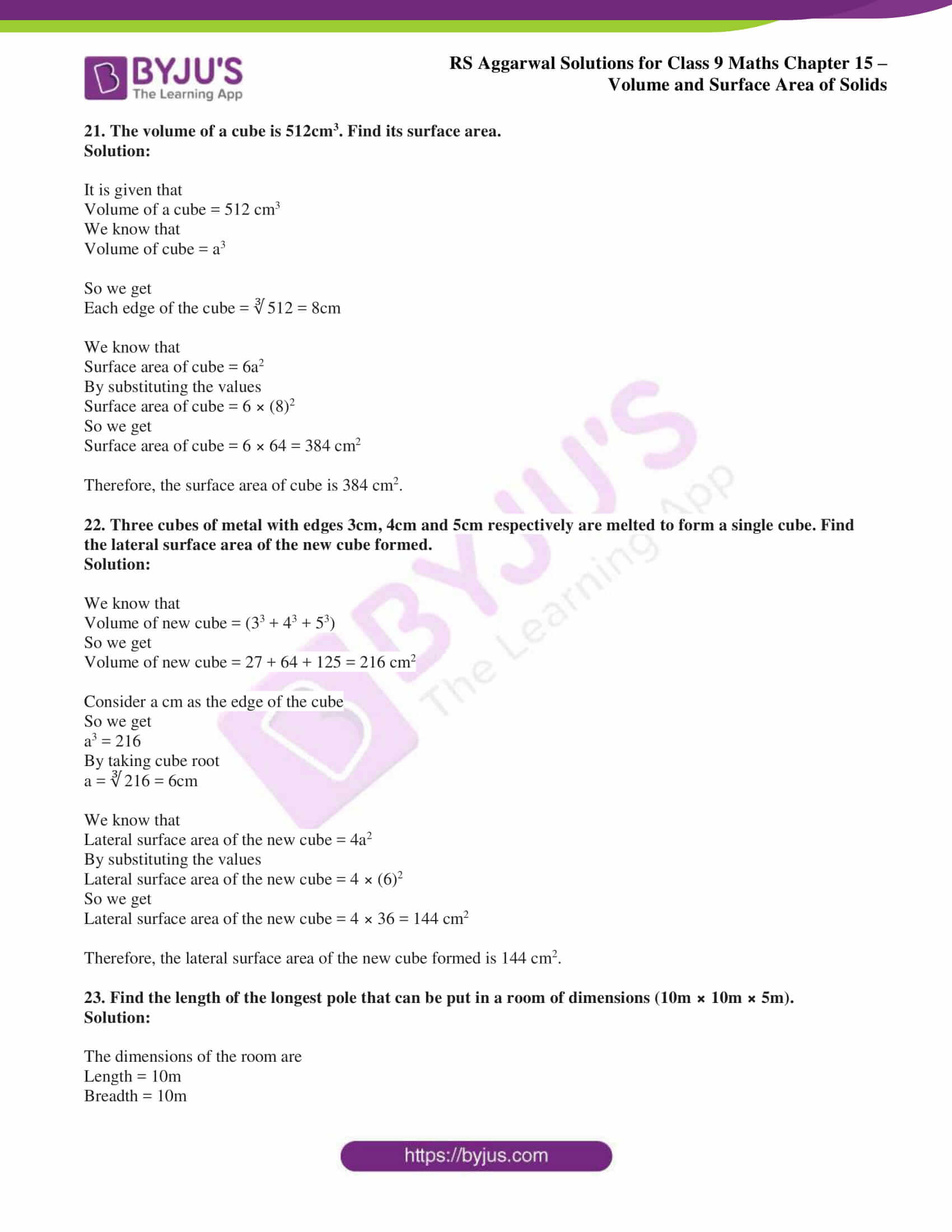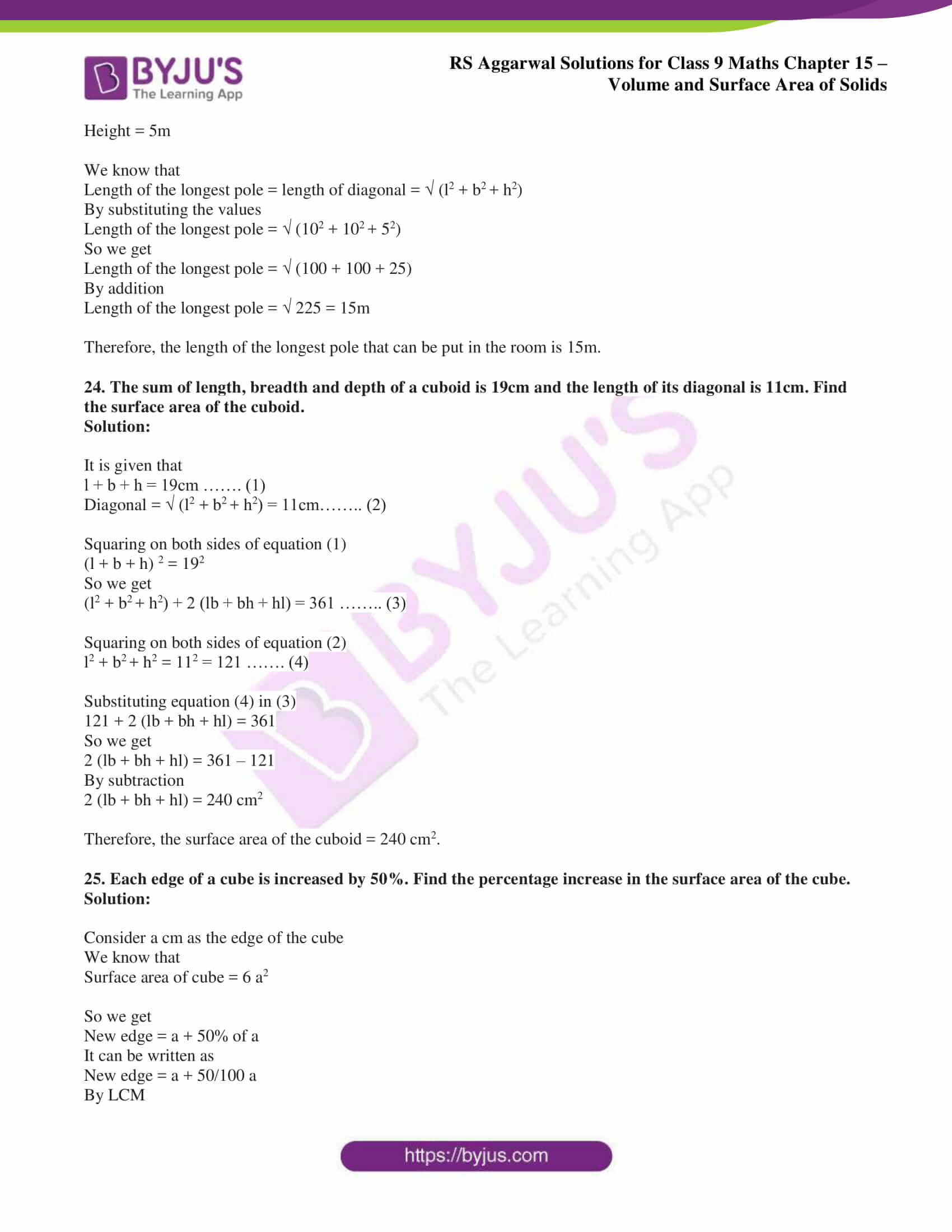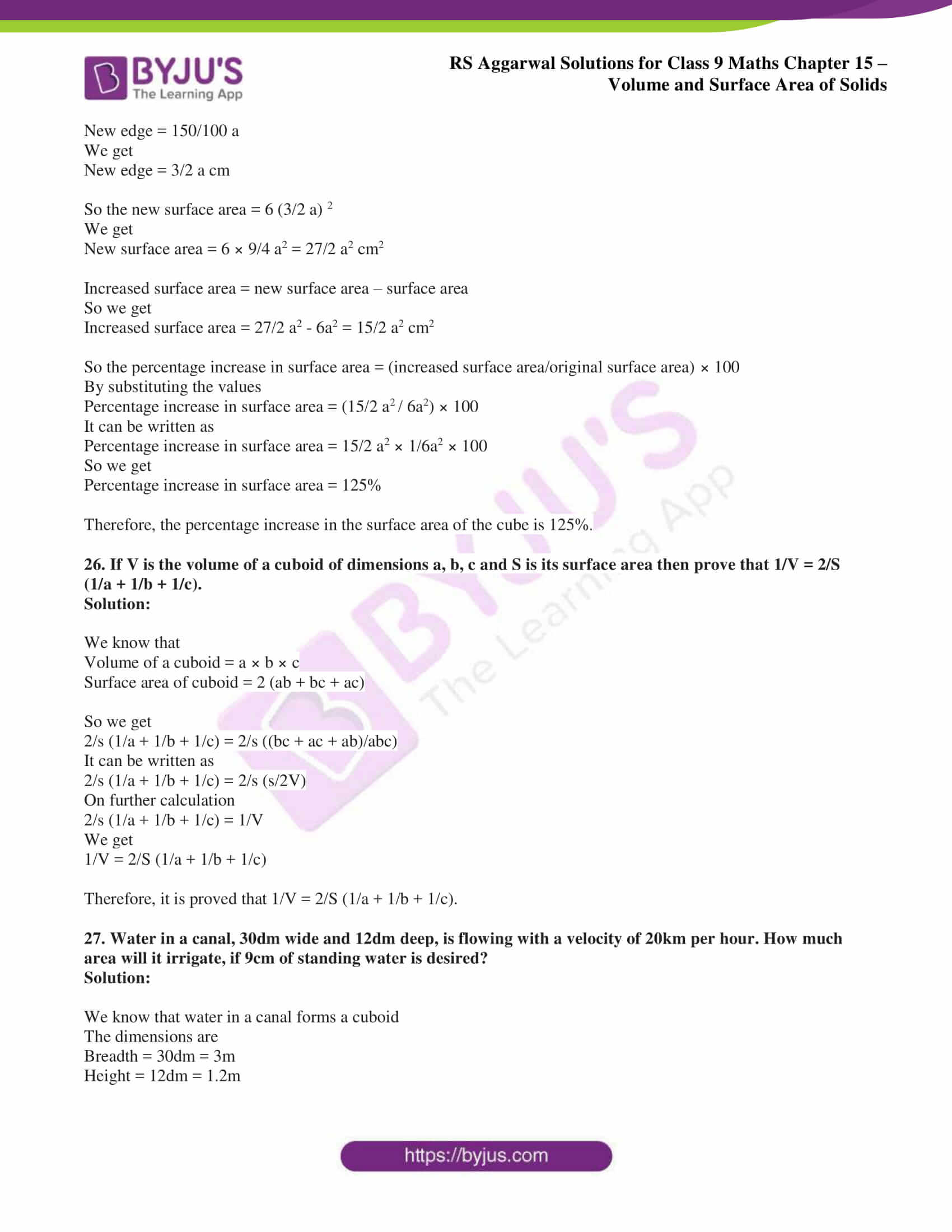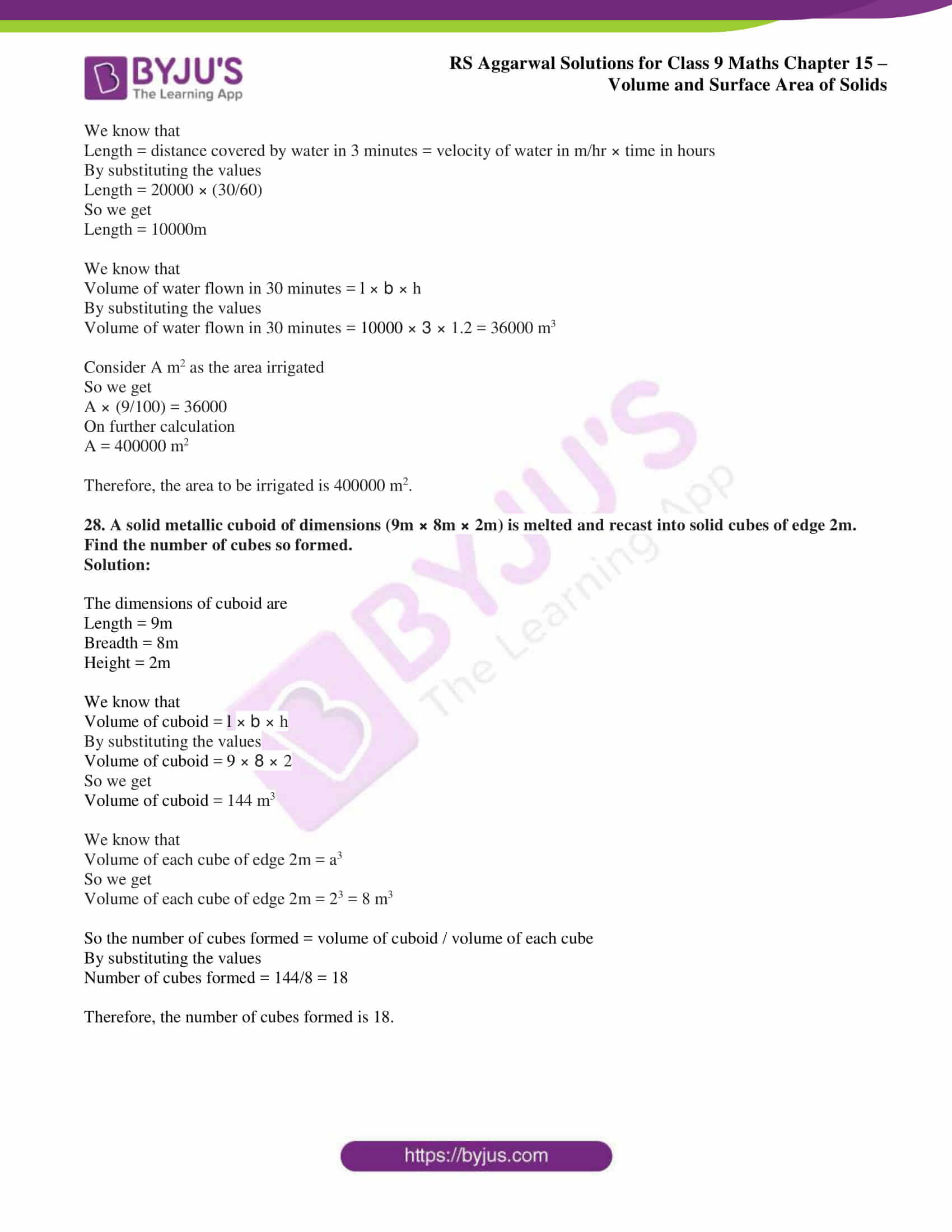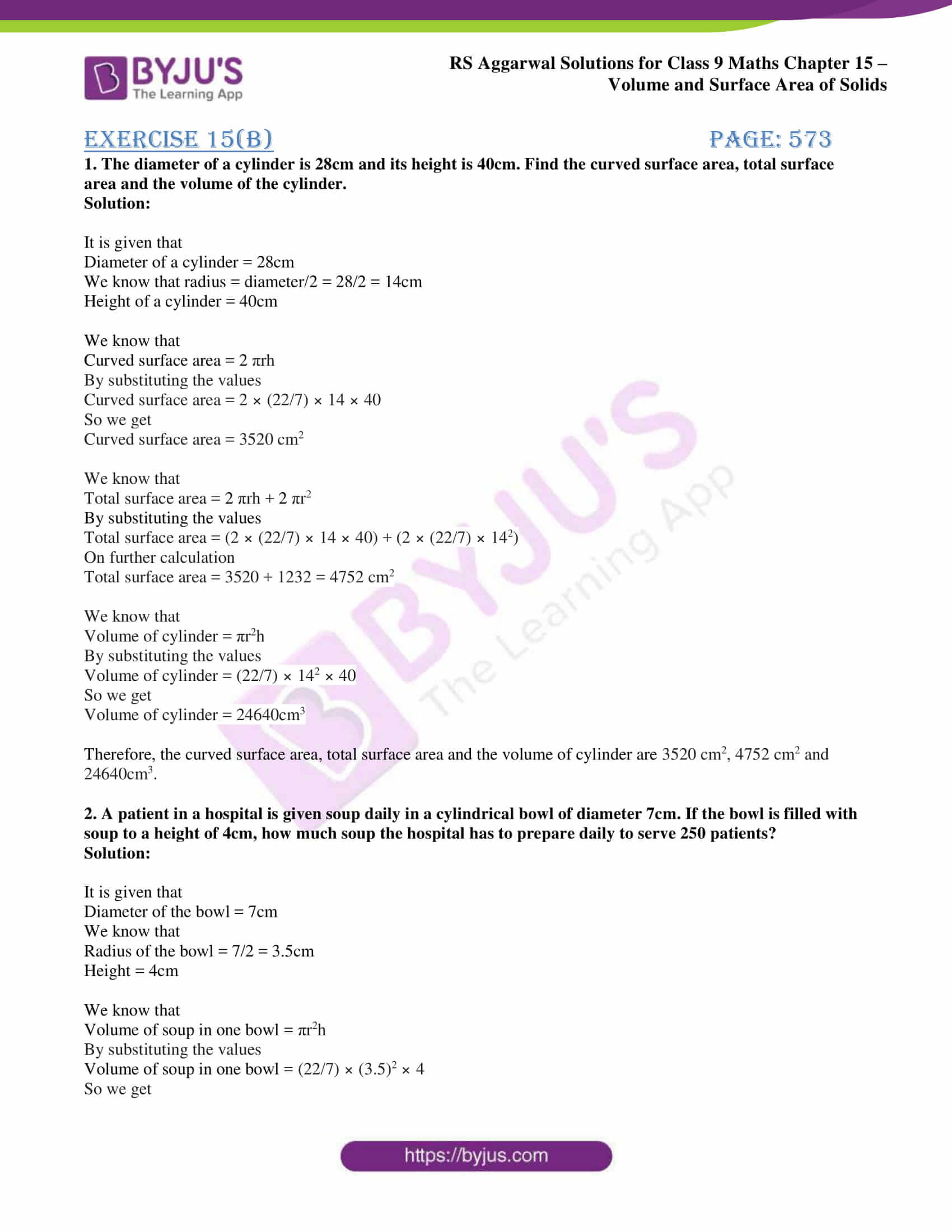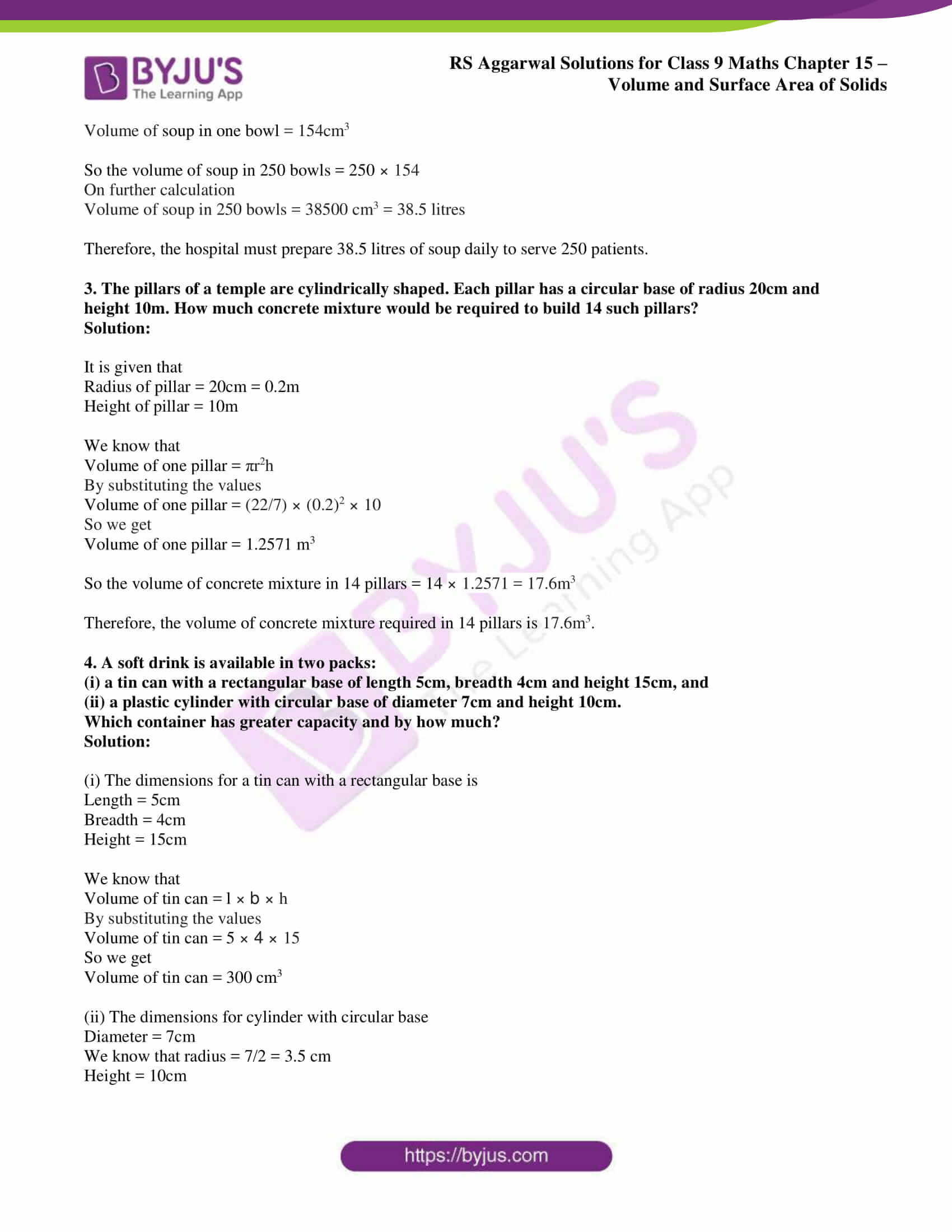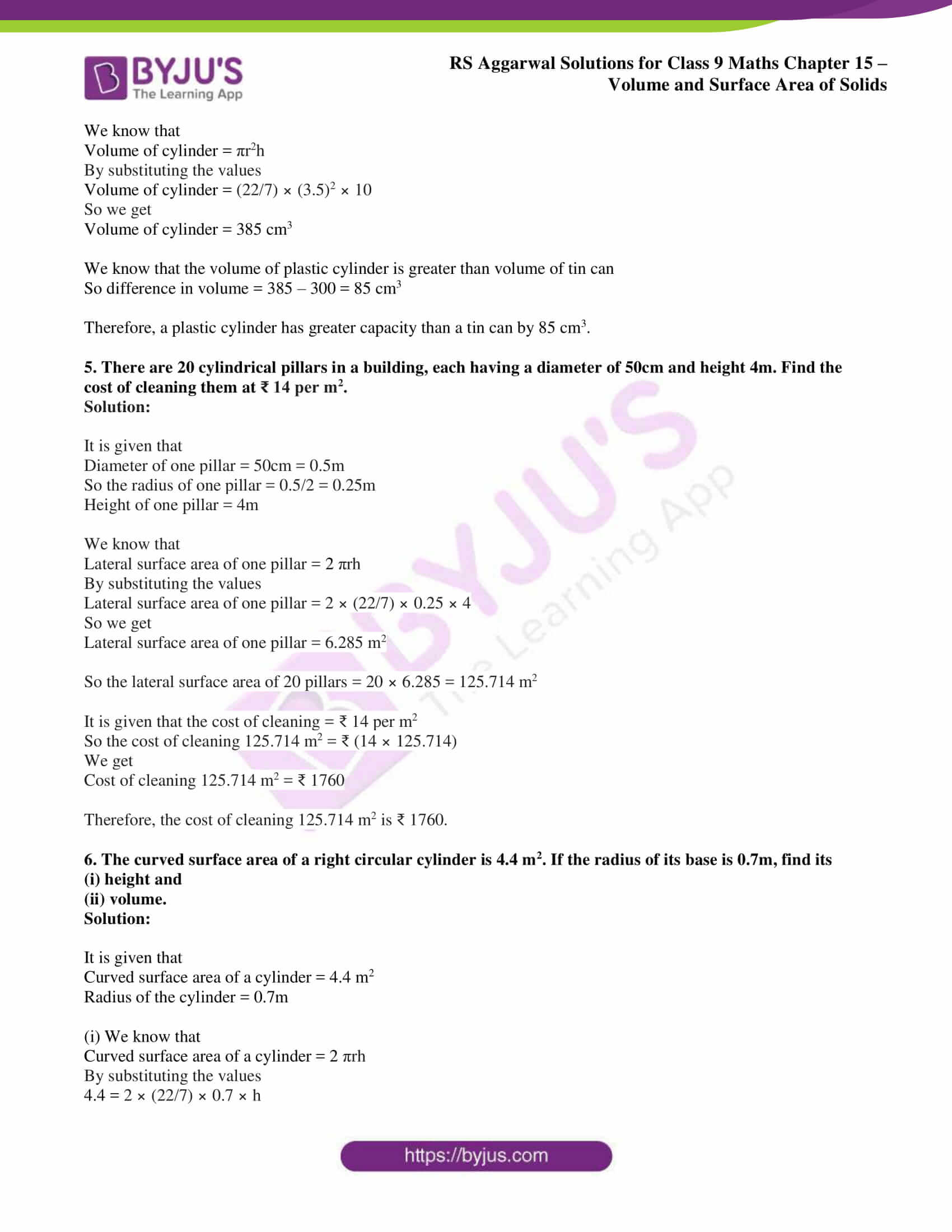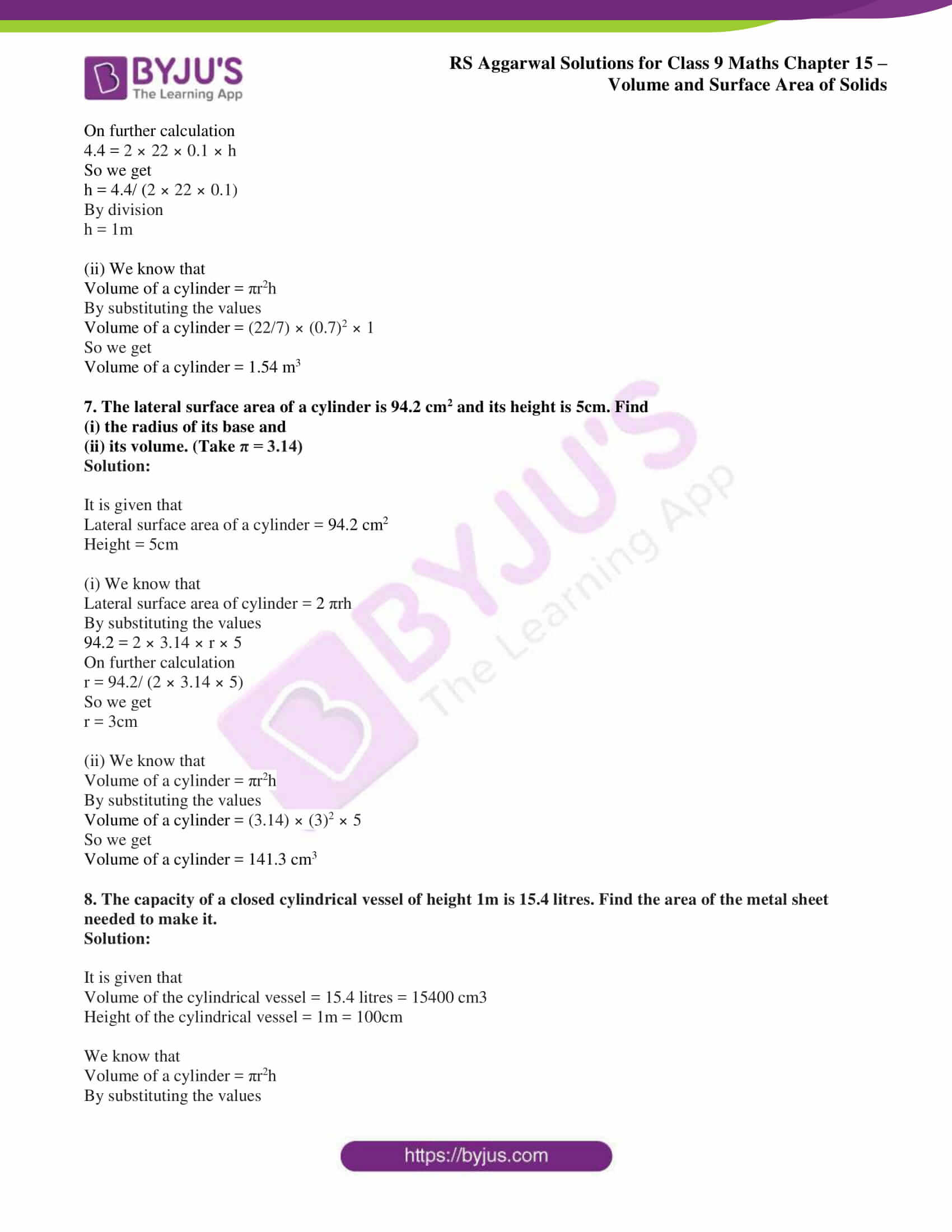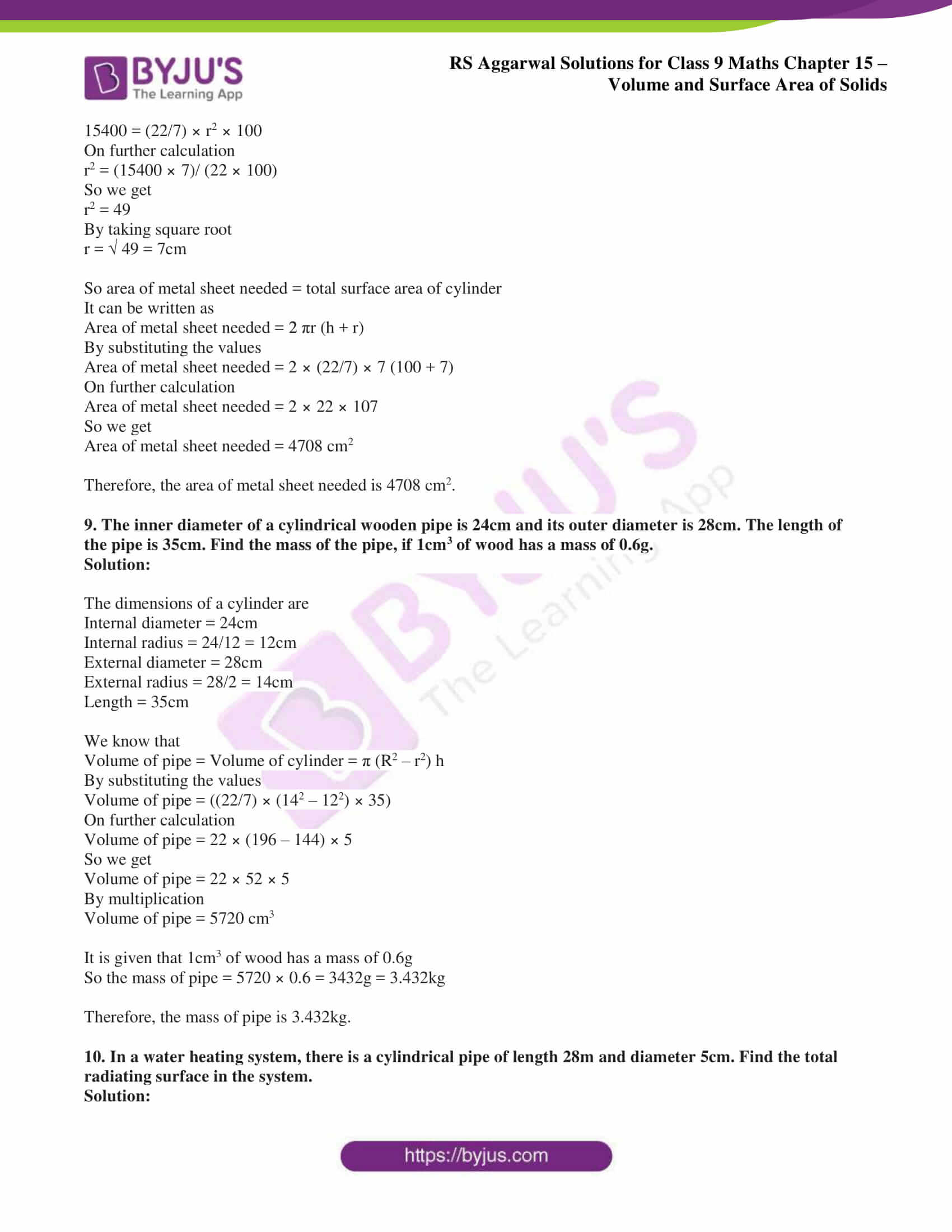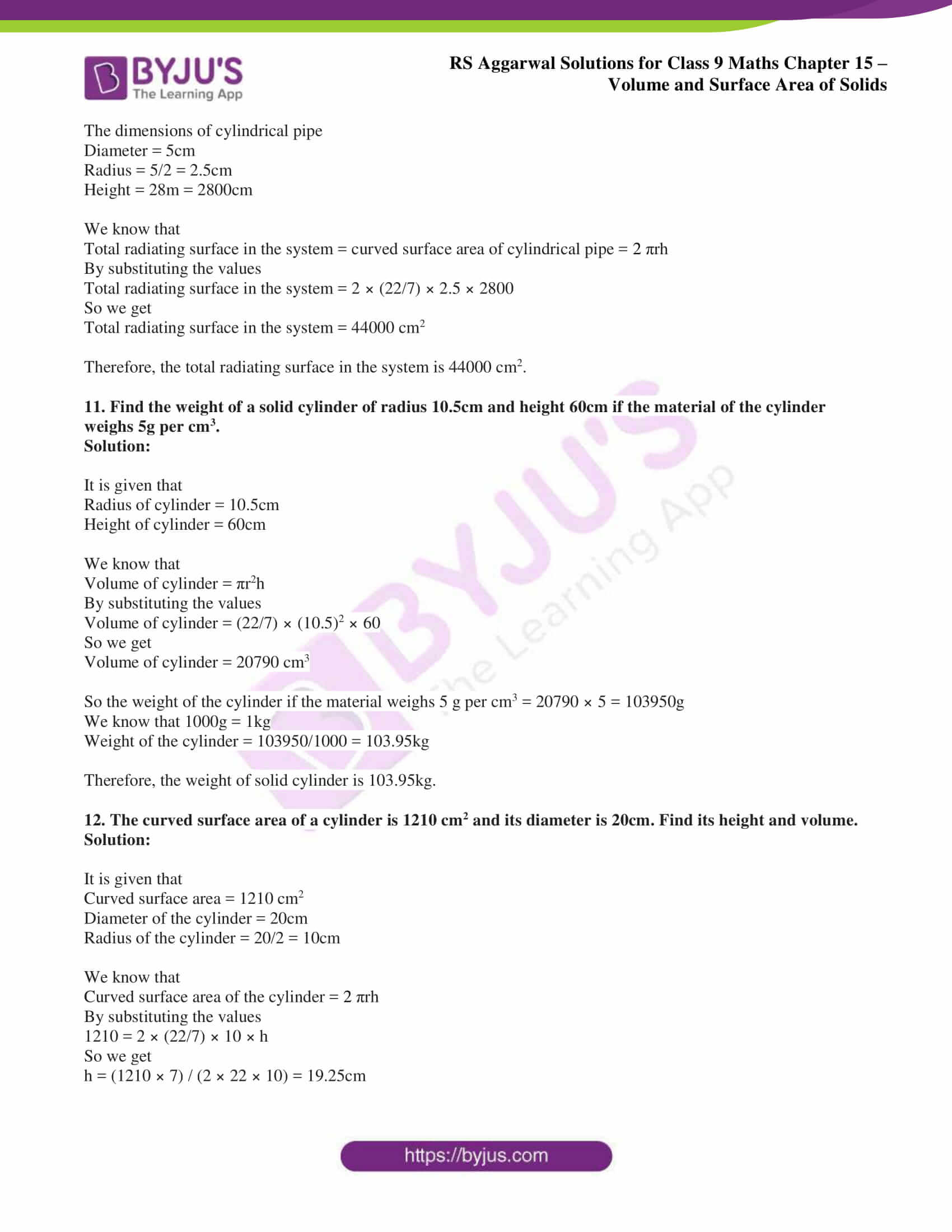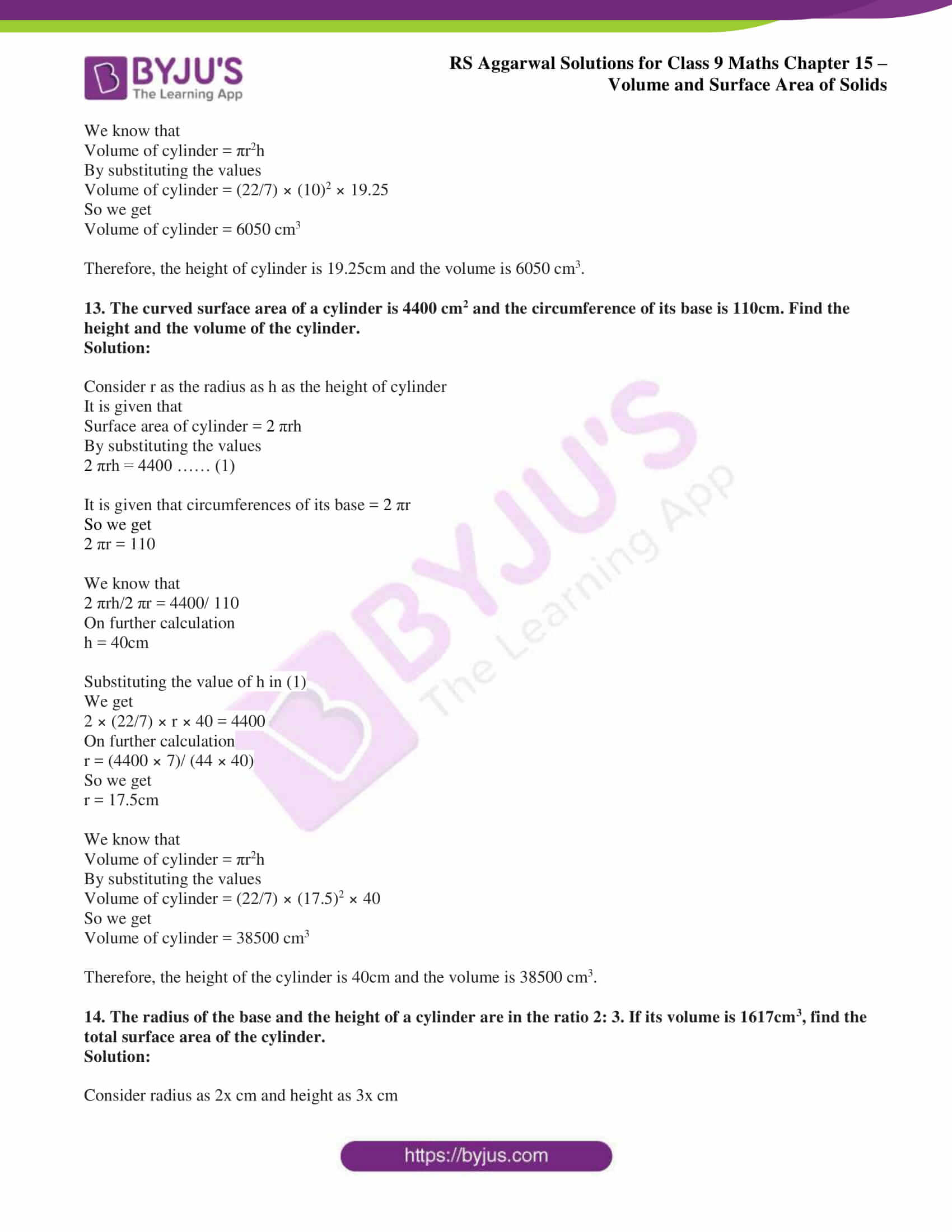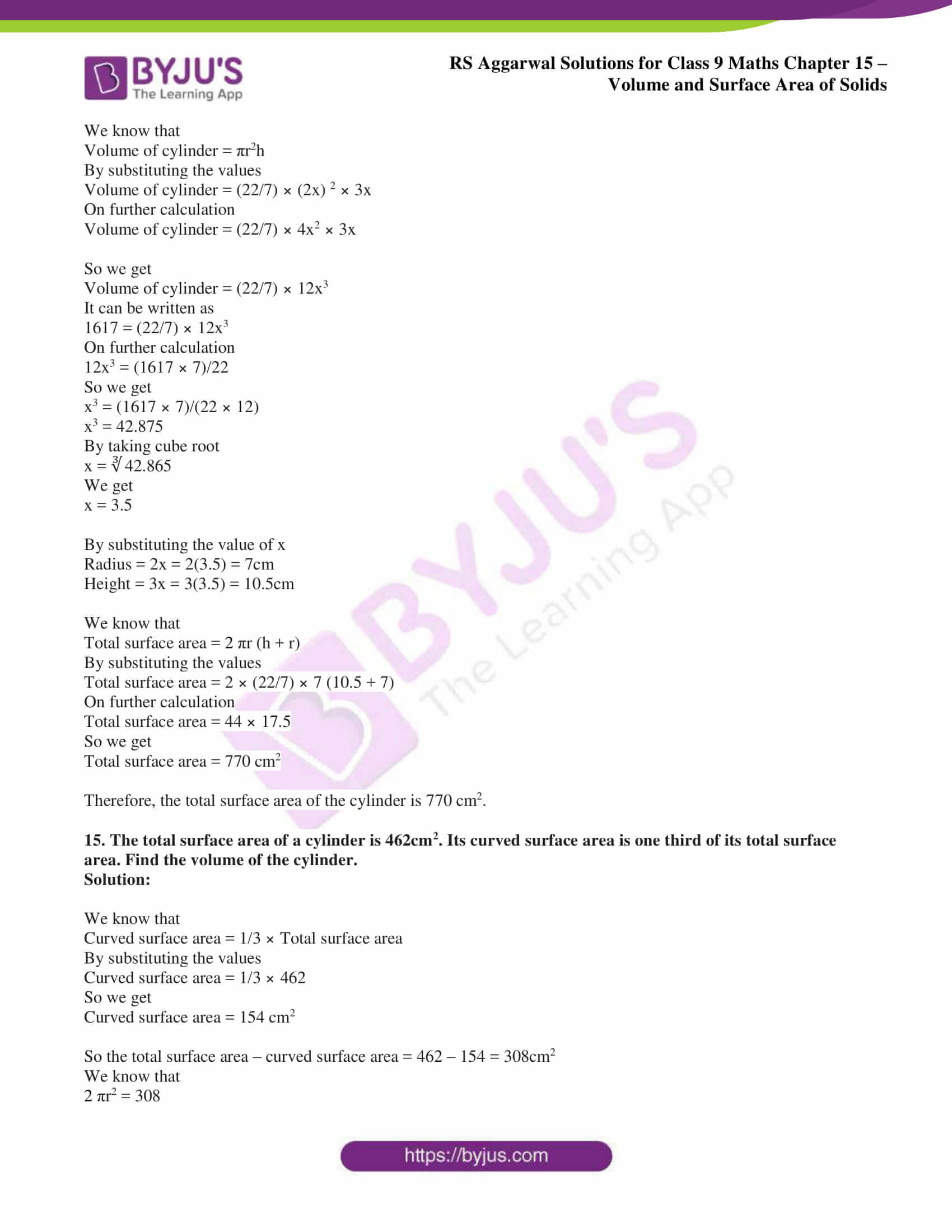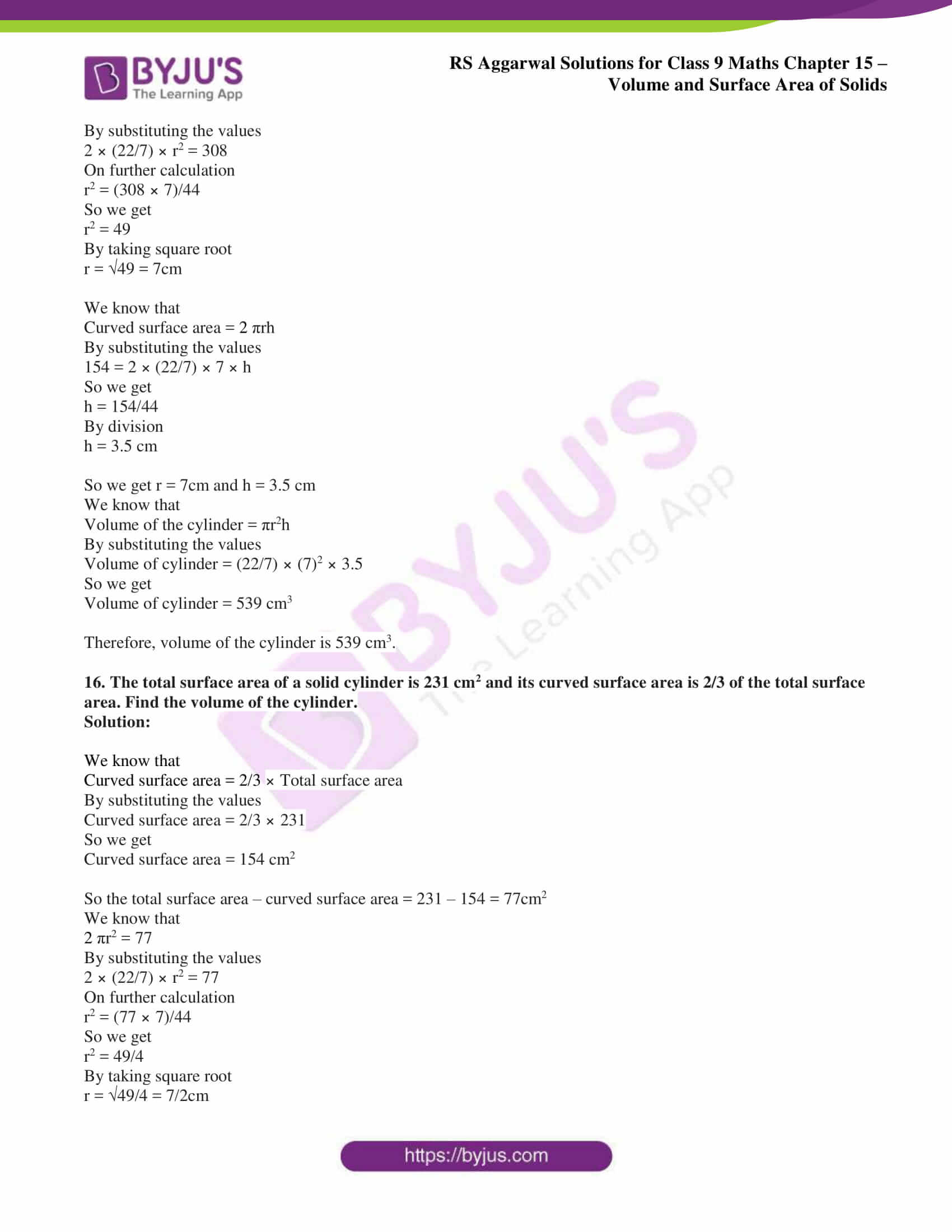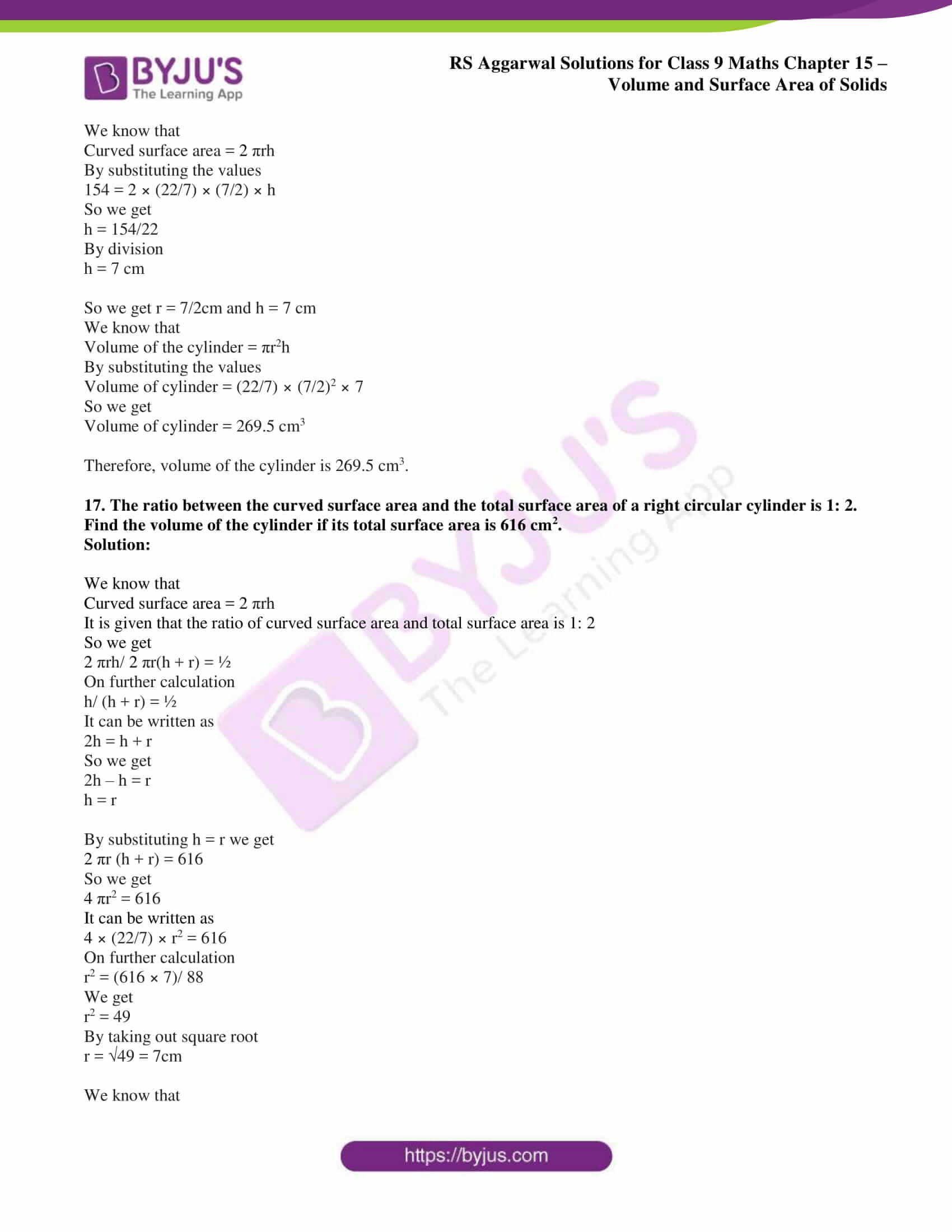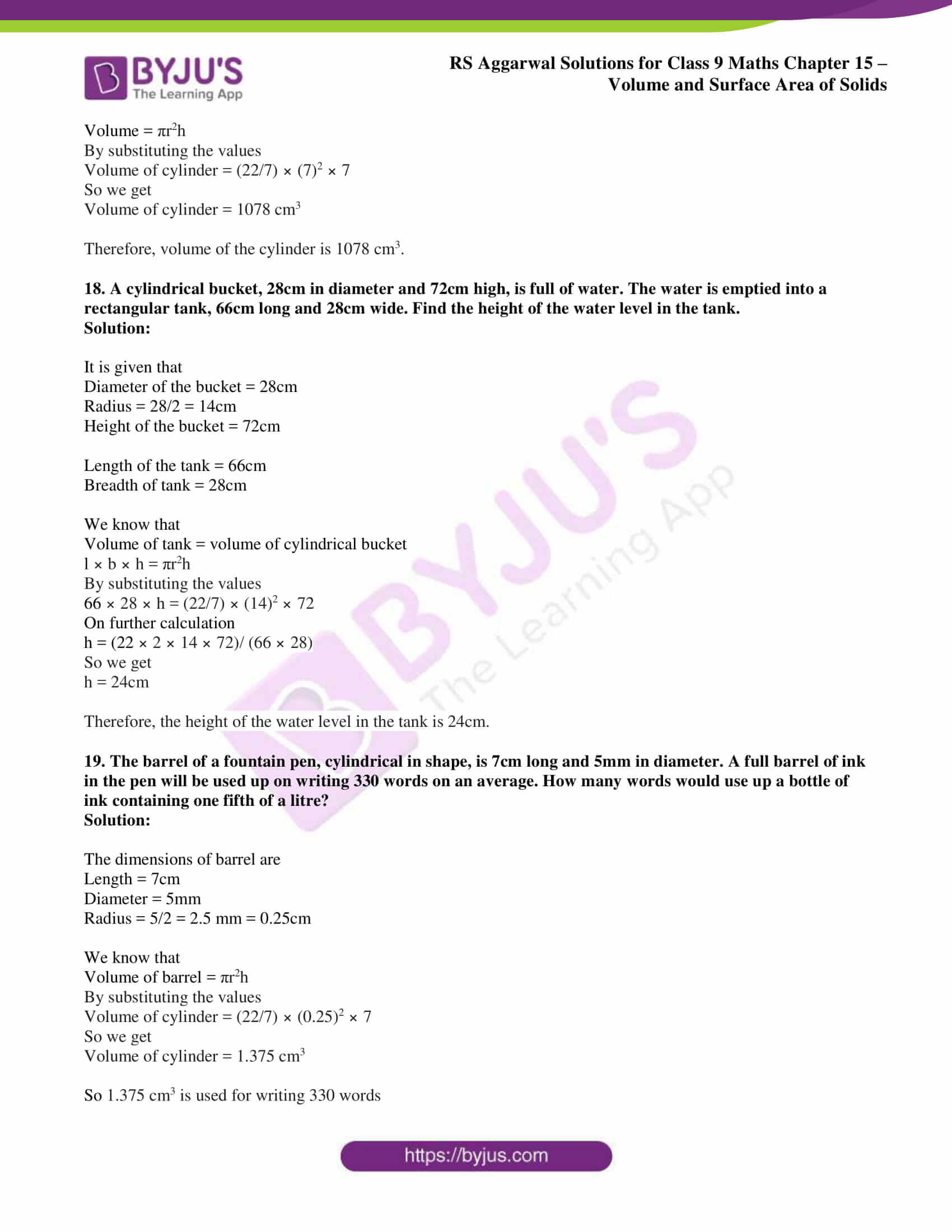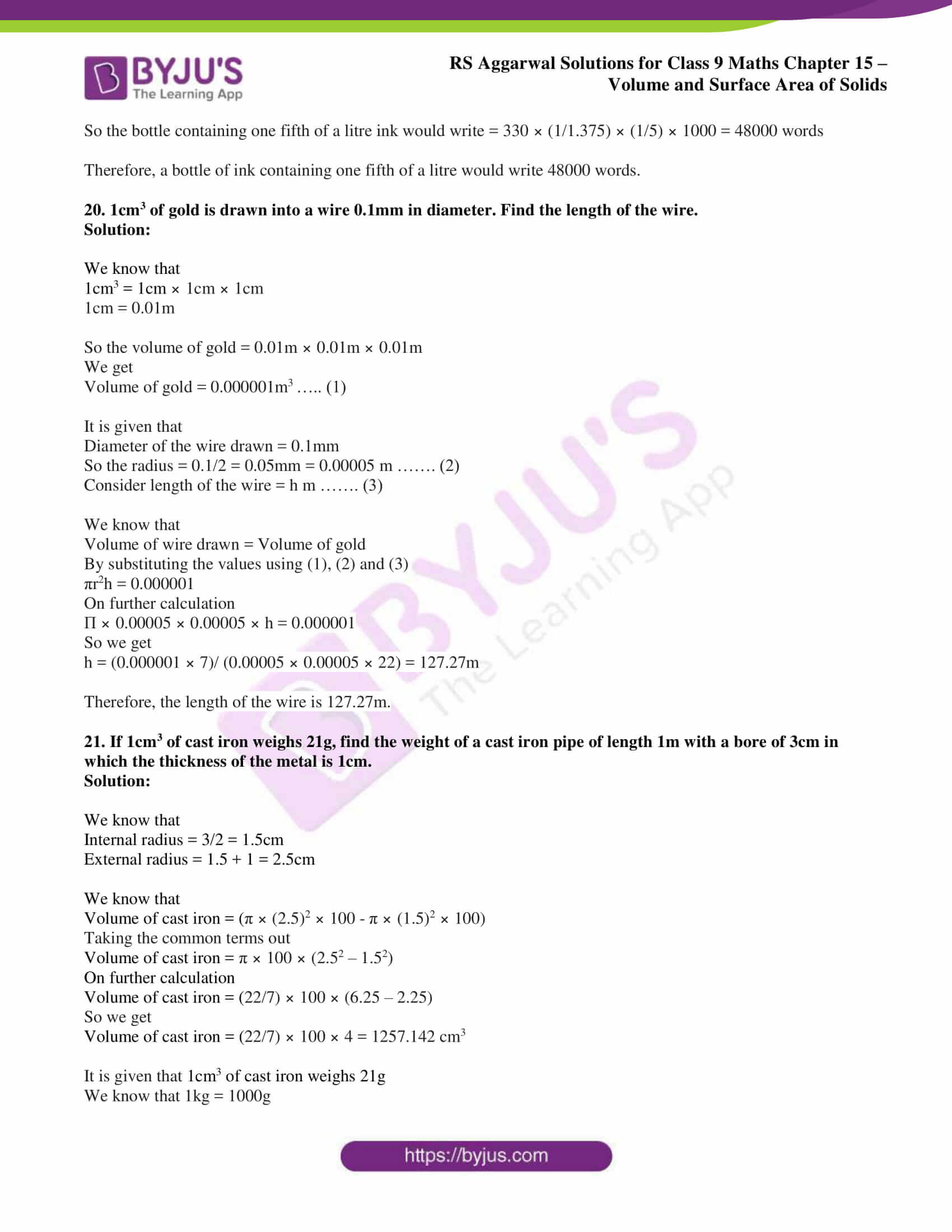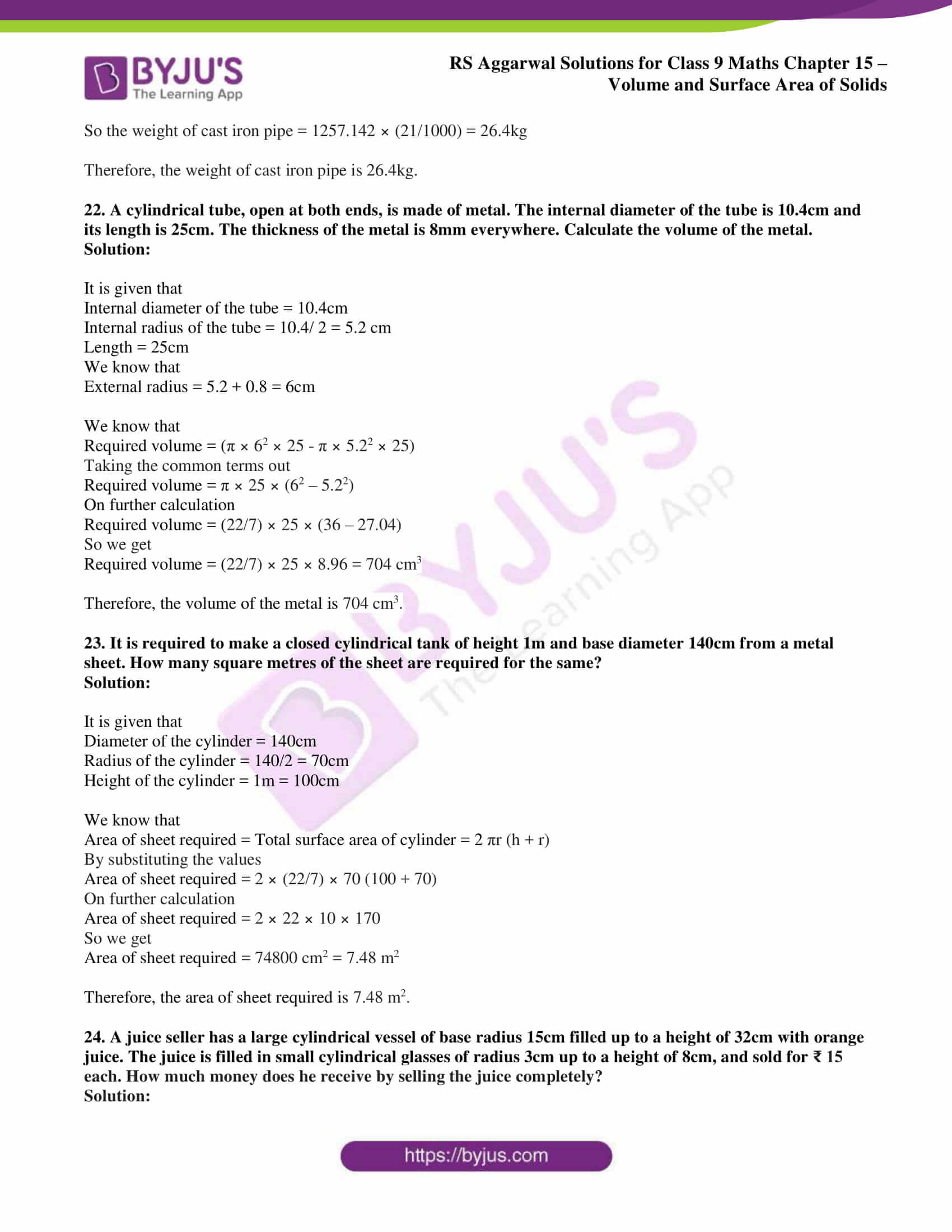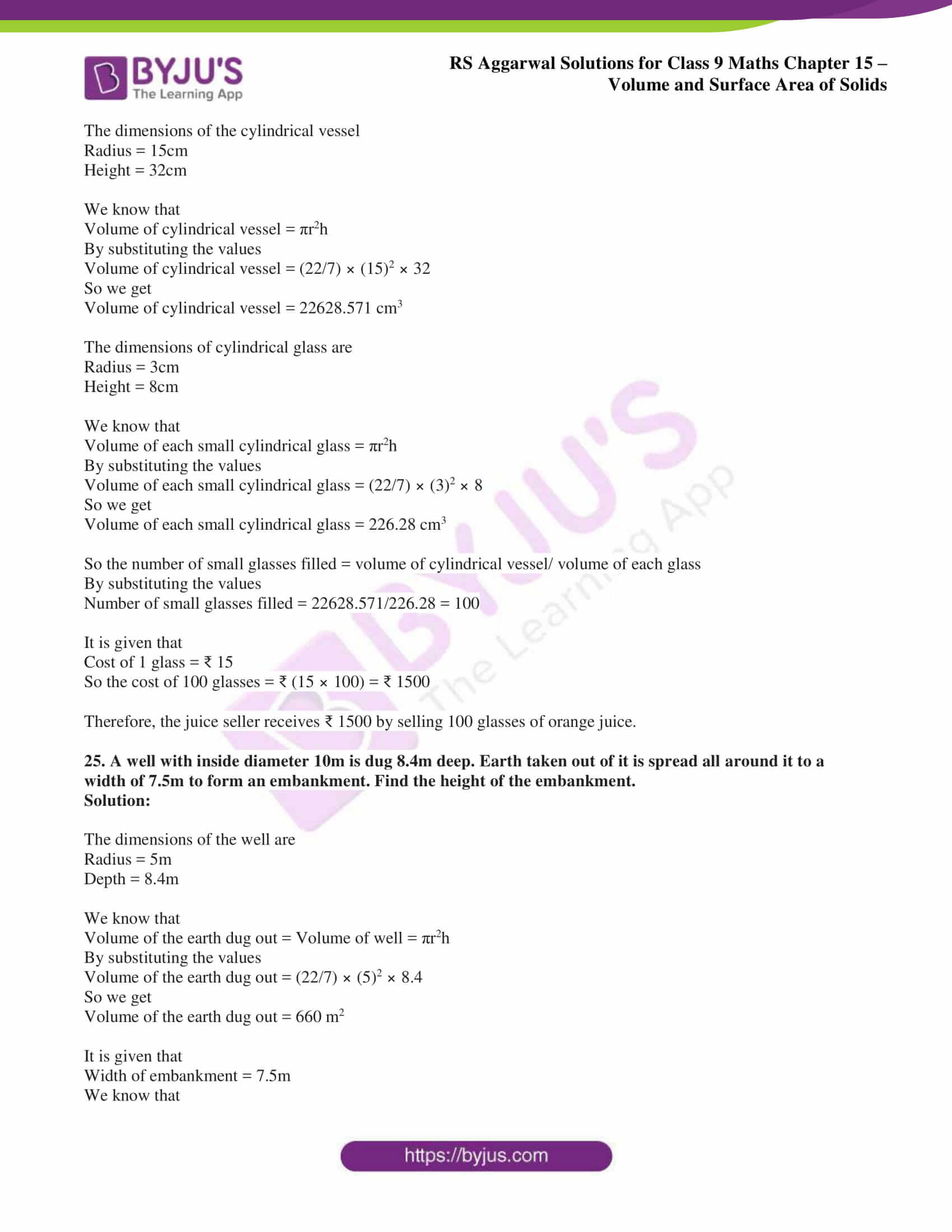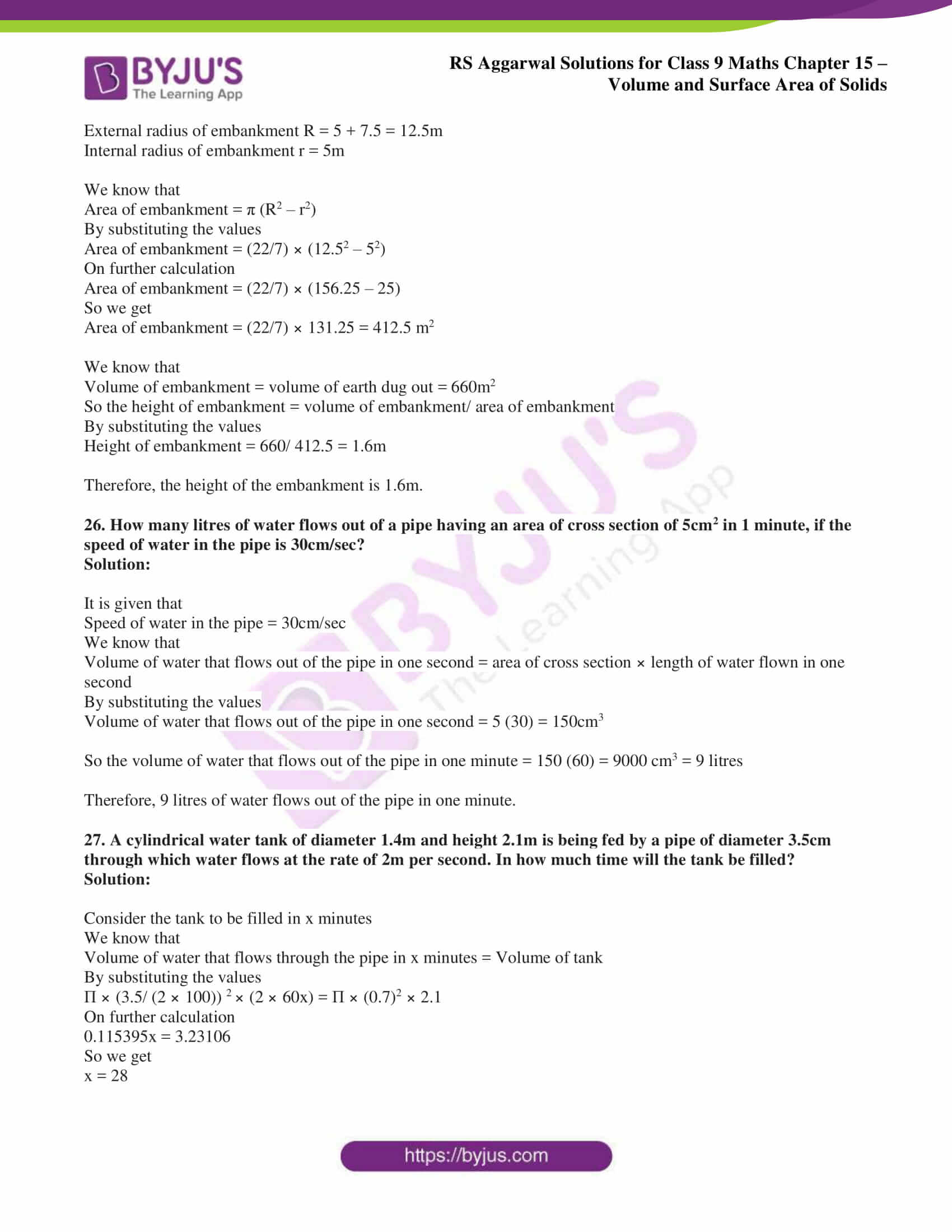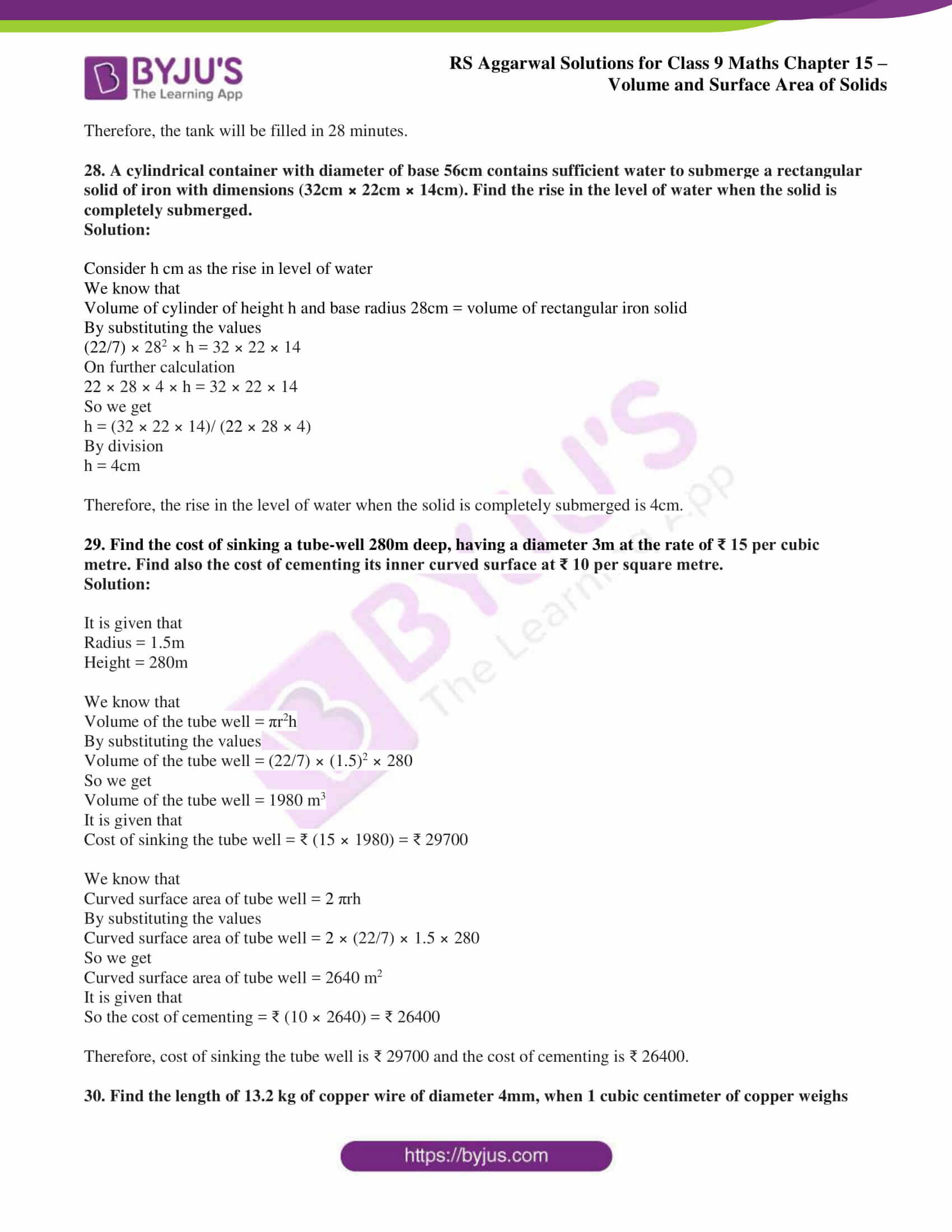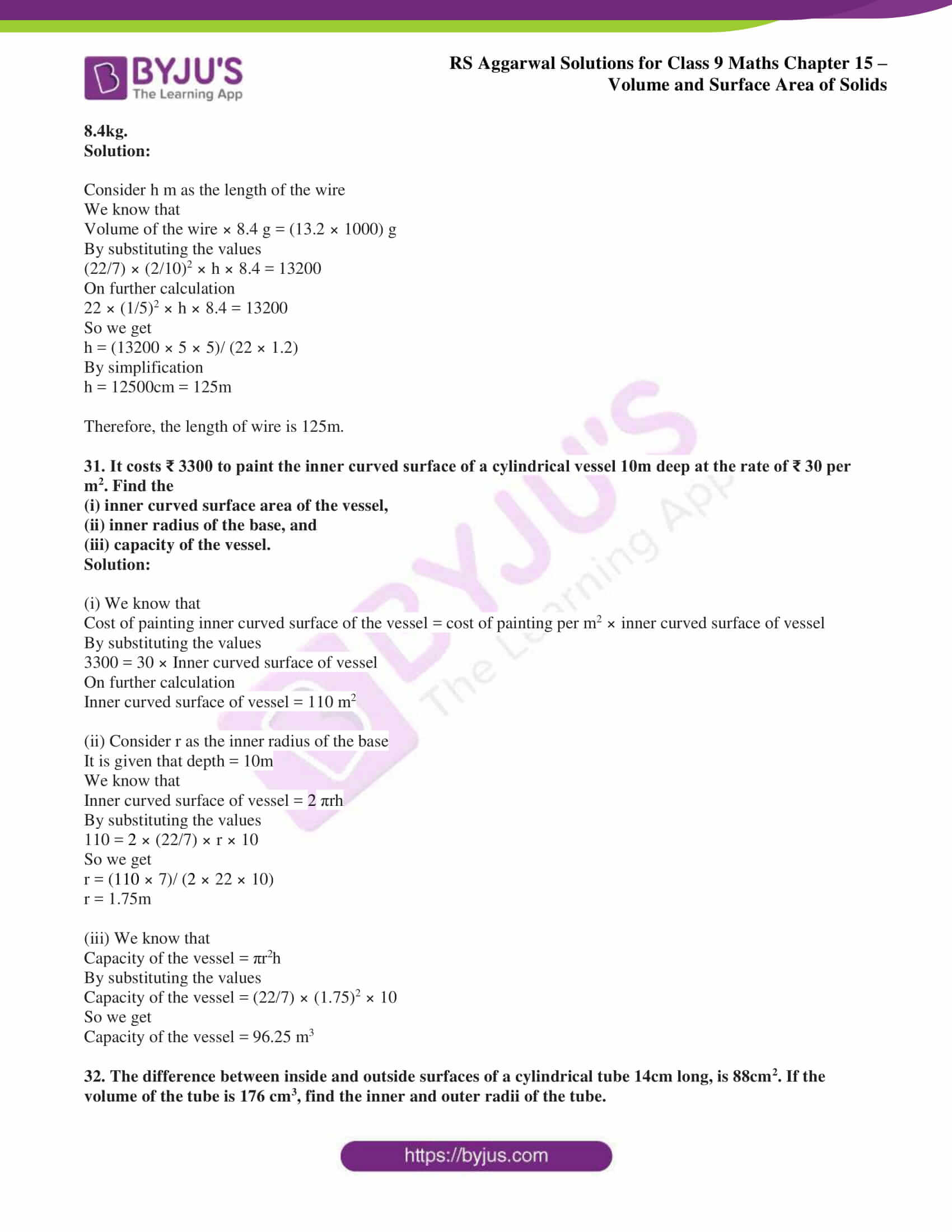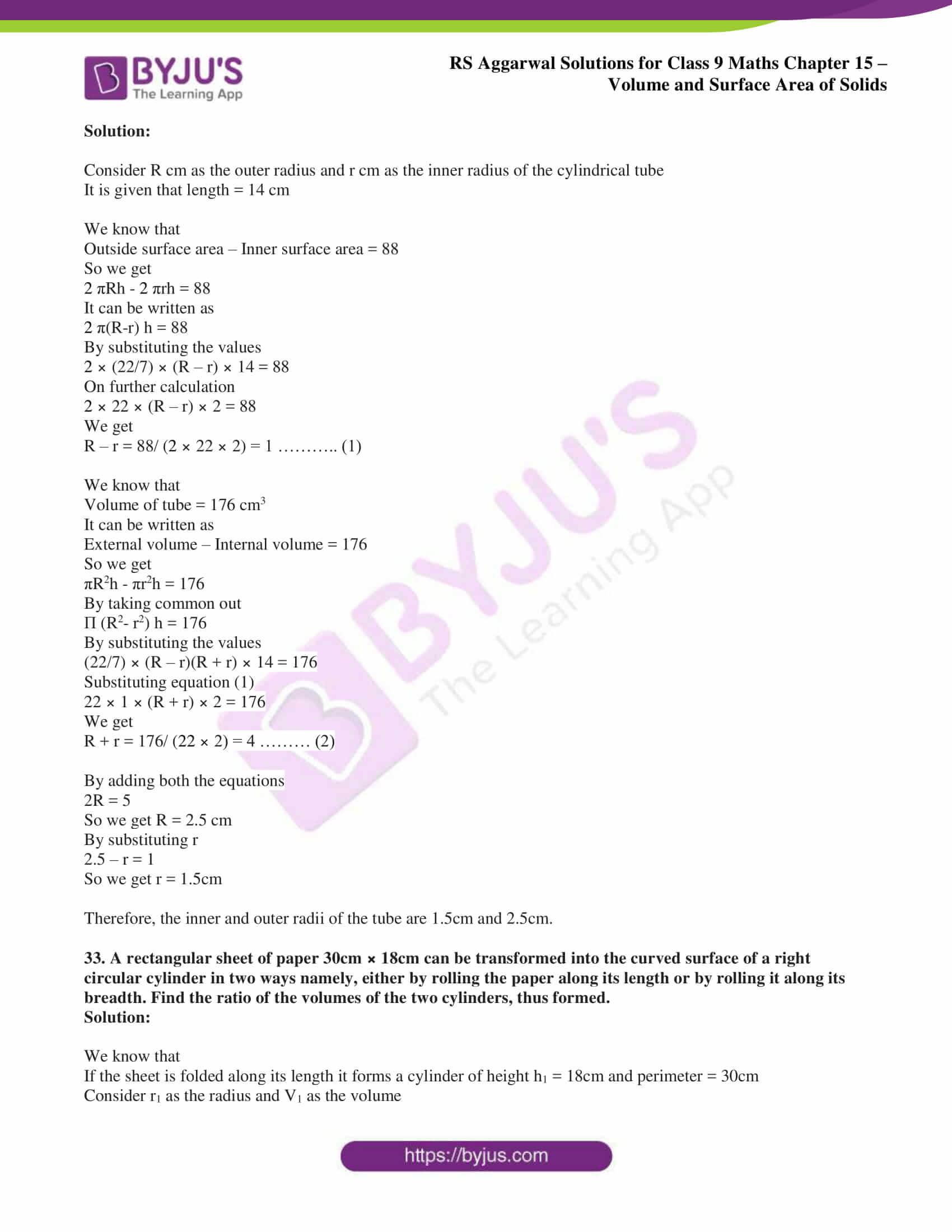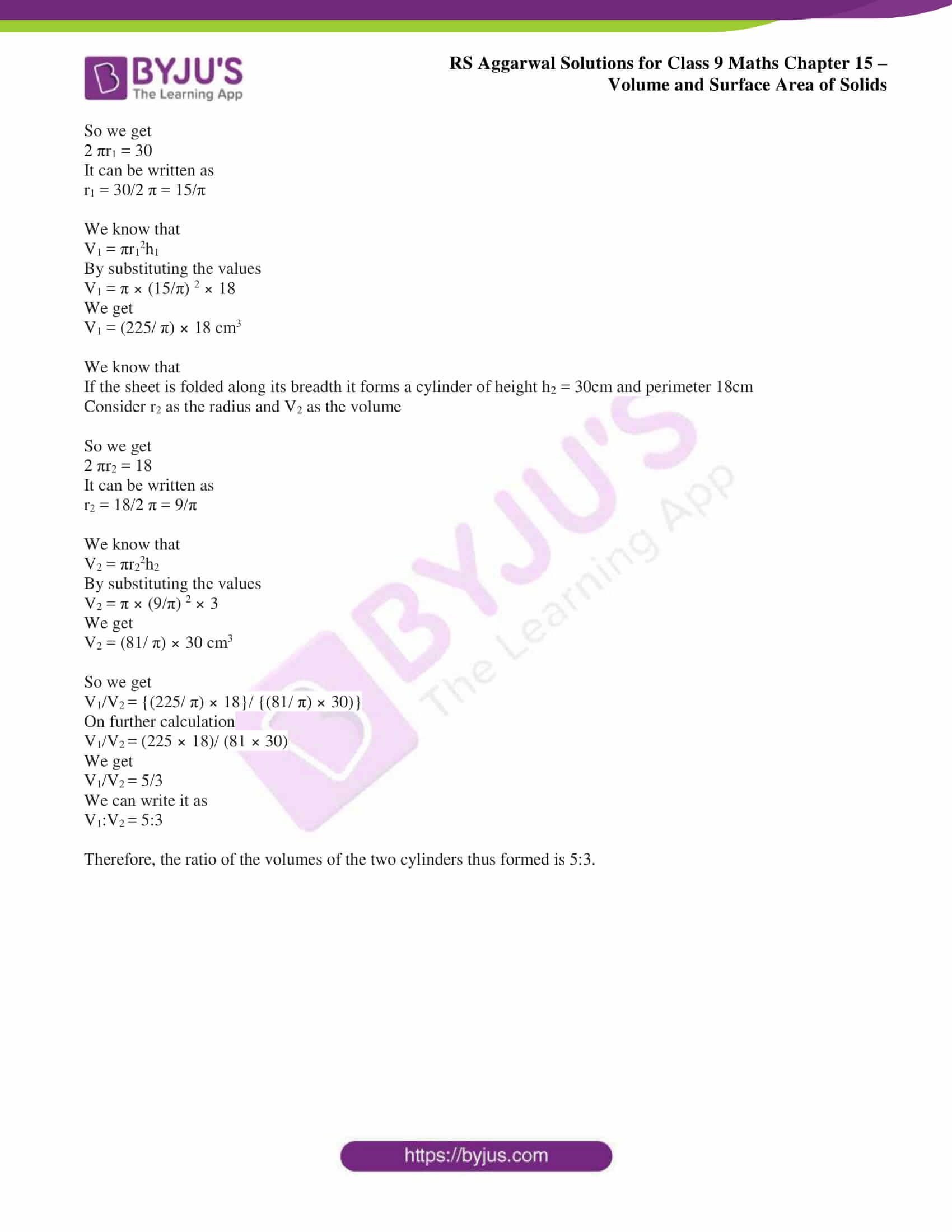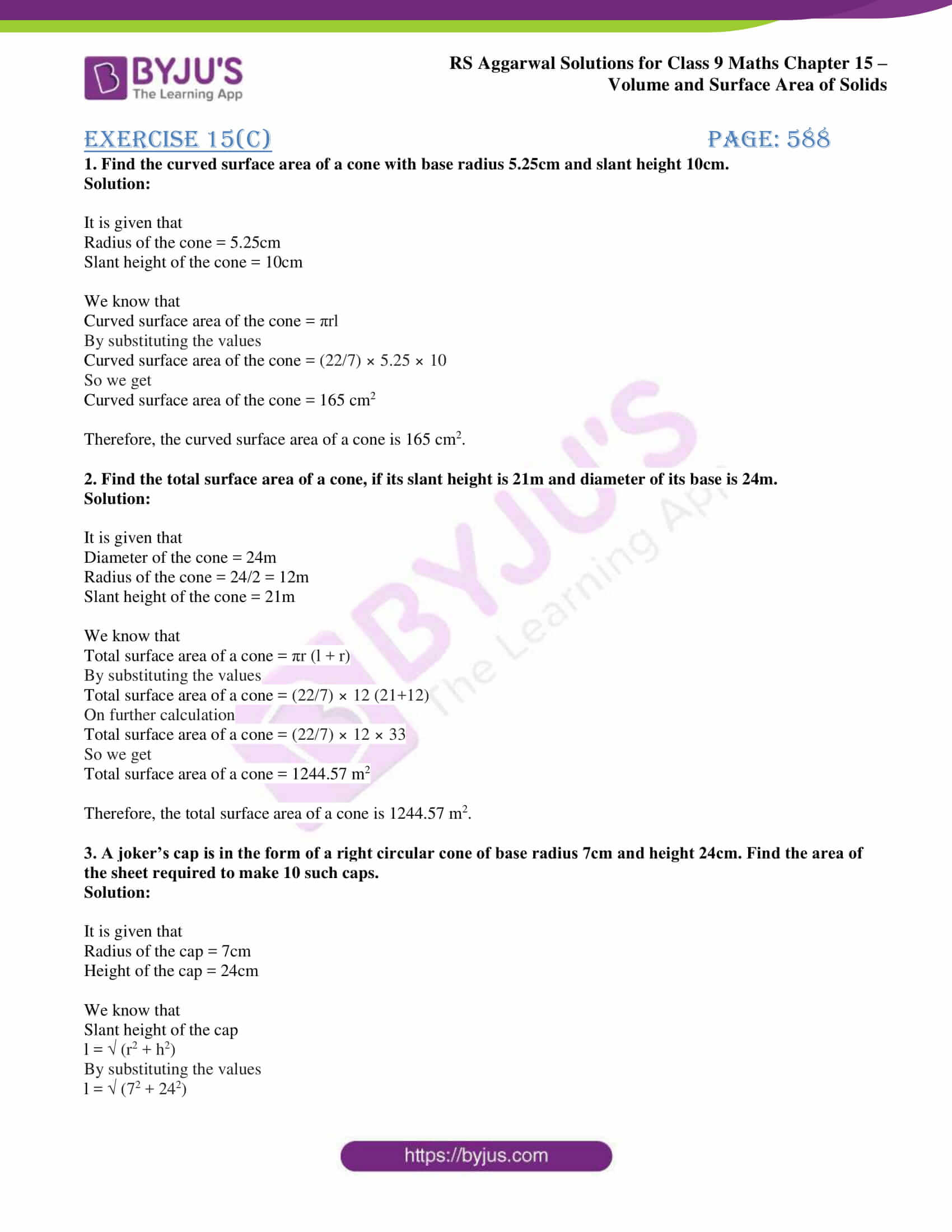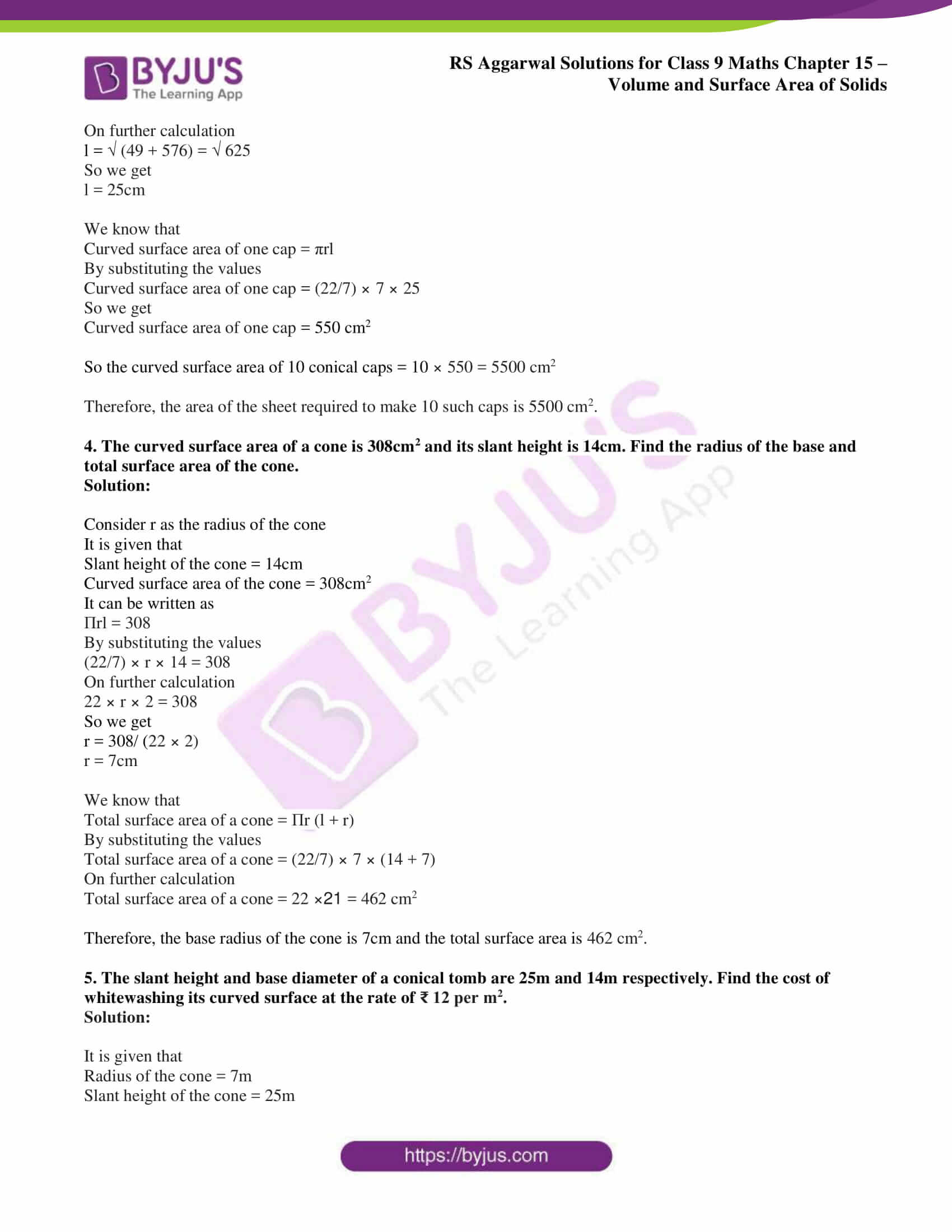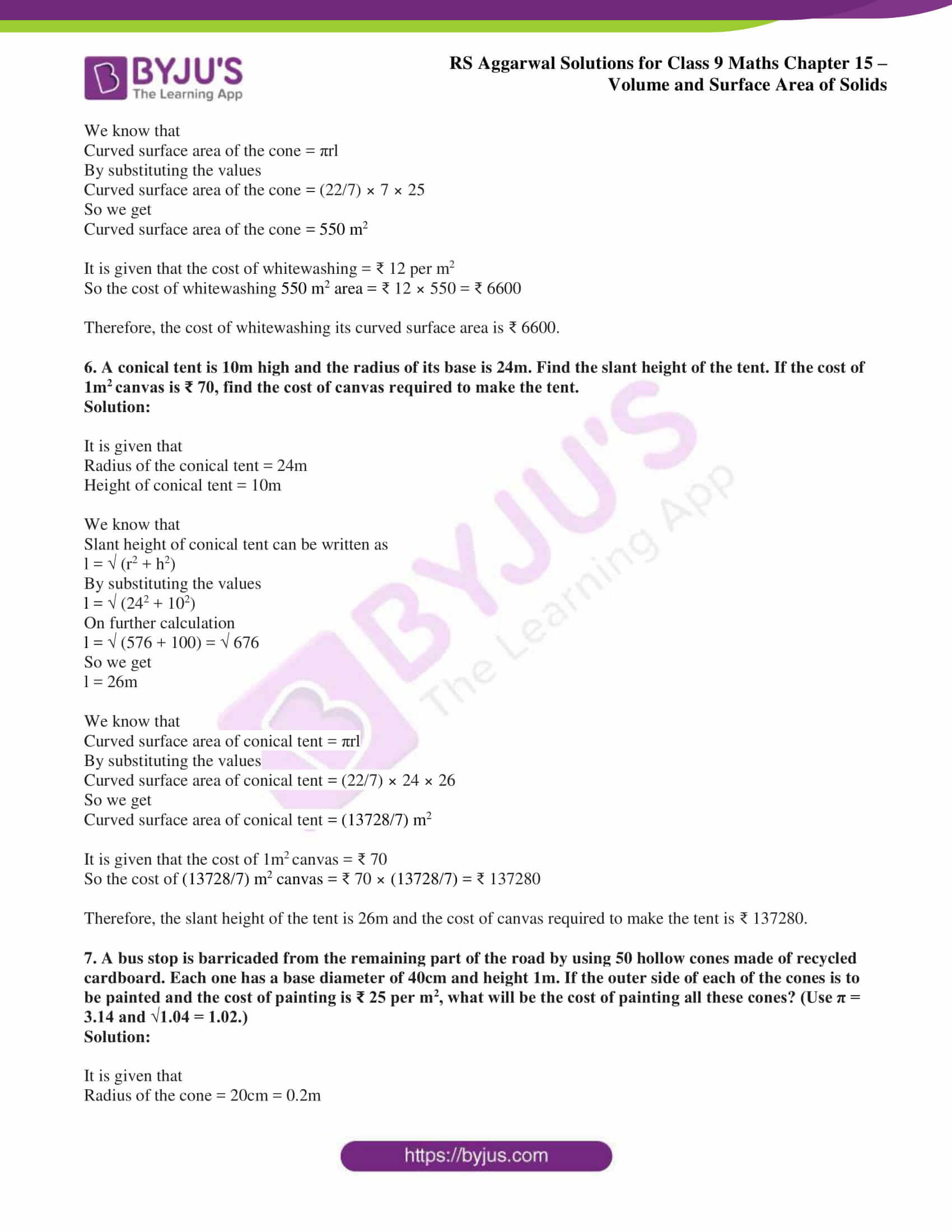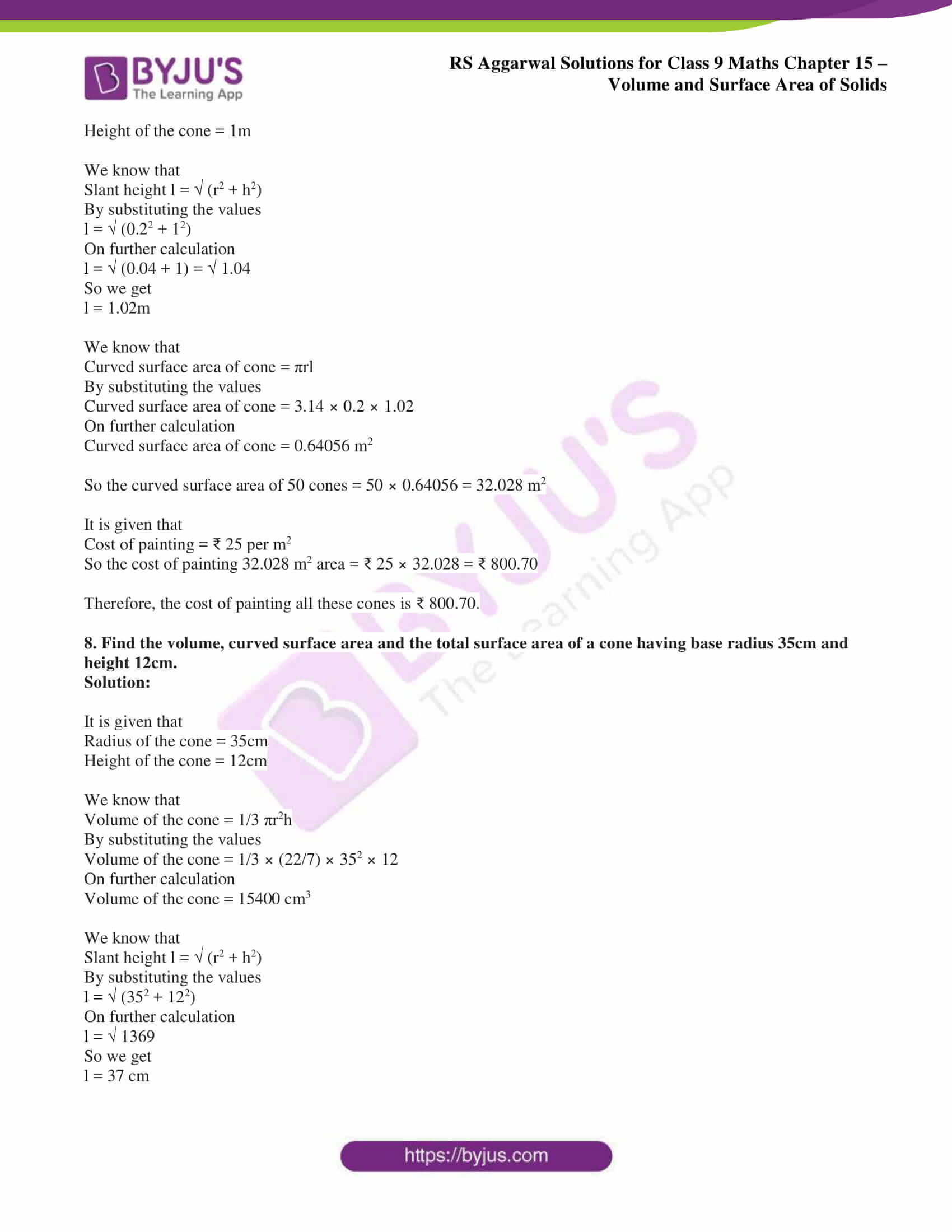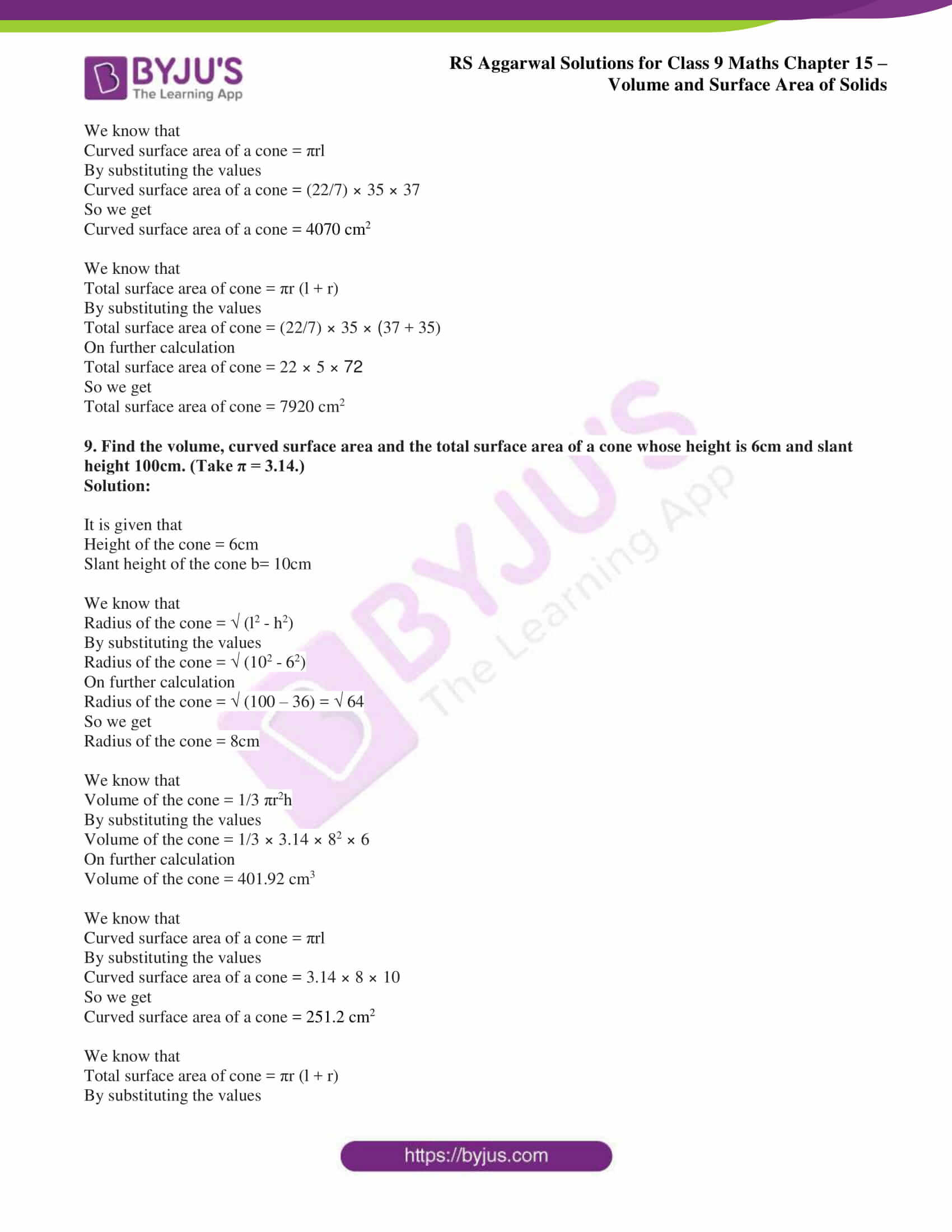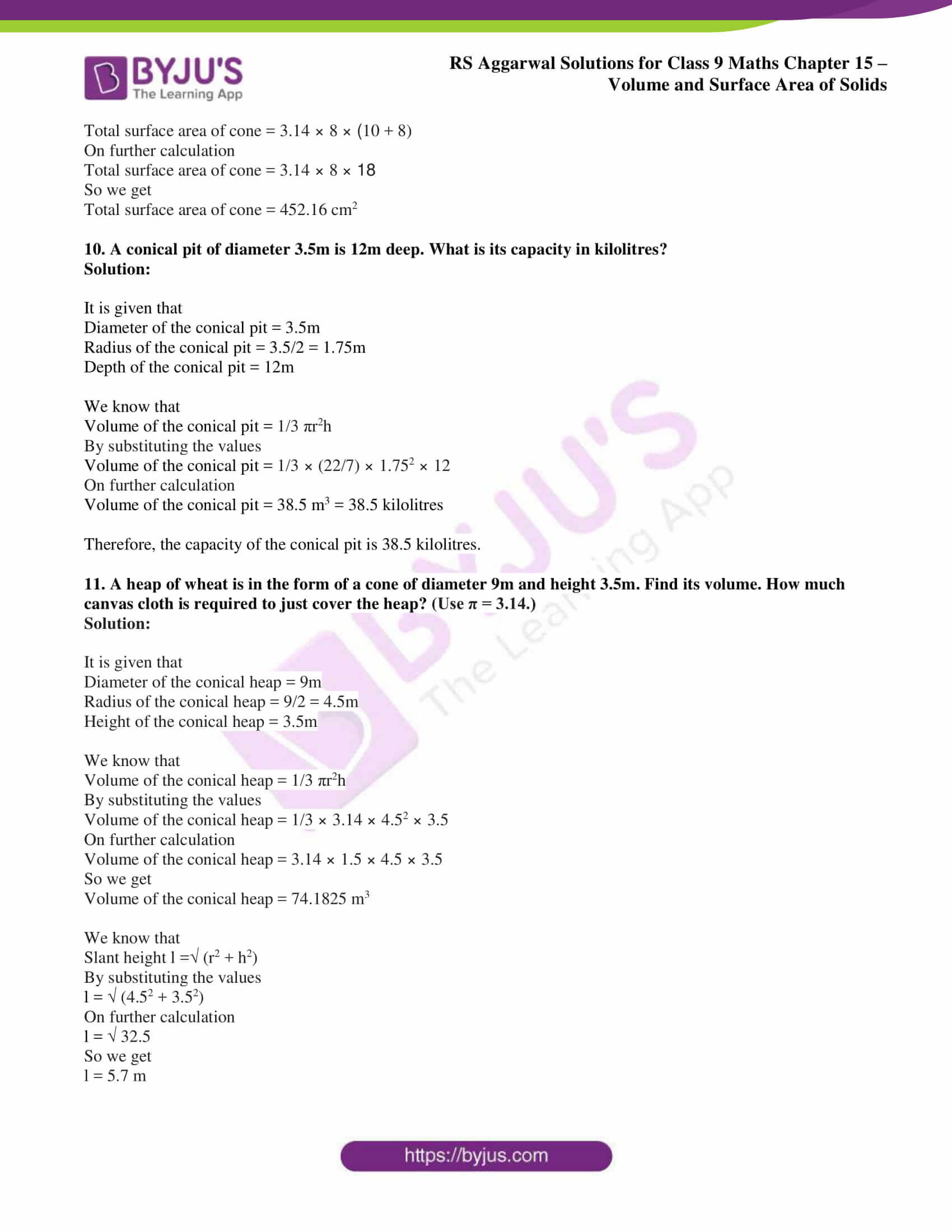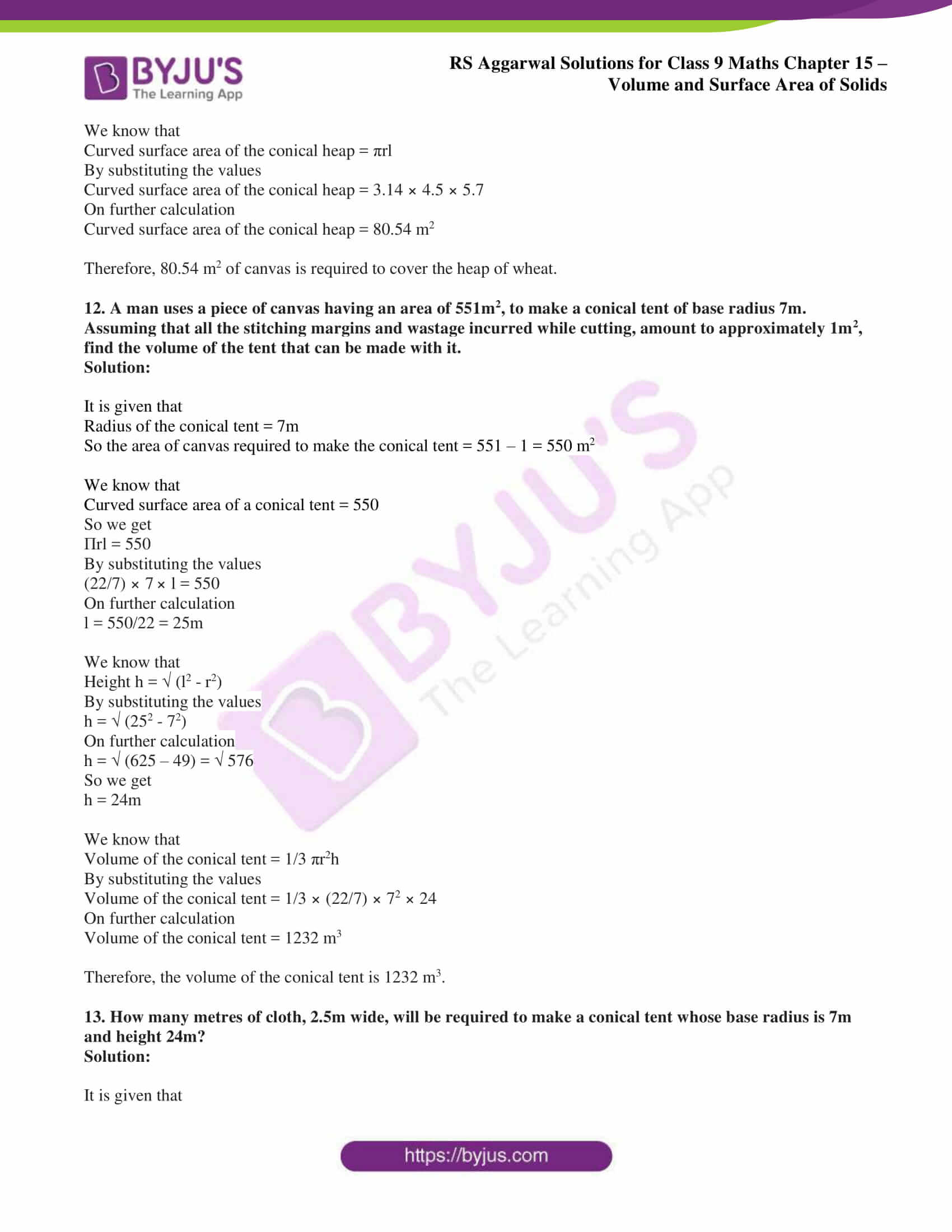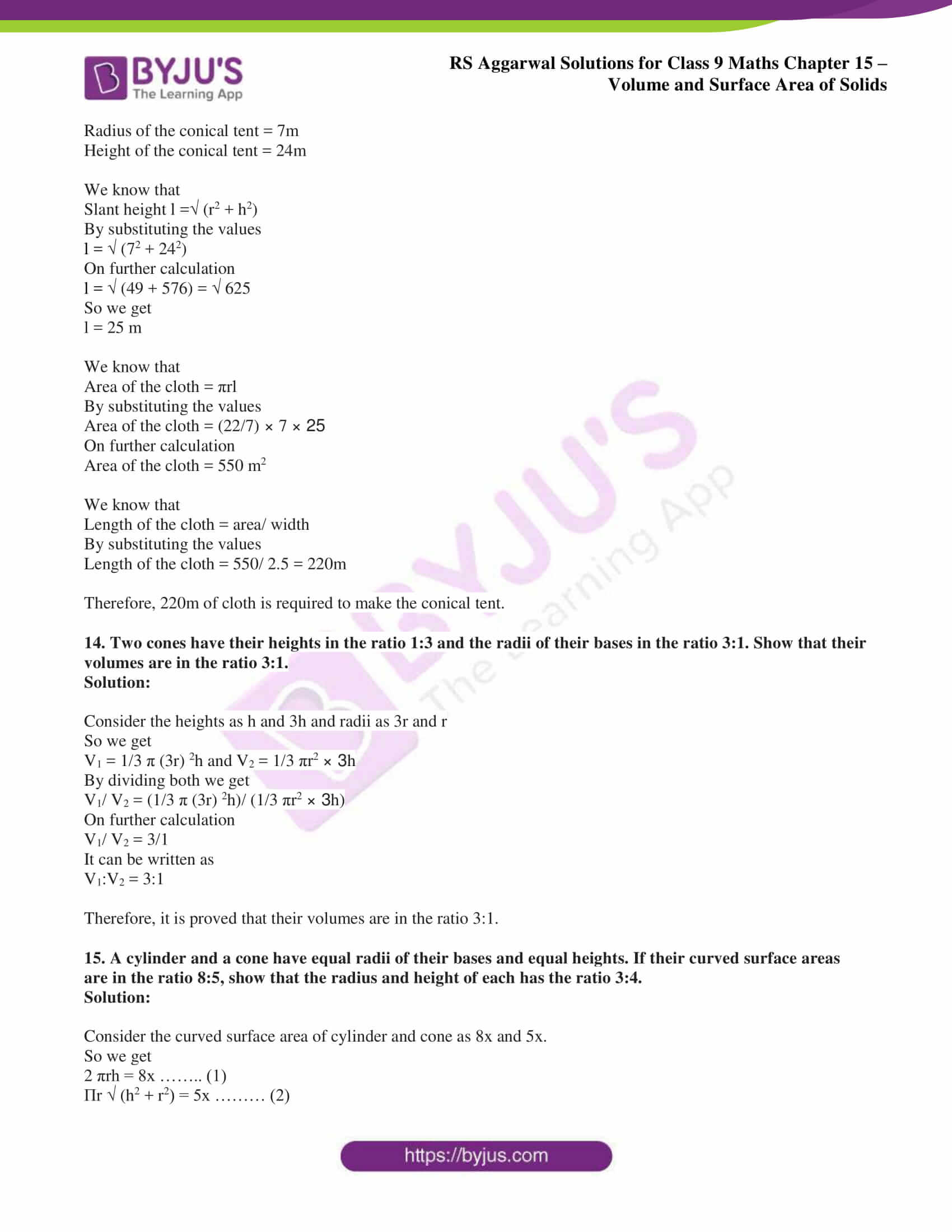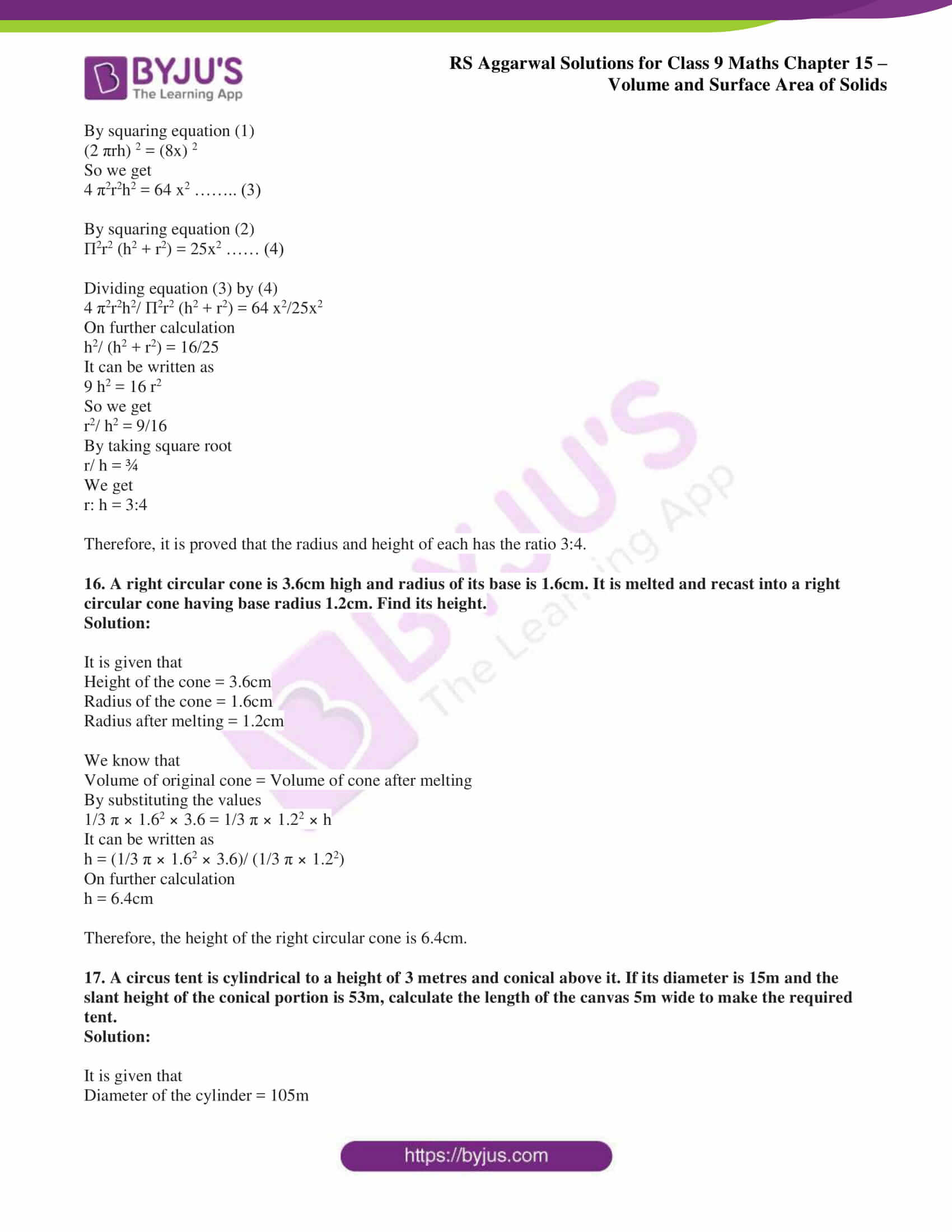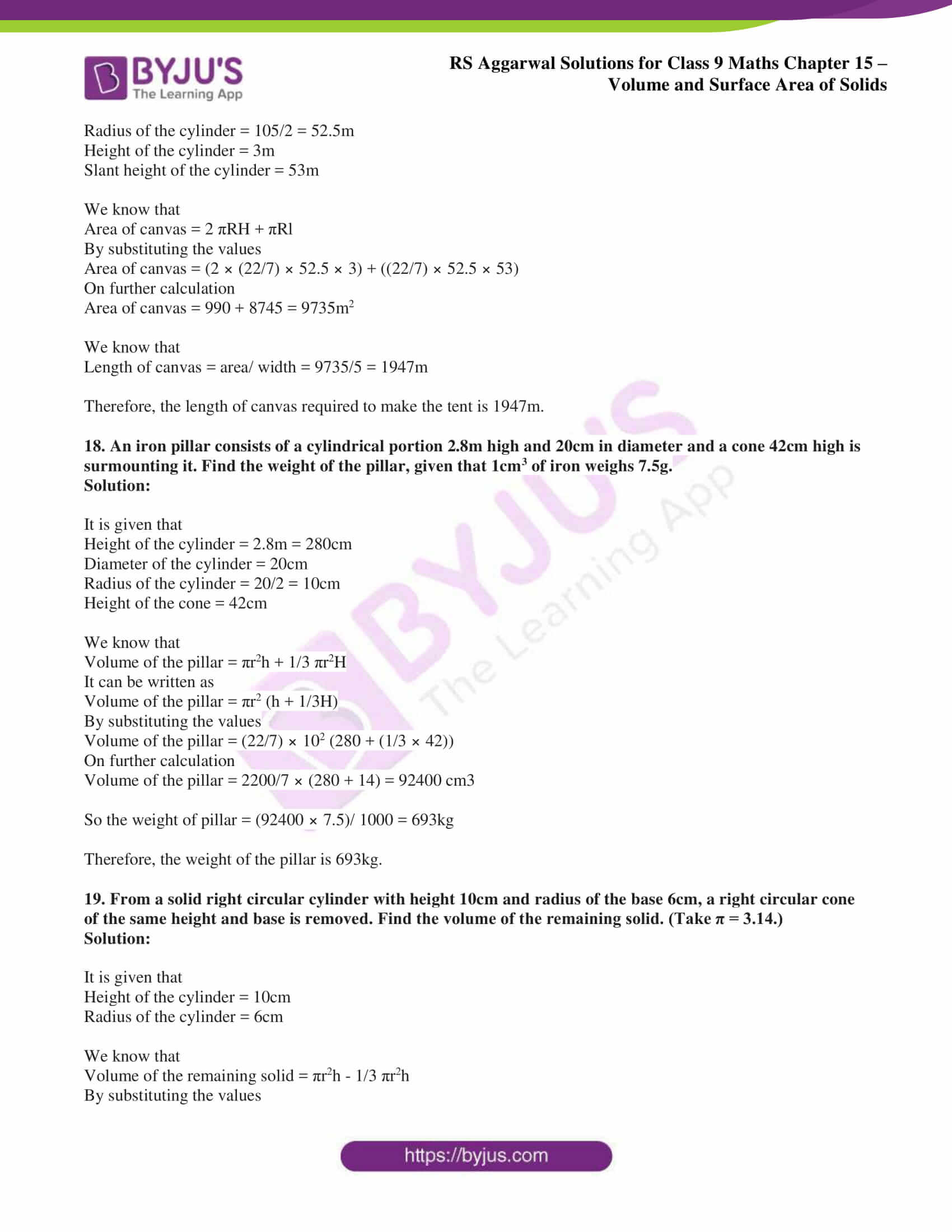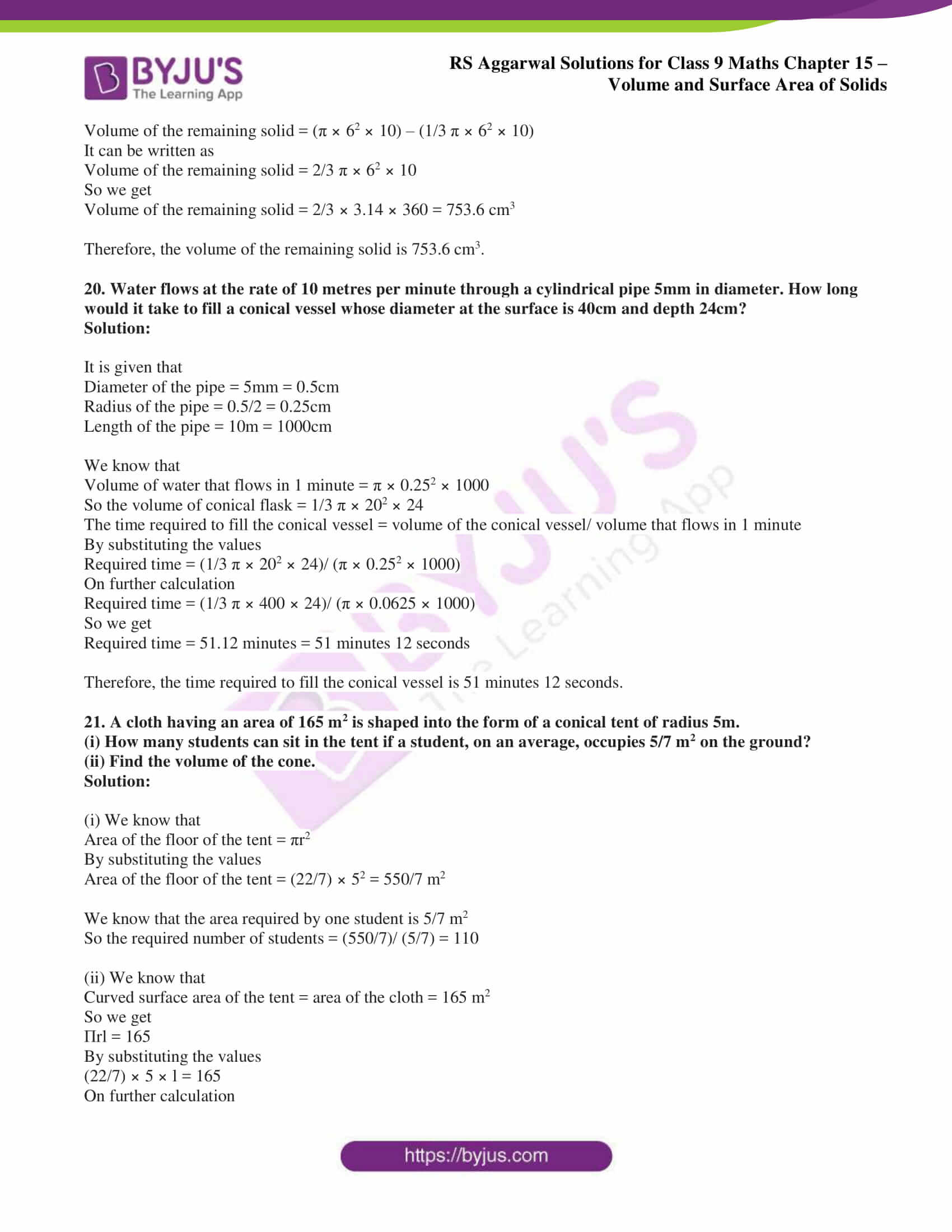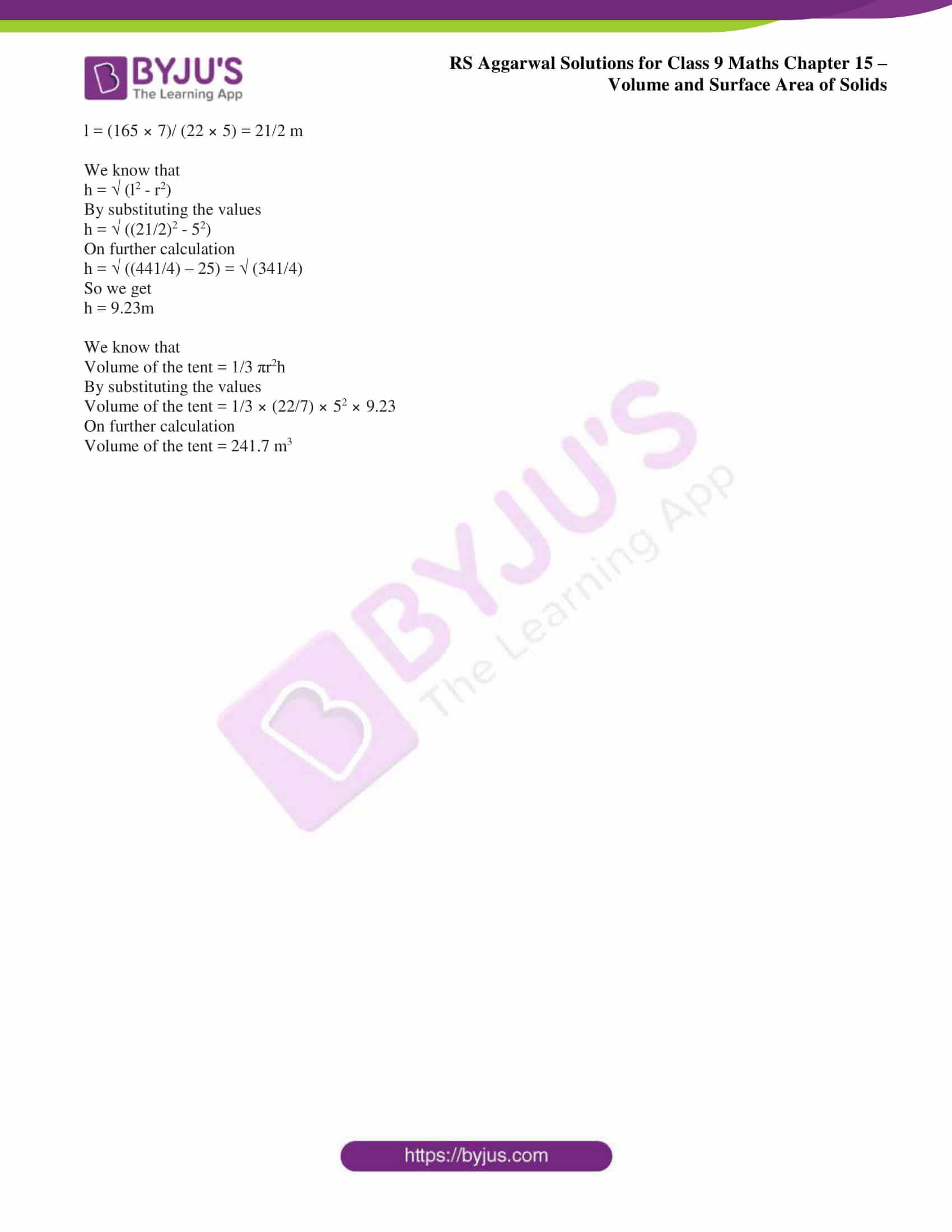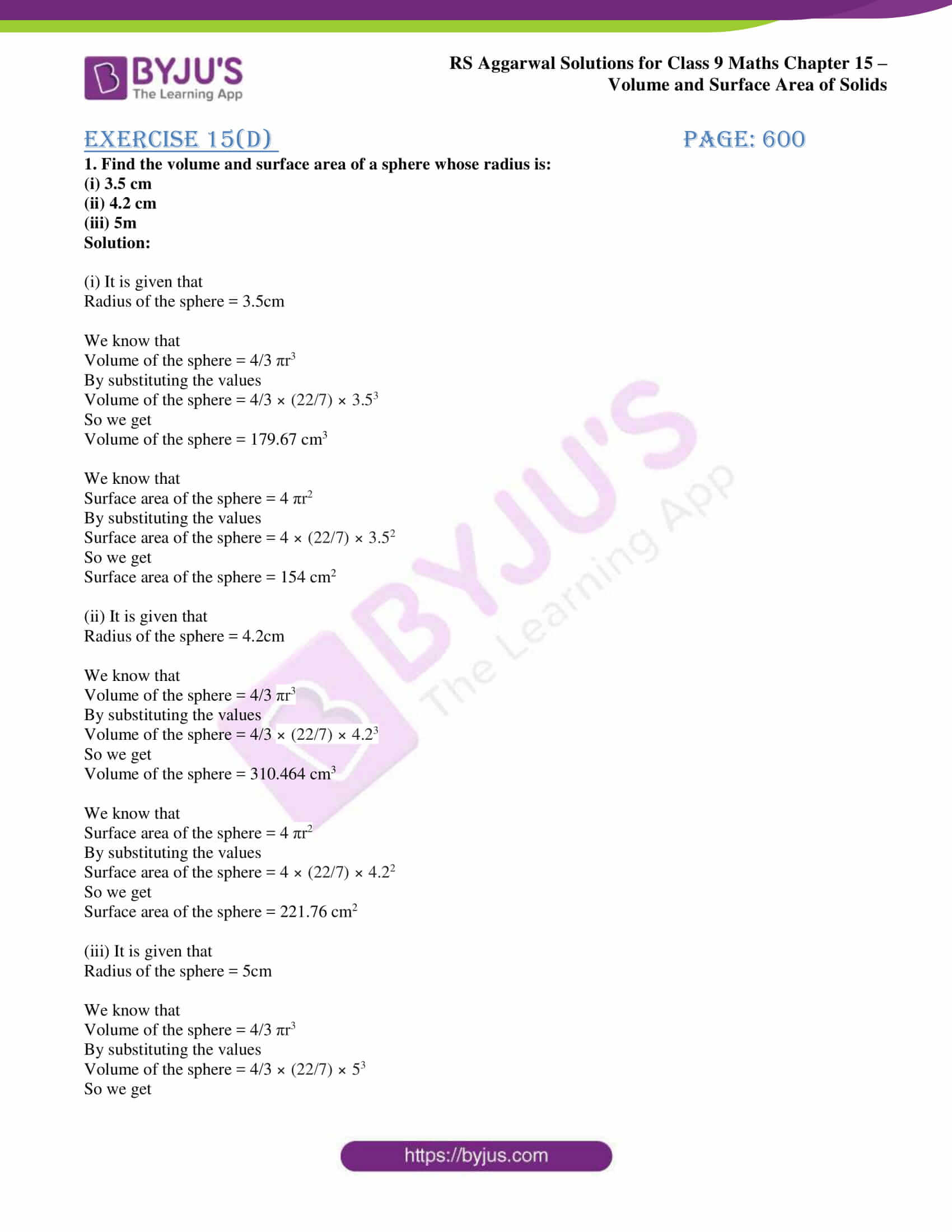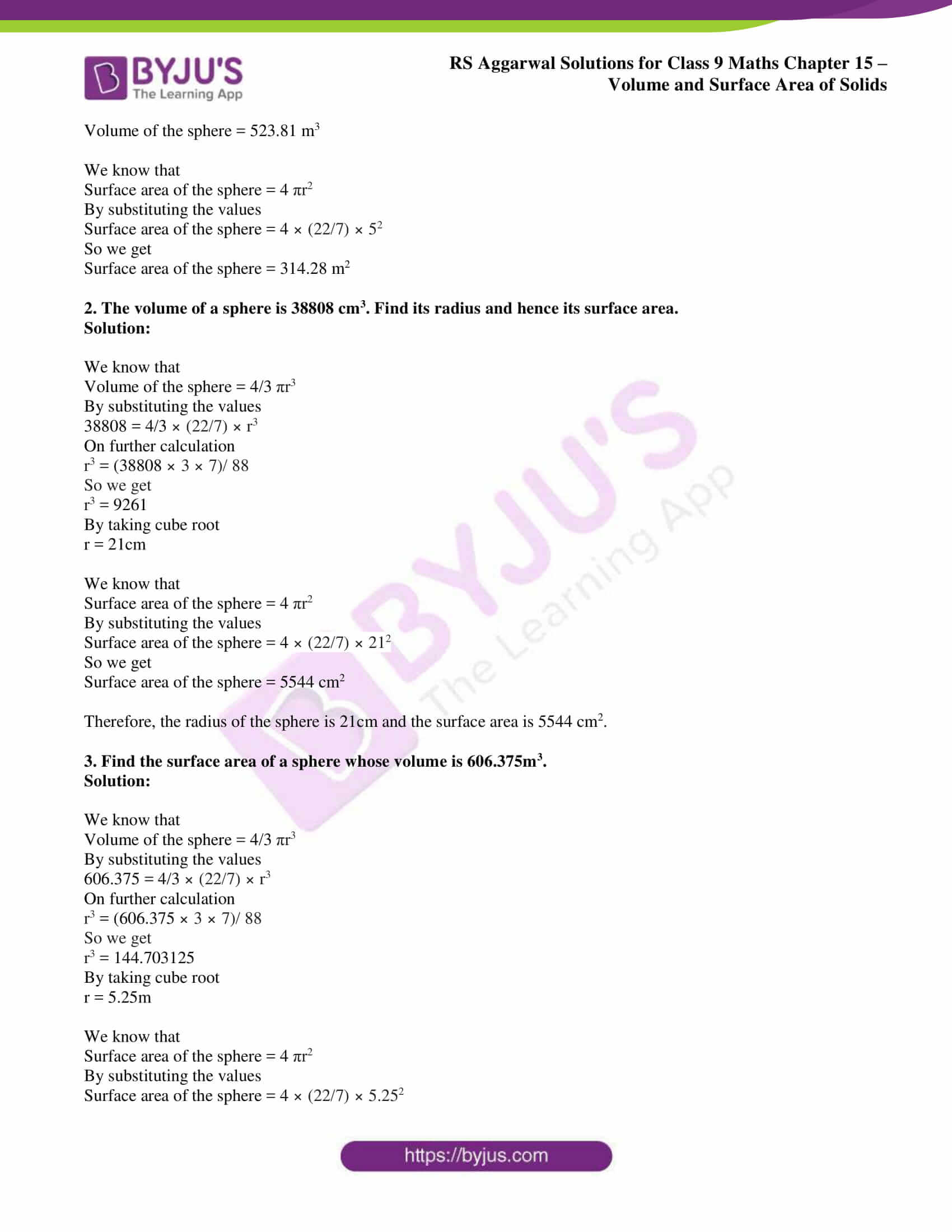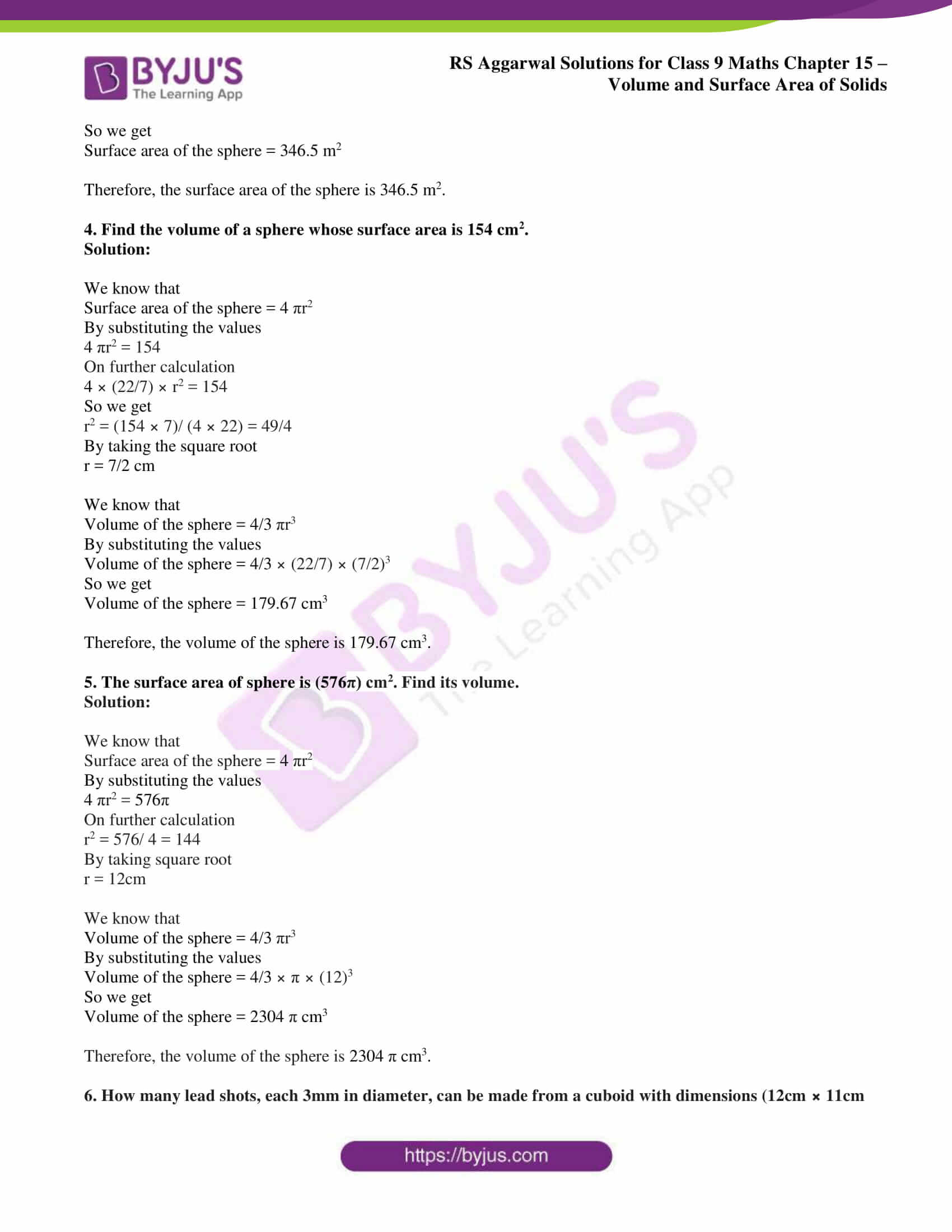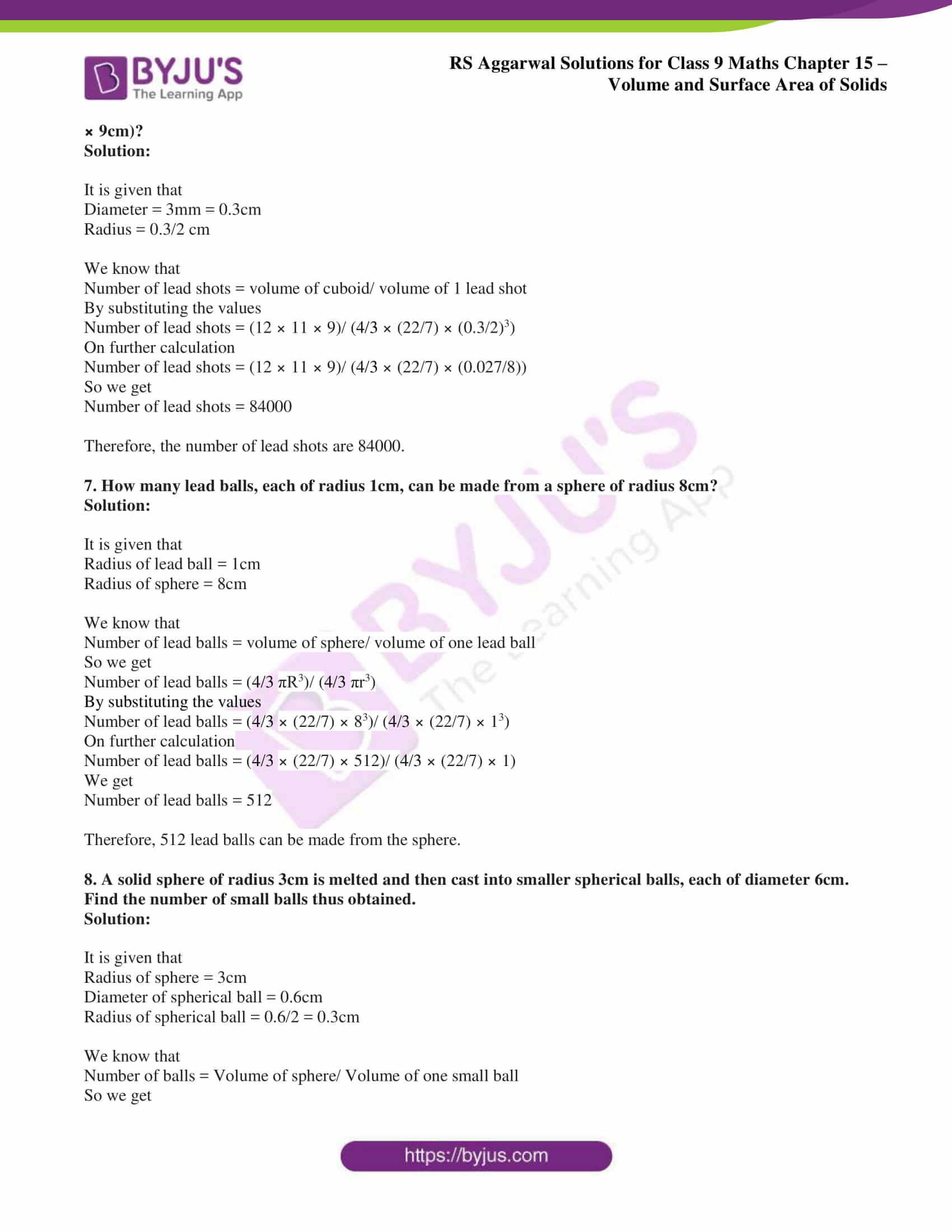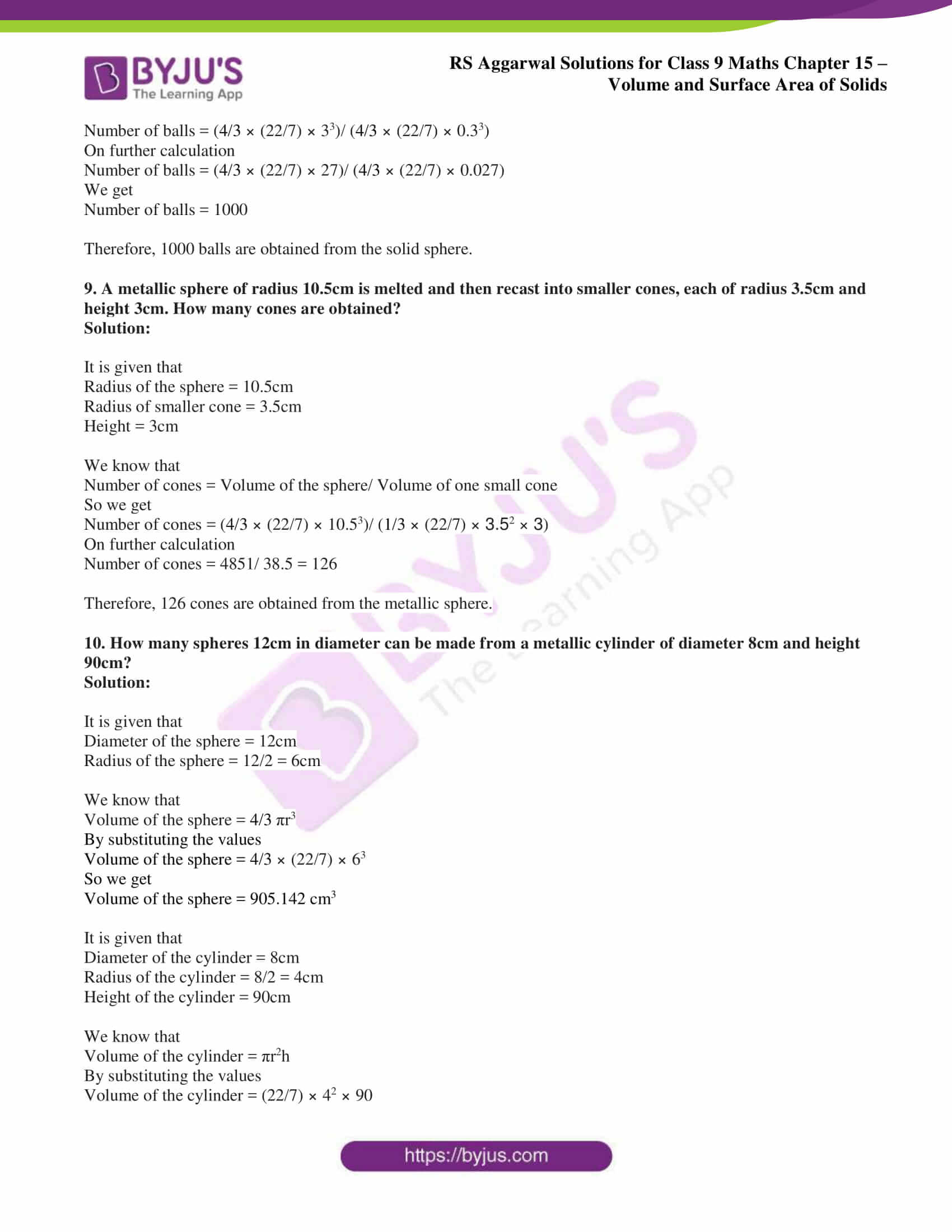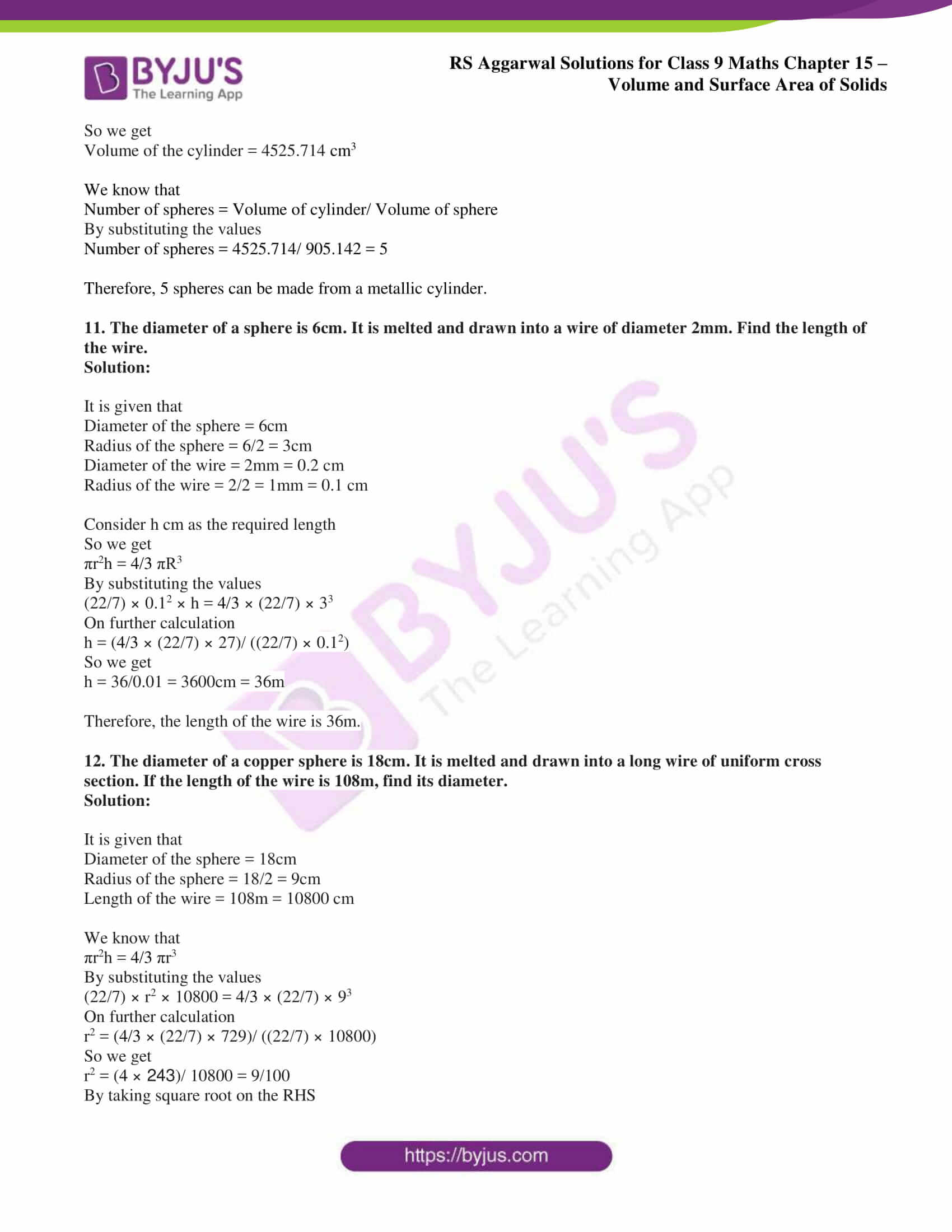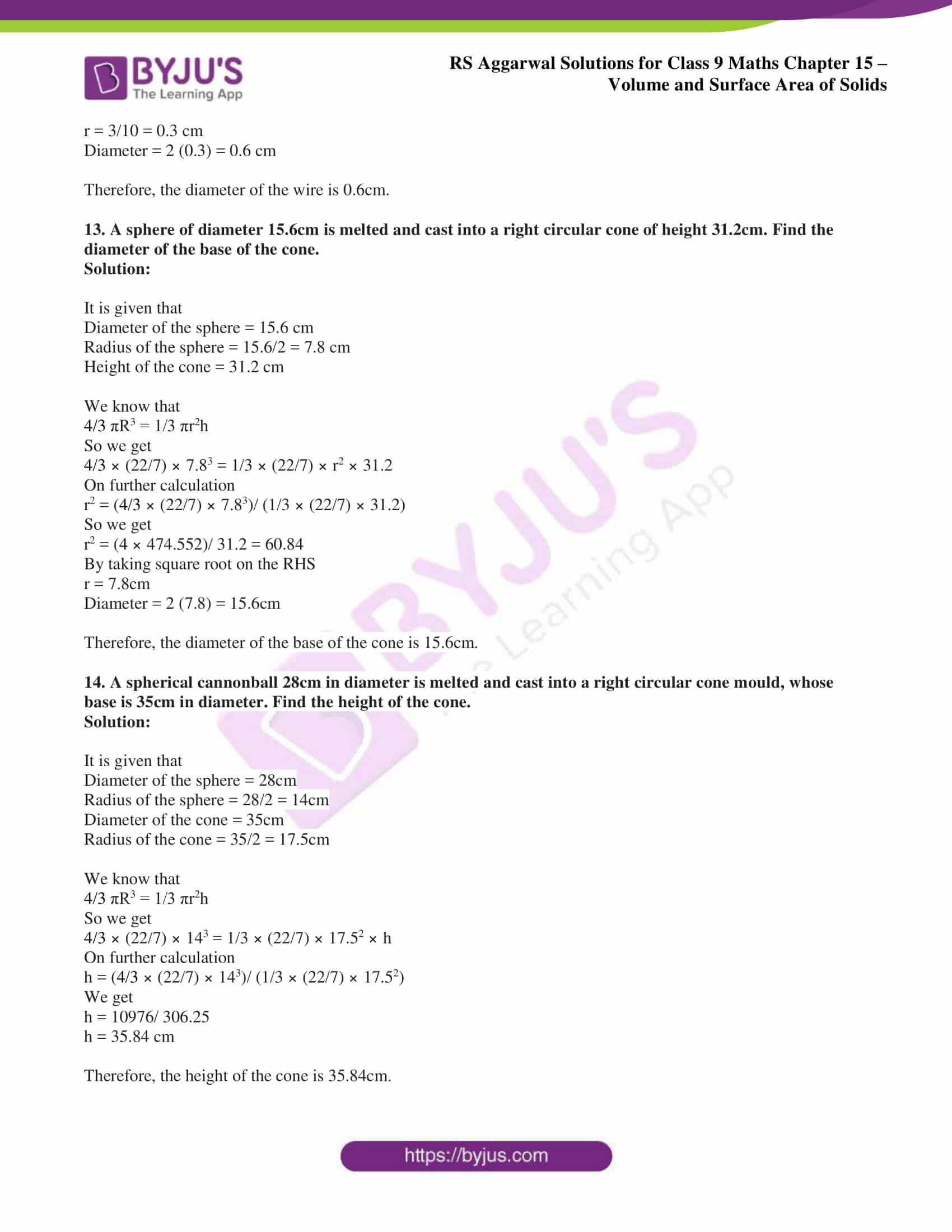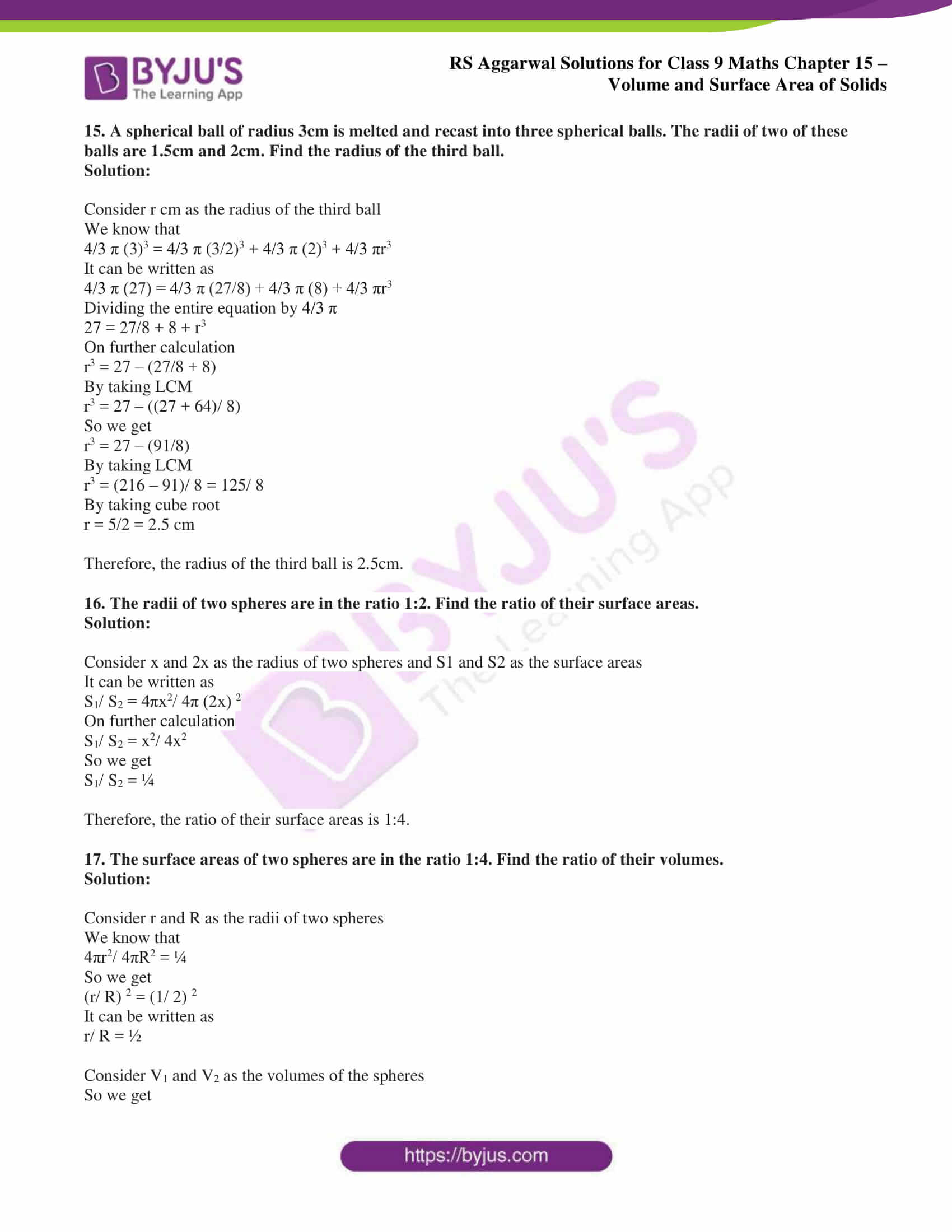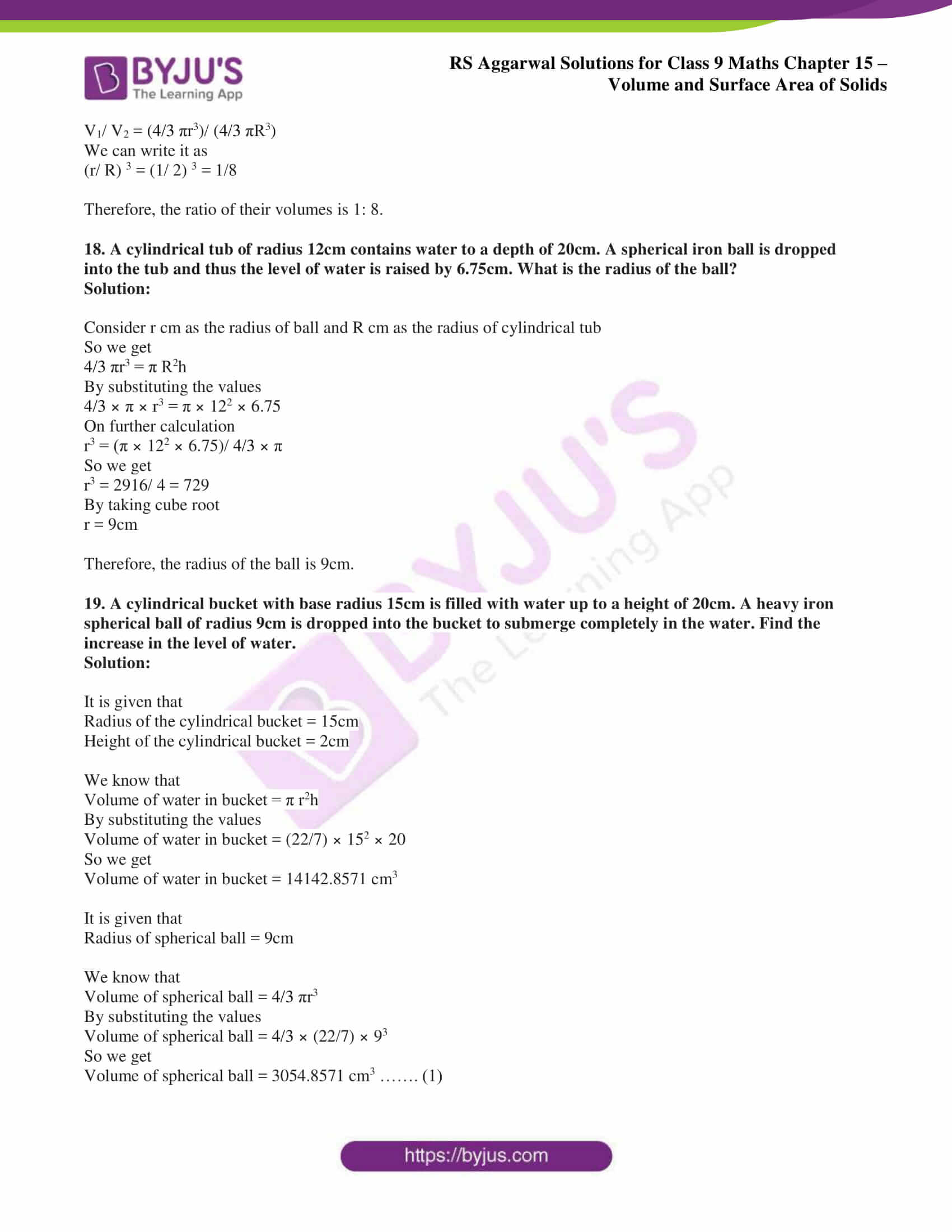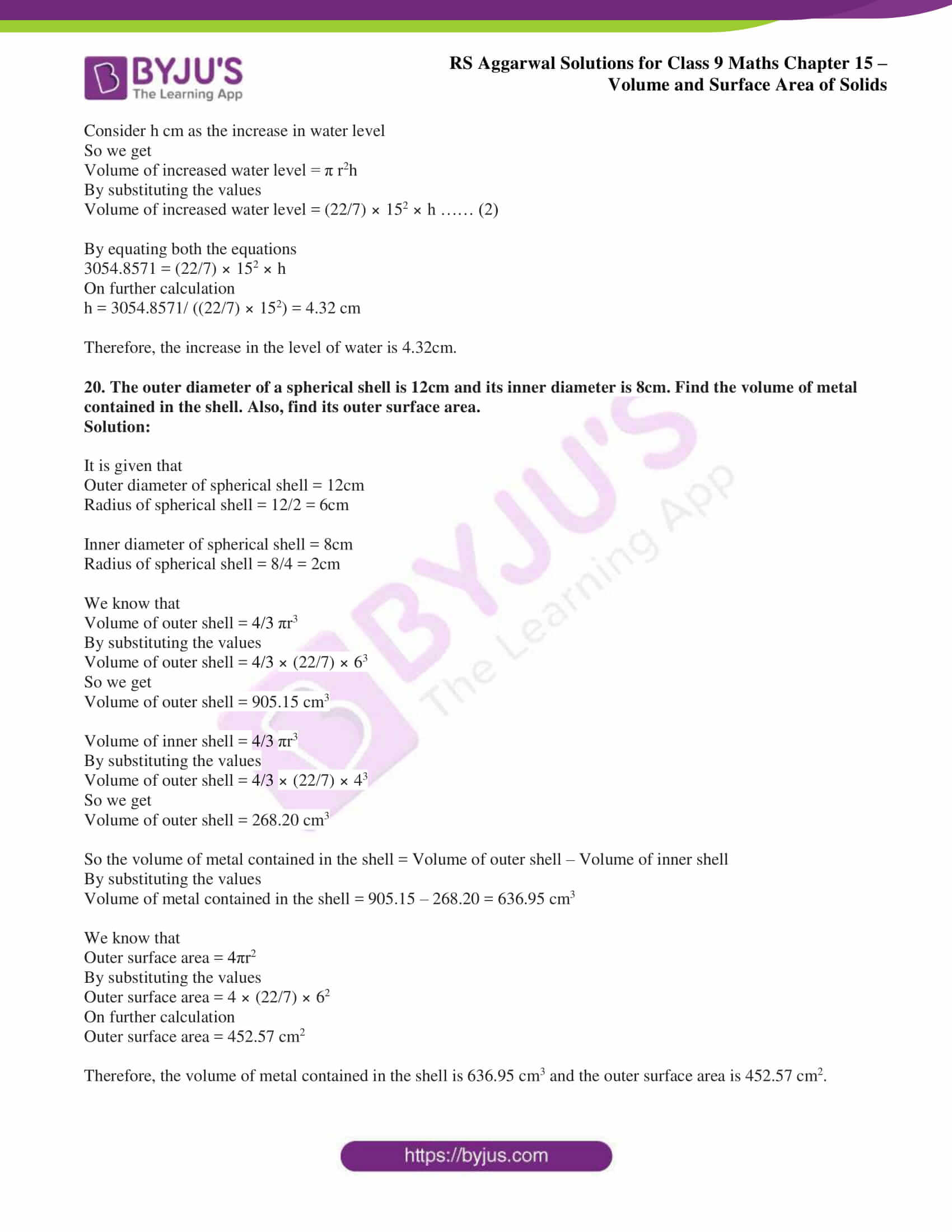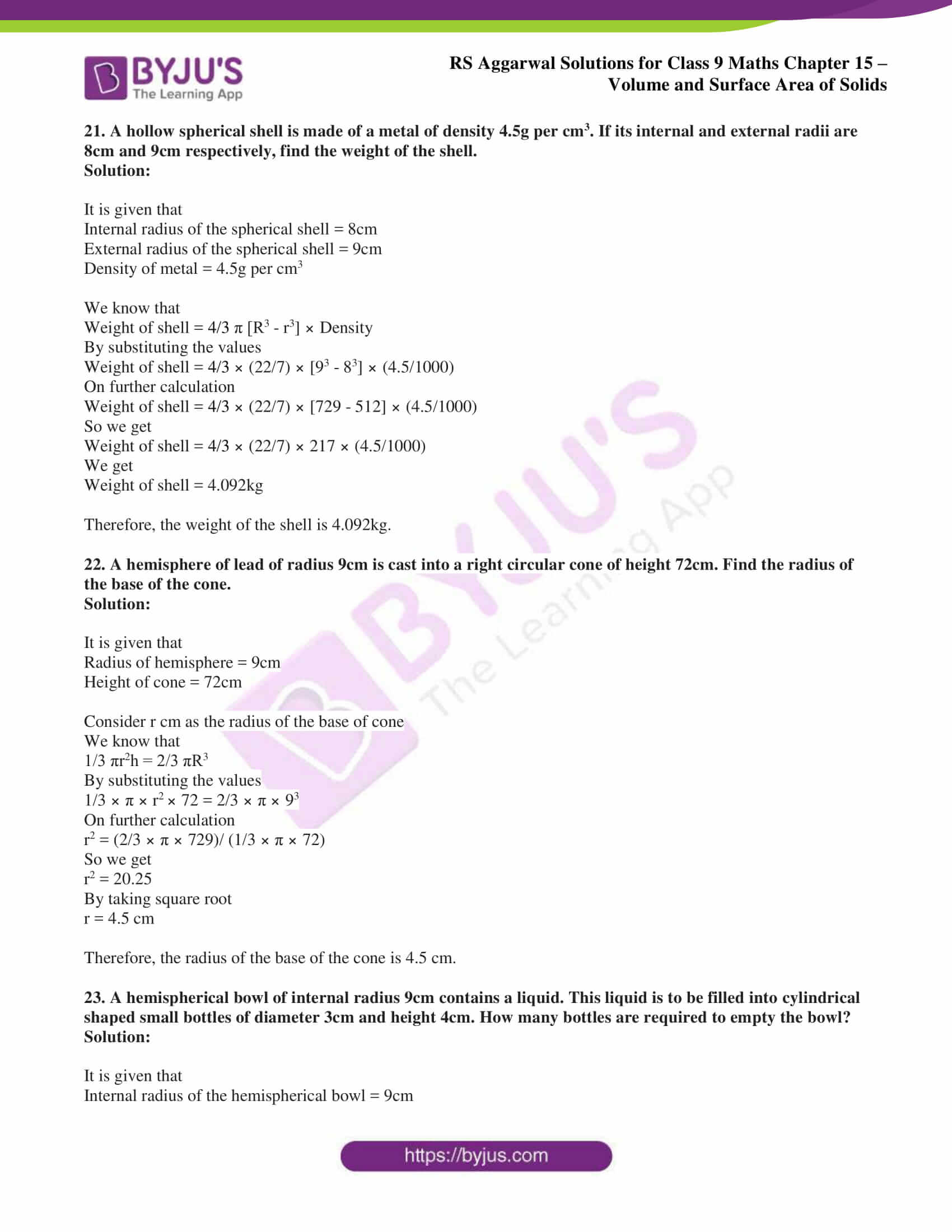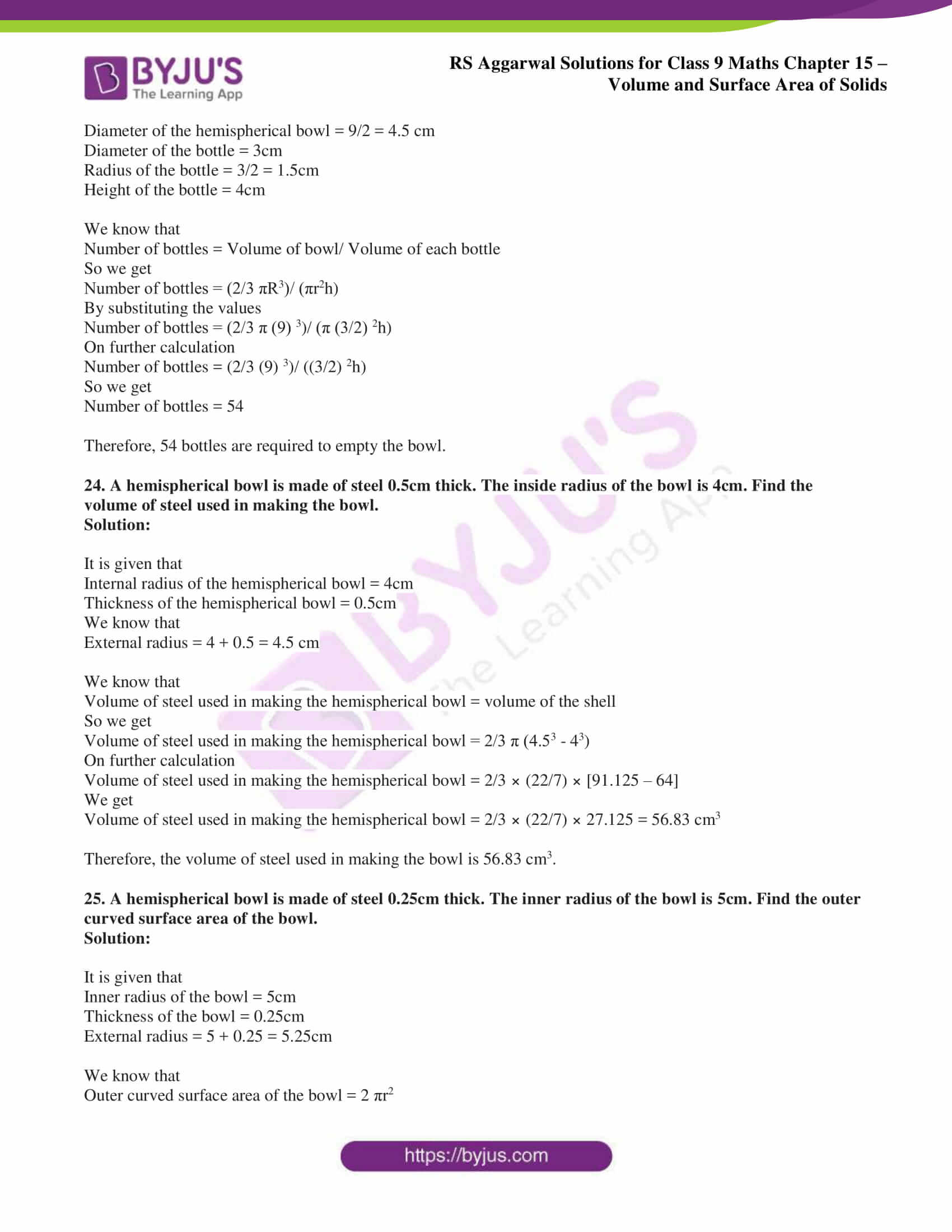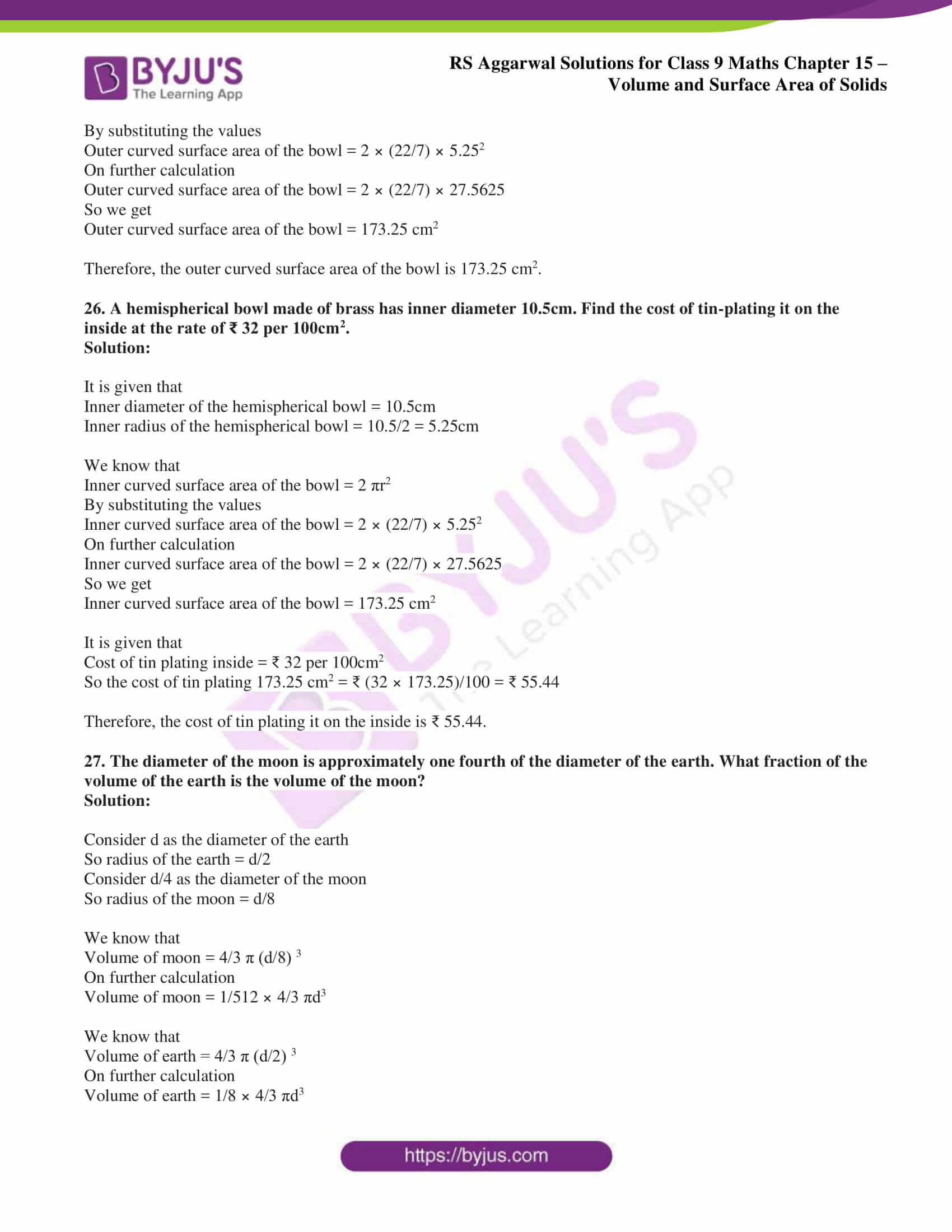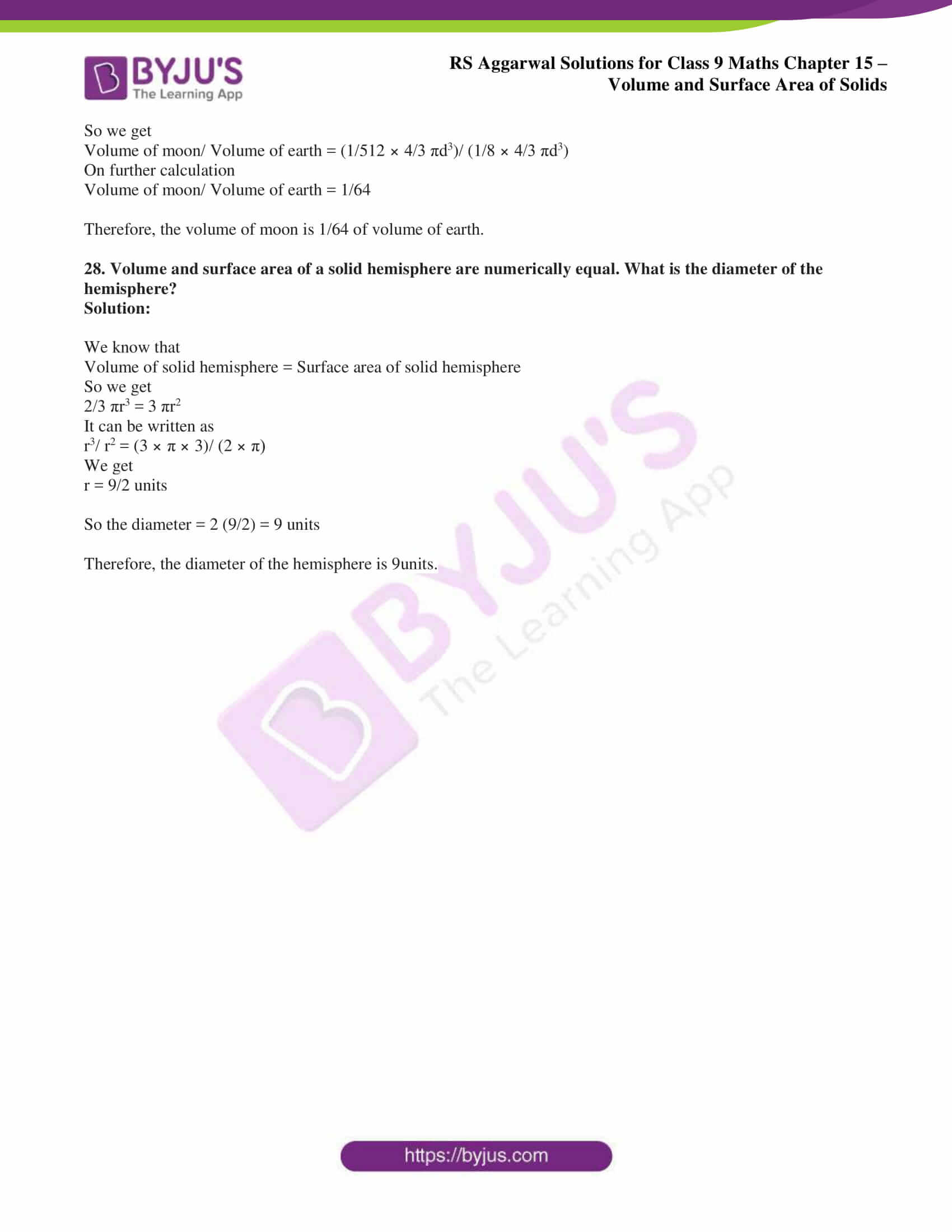## Exercise 15(A)

1. Find the volume, the lateral surface area and the total surface area of the cuboid whose dimensions are:

(i) length = 12cm, breadth = 8cm and height = 4.5cm

(ii) length = 26m, breadth = 14m and height = 6.5m

(iii) length = 15m, breadth = 6m and height = 5dm

(iv) length = 24m, breadth = 25cm and height = 6m.

Solution:

(i) It is given that length = 12cm, breadth = 8cm and height = 4.5cm

We know that

Volume of cuboid = l × b × h

By substituting the values we get

Volume of cuboid = 12 × 8 × 4.5

By multiplication

Volume of cuboid = 432 cm3

We know that

Lateral surface area of a cuboid = 2 (l + b) × h

By substituting the values

Lateral surface area of a cuboid = 2 (12 + 8) × 4.5

On further calculation

Lateral surface area of a cuboid = 2 × 20 × 4.5

By multiplication

Lateral surface area of a cuboid = 180cm2

We know that

Total surface area of cuboid = 2 (lb + bh + lh)

By substituting the values

Total surface area of cuboid = 2 (12 × 8 + 8 × 4.5 + 12 × 4.5)

On further calculation

Total surface area of cuboid = 2 (96 + 36 + 54)

So we get

Total surface area of cuboid = 2 × 186

By multiplication

Total surface area of cuboid = 372 cm2

(ii) It is given that length = 26m, breadth = 14m and height = 6.5m

We know that

Volume of cuboid = l × b × h

By substituting the values we get

Volume of cuboid = 26 × 14 × 6.5

By multiplication

Volume of cuboid = 2366 m3

We know that

Lateral surface area of a cuboid = 2 (l + b) × h

By substituting the values

Lateral surface area of a cuboid = 2 (26 + 14) × 6.5

On further calculation

Lateral surface area of a cuboid = 2 × 40 × 6.5

By multiplication

Lateral surface area of a cuboid = 520cm2

We know that

Total surface area of cuboid = 2 (lb + bh + lh)

By substituting the values

Total surface area of cuboid = 2 (26 × 14 + 14 × 6.5 + 26 × 6.5)

On further calculation

Total surface area of cuboid = 2 (364 + 91 + 169)

So we get

Total surface area of cuboid = 2 × 624

By multiplication

Total surface area of cuboid = 1248 m2

(iii) It is given that length = 15m, breadth = 6m and height = 5dm = 0.5m

We know that

Volume of cuboid = l × b × h

By substituting the values we get

Volume of cuboid = 15 × 6 × 0.5

By multiplication

Volume of cuboid = 45 m3

We know that

Lateral surface area of a cuboid = 2 (l + b) × h

By substituting the values

Lateral surface area of a cuboid = 2 (15 + 6) × 0.5

On further calculation

Lateral surface area of a cuboid = 2 × 21 × 0.5

By multiplication

Lateral surface area of a cuboid = 21 m2

We know that

Total surface area of cuboid = 2 (lb + bh + lh)

By substituting the values

Total surface area of cuboid = 2 (15 × 6 + 6 × 0.5 + 15 × 0.5)

On further calculation

Total surface area of cuboid = 2 (90 + 3 + 7.5)

So we get

Total surface area of cuboid = 2 × 100.5

By multiplication

Total surface area of cuboid = 201 m2

(iv) It is given that length = 24m, breadth = 25cm = 0.25m and height = 6m

We know that

Volume of cuboid = l × b × h

By substituting the values we get

Volume of cuboid = 24 × 0.25 × 6

By multiplication

Volume of cuboid = 36 m3

We know that

Lateral surface area of a cuboid = 2 (l + b) × h

By substituting the values

Lateral surface area of a cuboid = 2 (24 + 0.25) × 6

On further calculation

Lateral surface area of a cuboid = 2 × 24.25 × 6

By multiplication

Lateral surface area of a cuboid = 291 m2

We know that

Total surface area of cuboid = 2 (lb + bh + lh)

By substituting the values

Total surface area of cuboid = 2 (24 × 0.25 + 0.25 × 6 + 24 × 6)

On further calculation

Total surface area of cuboid = 2 (6 + 1.5 + 144)

So we get

Total surface area of cuboid = 2 × 151.5

By multiplication

Total surface area of cuboid = 303 m2

2. A matchbox measure 4cm × 2.5cm × 1.5 cm. What is the volume of a packet containing 12 such matchboxes?

Solution:

It is given that

Length of the matchbox = 4cm

Breadth of the matchbox = 2.5cm

Height of the matchbox = 1.5cm

We know that

Volume of one matchbox = volume of cuboid = l × b × h

By substituting the values

Volume of one matchbox = 4 × 2.5 × 1.5

By multiplication

Volume of one matchbox = 15 cm3

So the volume of 12 such matchboxes = 12 × 15 = 180 cm3

Therefore, the volume of a packet containing 12 such matchboxes is 180 cm3.

3. A cuboidal water tank is 6m long, 5m wide and 4.5m deep. How many litres of water can it hold? (Given, 1m3 = 1000 litres.)

Solution:

It is given that

Length of the cuboidal water tank = 6m

Breadth of the cuboidal water tank = 5m

Height of the cuboidal water tank = 4.5m

We know that

Volume of a cuboidal water tank = l × b × h

By substituting the values

Volume of a cuboidal water tank = 6 × 5 × 4.5

By multiplication

Volume of a cuboidal water tank = 135 m3

We know that 1m3 = 1000 litres

So we get

Volume of a cuboidal water tank = 135 × 1000 = 135000 litres

Therefore, the cuboidal water tank can hold 135000 litres of water.

4. The capacity of a cuboidal tank is 50000 litres of water. Find the breadth of the tank if its length and depth are respectively 10m and 2.5m. (Given, 1000 litres = 1m3.)

Solution:

It is given that

Length of the cuboidal tank = 10m

Depth of the cuboidal tank = 2.5m

Volume of the cuboidal tank = 5000 litres = 50 m3

We know that

Volume of a cuboidal tank = l × b × h

By substituting the values

50 = 10 × b × 2.5

On further calculation

b = 2m

Therefore, the breadth of the cuboidal tank is 2m.

5. A godown measure 40m × 25m × 15m. Find the maximum number of wooden crates, each measuring 1.5m × 1.25m × 0.5m that can be stored in the godown.

Solution:

It is given that

Length of the godown = 40m

Breadth of the godown = 25m

Height of the godown = 15m

We know that

Volume of godown = l × b × h

By substituting the values

Volume of godown = 40 × 25 × 15

On further calculation

Volume of godown = 15000 m3

It is given that

Length of wooden crate = 1.5m

Breadth of wooden crate = 1.25m

Height of wooden crate = 0.5m

We know that

Volume of each wooden crate = l × b × h

By substituting the values

Volume of each wooden crate = 1.5 × 1.25 × 0.5

On further calculation

Volume of each wooden crate = 0.9375 m3

So we get

Number of wooden crates that can be stored in godown = Volume of godown/ Volume of each wooden crate

By substituting the values

Number of wooden crates that can be stored in godown = 15000/ 0.9375

So we get

Number of wooden crates that can be stored in godown = 16000

Therefore, the number of wooden crates that can be stored in the godown are 16000.

6. How many planks of dimensions (5m × 25cm × 10cm) can be stored in a pit which is 20m long, 6m wide and 80cm deep?

Solution:

The dimensions of the plank are

Length = 5m = 500cm

Height = 10cm

We know that

Volume of the plank = l × b × h

By substituting the values

Volume of the plank = 500 × 25 × 10

So we get

Volume of the plank = 125000 cm3

The dimensions of the pit are

Length = 20m = 2000 cm

Breadth = 6m = 600 cm

Height = 80cm

We know that

Volume of one pit = l × b × h

By substituting the values

Volume of one pit = 2000 × 600 × 80

So we get

Volume of one pit = 96000000cm3

So the number of planks that can be stored = Volume of one pit/ Volume of plank

By substituting the values

Number of planks that can be stored = 96000000/125000

So we get

Number of planks that can be stored = 768

Therefore, the number of planks that can be stored is 768.

7. How many bricks will be required to construct a wall 8m long, 6m high and 22.5cm thick if each brick measures (25cm × 11.25cm × 6cm)?

Solution:

The dimensions of the wall are

Length = 8m = 800 cm

Breadth = 6m = 600 cm

Height = 22.5 cm

We know that

Volume of wall = l × b × h

By substituting the values

Volume of wall = 800 × 600 × 22.5

By multiplication

Volume of wall = 10800000 cm3

The dimensions of brick are

Length = 25cm

Height = 6cm

We know that

Volume of brick = l × b × h

By substituting the values

Volume of brick = 25 × 11.25 × 6

By multiplication

Volume of brick = 1687.5 cm3

So the number of bricks required = Volume of wall/ Volume of brick

By substituting the values

Number of bricks required = 10800000/1687.5

By division

Number of bricks required = 6400

Therefore, the number of bricks required to construct a wall is 6400.

8. Find the capacity of a closed rectangular cistern whose length is 8m, breadth 6m and depth 2.5m. Also, find the area of the iron sheet required to make the cistern.

Solution:

The dimensions of the cistern are

Length = 8m

Height = 2.5m

We know that

Capacity of cistern = volume of cistern

Volume of cistern = l × b × h

By substituting the values

Volume of cistern = 8 × 6 × 2.5

By multiplication

Volume of cistern = 120m3

We know that the area of iron sheet required is equal to the total surface area of the cistern

So we get

Total surface area = 2 (lb + bh + lh)

By substituting the values

Total surface area = 2 (8 × 6 + 6 × 2.5 + 2.5 × 8)

On further calculation

Total surface area = 2 (48 + 15 + 20)

So we get

Total surface area = 2 × 83 = 166 m2

Therefore, the capacity of the cistern is 120 m3 and the area of the iron sheet required to make the cistern is 166m2.

9. The dimensions of a room are (9m × 8m × 6.5m). It has one door of dimensions (2m × 1.5m) and two windows, each of dimensions (1.5m × 1m). Find the cost of whitewashing the walls at ₹ 25 per square metre.

Solution:

The dimensions of the room is

Length = 9m

Height = 6.5m

We know that

Area of four walls of the room = 2 (l + b) × h

By substituting the values

Area of the four walls of the room = 2 (9 + 8) × 6.5

On further calculation

Area of the four walls of the room = 34 × 6.5

So we get

Area of the four walls of the room = 221 m2

The dimensions of the door are

Length = 2m

We know that

Area of one door = l × b

By substituting the values

Area of one door = 2 × 1.5

So we get

Area of one door = 3m2

The dimensions of the window are

Length = 1.5m

We know that

Area of two windows = 2 (l × b)

By substituting the values

Area of two windows = 2 (1.5 × 1)

On further calculation

Area of two windows = 2 × 1.5 = 3m2

So the area to be whitewashed = Area of four walls of the room – Area of one door – Area of two windows

By substituting the values

Area to be whitewashed = (221 – 3 – 3)

So we get

Area to be whitewashed = 215m2

It is given that the cost of whitewashing = ₹ 25 per square metre

So the cost of whitewashing 215m2 = ₹ (25 × 215)

Cost of whitewashing 215m2 = ₹ 5375

Therefore, the cost of whitewashing 215m2 is ₹ 5375.

10. A wall 15m long, 30cm wide and 4m high is made of bricks, each measuring (22cm × 12.5cm × 7.5cm). If 1/12 of the total volume of the wall consists of mortar, how many bricks are there in the wall?

Solution:

The dimensions of the wall are

Length = 15m

Height = 4m

We know that

Volume of the wall = l × b × h

By substituting the values

Volume of the wall = 15 × 0.3 × 4

So we get

Volume of the wall = 18 m3

It is given that the wall consists of 1/12 mortar

So we get

Volume of mortar = 1/12 × 18

By division

Volume of mortar = 1.5 m3

So the volume of wall = Volume of wall – Volume of mortar

By substituting the values

Volume of wall = 18 – 1.5

By subtraction

Volume of wall = 16.5 m3

The dimensions of the brick are

Length = 22cm = 0.22 m

Breadth = 12.5 cm = 0.125 m

Height = 7.5cm = 0.075 m

We know that

Volume of one brick = l × b × h

By substituting the values

Volume of one brick = 0.22 × 0.125 × 0.075

So we get

Volume of one brick = 0.0020625 m3

So the number of bricks = Volume of bricks/ Volume of one brick

By substituting the values

Number of bricks = 16.5/0.0020625

So we get

Number of bricks = 8000

Therefore, the number of bricks in the wall are 8000.

11. How many cubic centimetres of iron are there in an open box whose external dimensions are 36cm, 25cm and 16.5cm, the iron being 1.5cm thick throughout? If 1cm3 of iron weighs 15g, find the weight of the empty box in kilograms.

Solution:

The external dimensions of the box are

Length = 36cm

Height = 16.5cm

We know that

External volume of the box = l × b × h

By substituting the values

External volume of the box = 36 × 25 × 16.5

So we get

External volume of the box = 14850 cm3

It is given that the box is 1.5cm thick throughout

Internal length of the box = (36 – (1.5 × 2))

So we get

Internal length of the box = 33 cm

Internal breadth of the box = (25 – (1.5 × 2))

So we get

Internal breadth of the box = 22cm

Internal height of the box = (16.5 – 1.5)

So we get

Internal height of the box = 15cm

We know that

Internal Volume of the box = l × b × h

By substituting the values

Internal Volume of the box = 33 × 22 × 15

By multiplication

Internal volume of the box = 10890 cm3

So the volume of iron used in the box = External volume of box – internal volume of box

By substituting the values

Volume of iron used in the box = 14850 – 10890 = 3960 cm3

It is given that

Weight of 1cm3 of iron = 15g = 15/1000 kg

So the weight of 3960 cm3 of iron = 3960 × (15/1000)

We get

Weight of 3960 cm3 of iron = 59.4 kg

Therefore, the volume of iron used in the box is 3960 cm3 and the weight of the empty box is 59.4 kg.

12. A box made of sheet metal costs ₹ 6480 at ₹ 120 per square metre. If the box is 5m long and 3m wide, find its height.

Solution:

We know that

Area of sheet metal = Total cost/ Cost per m2

By substituting the values

Area of sheet metal = 6480/120

So we get

Area of sheet metal = 54 m2

So we get

Area of sheet metal = 2 (lb + bh + hl)

By substituting the values

54 = 2 (5 × 3 + 3 × h + h × 5)

On further calculation

27 = 15 + 3h + 5h

So we get

8h = 12

By division

h = 1.5m

Therefore, the height of sheet metal is 1.5m.

13. The volume of a cuboid is 1536m3. Its length is 16m, and its breadth and height are in the ratio 3:2. Find the breadth and height of the cuboid.

Solution:

It is given that

Volume of cuboid = 1536 m3

Length of cuboid = 16m

Consider breath as 3x and height as 2x

We know that

Volume of cuboid = l × b × h

By substituting the values

1536 = 16 × 3x × 2x

On further calculation

1536 = 96 x2

So we get

x2 = 1536/96

x2 = 16

By taking the square root

x = √16

We get

x = 4m

Substituting the value of x

Breadth of cuboid = 3x = 3(4) = 12m

Height of cuboid = 2x = 2(4) = 8m

Therefore, the breadth and height of the cuboid are 12m and 8m.

14. How many persons can be accommodated in a dining hall of dimensions (20m × 16m × 4.5m), assuming that each person requires 5 cubic metres of air?

Solution:

The dimensions of hall are

Length = 20m

Height = 4.5m

We know that

Volume of hall = l × b × h

By substituting the values

Volume of hall = 20 × 16 × 4.5

So we get

Volume of hall = 1440 m3

It is given that volume of air for each person = 5 cubic metres

So the number of persons = volume of hall/ volume of air needed per person

By substituting the values

Number of persons = 1440/5

So we get

Number of persons = 288

15. A classroom is 10m long, 6.4m wide and 5m high. If each student be given 1.6m2 of the floor area, how many students can be accommodated in the room? How many cubic metres of air would each student get?

Solution:

The dimensions of classroom are

Length = 10m

Height = 5m

It is given that the floor area for each student = 1.6m2

So the number of students = area of room/floor area for each student

By substituting the values

Number of students = (10 × 6.4)/1.6

So we get

Number of students = 40

So the air required by each student = Volume of room/ number of students

We know that Volume of room = l × b × h

By substituting the values

Volume of room = 10 × 6.4 × 5 = 320 m3

So we get

Air required by each student = 320/40 = 8m3

Therefore, the number of students that can be accommodated in the room is 40 and the air required by each student is 8m3.

16. The surface area of a cuboid is 758cm2. Its length and breadth are 14cm and 11cm respectively. Find its height.

Solution:

It is given that

Surface area of cuboid = 758 cm2

The dimensions of cuboid are

Length = 14cm

Consider h as the height of cuboid

We know that

Surface area of cuboid = 2 (lb + bh + lh)

By substituting the values

758 = 2 (14 × 11 + 11 × h + 14 × h)

On further calculation

758 = 2 (154 + 11h + 14h)

So we get

758 = 2 (154 + 25h)

By multiplication

758 = 308 + 50h

It can be written as

50h = 758 – 308

By subtraction

50h = 450

By division

h = 9cm

Therefore, the height of cuboid is 9cm.

17. In a shower, 5cm of rain falls. Find the volume of water that falls on 2 hectares of ground.

Solution:

We know that 1 hectare = 10000 m2

So we get

2 hectares = 2 × 10000 = 20000 m2

It is given that

Depth of ground = 5cm = 0.05m

We know that

Volume of water = area × depth

By substituting the values

Volume of water = 20000 × 0.05 = 1000 m3

Therefore, the volume of water that falls is 1000 m3.

18. Find the volume, the lateral surface area, the total surface area and the diagonal of a cube, each of whose edges measures 9m. (Take √3 = 1.73)

Solution:

It is given that each edge of a cube = 9m

We know that

Volume of cube = a3

By substituting the values

Volume of cube = 93

So we get

Volume of cube = 729 m3

We know that

Lateral surface area of cube = 4a2

By substituting the values

Lateral surface area of cube = 4 × 92

So we get

Lateral surface area of cube = 4 × 81 = 324 m2

We know that

Total surface area of cube = 6a2

By substituting the values

Total surface area of cube = 6 × 92

So we get

Total surface area of cube = 6 × 81 = 486 m2

We know that

Diagonal of cube = √3 a

By substituting the values

Diagonal of cube = 1.73 × 9 = 15.57 m

Therefore, the volume is 729 m3, lateral surface area is 324 m2, total surface area is 486 m2 and the diagonal of cube is 15.57 m.

19. The total surface area of a cube is 1176 cm2. Find its volume.

Solution:

Consider a cm as each side of the cube

We know that

Total surface area of the cube = 6a2

By substituting the values

6a2 = 1176

On further calculation

a2 = 1176/6

So we get

a2 = 196

By taking square root

a = √ 196 = 14cm

We know that

Volume of cube = a3

By substituting the values

Volume of cube = 143

So we get

Volume of cube = 2744 cm3

Therefore, the volume of cube is 2744 cm3.

20. The lateral surface area of a cube is 900 cm2. Find its volume.

Solution:

Consider a cm as each side of the cube

We know that

Lateral surface area of cube = 4a2

By substituting the values

4a2 = 900

On further calculation

a2 = 900/4

By division

a2 = 225

By taking square root

a = √ 225 = 15cm

We know that

Volume of cube = a3

By substituting the values

Volume of cube = 153

So we get

Volume of cube = 3375 cm3

Therefore, the volume of cube is 3375 cm3.

21. The volume of a cube is 512cm3. Find its surface area.

Solution:

It is given that

Volume of a cube = 512 cm3

We know that

Volume of cube = a3

So we get

Each edge of the cube = ∛ 512 = 8cm

We know that

Surface area of cube = 6a2

By substituting the values

Surface area of cube = 6 × (8)2

So we get

Surface area of cube = 6 × 64 = 384 cm2

Therefore, the surface area of cube is 384 cm2.

22. Three cubes of metal with edges 3cm, 4cm and 5cm respectively are melted to form a single cube. Find the lateral surface area of the new cube formed.

Solution:

We know that

Volume of new cube = (33 + 43 + 53)

So we get

Volume of new cube = 27 + 64 + 125 = 216 cm2

Consider a cm as the edge of the cube

So we get

a3 = 216

By taking cube root

a = ∛ 216 = 6cm

We know that

Lateral surface area of the new cube = 4a2

By substituting the values

Lateral surface area of the new cube = 4 × (6)2

So we get

Lateral surface area of the new cube = 4 × 36 = 144 cm2

Therefore, the lateral surface area of the new cube formed is 144 cm2.

23. Find the length of the longest pole that can be put in a room of dimensions (10m × 10m × 5m).

Solution:

The dimensions of the room are

Length = 10m

Height = 5m

We know that

Length of the longest pole = length of diagonal = √ (l2 + b2 + h2)

By substituting the values

Length of the longest pole = √ (102 + 102 + 52)

So we get

Length of the longest pole = √ (100 + 100 + 25)

Length of the longest pole = √ 225 = 15m

Therefore, the length of the longest pole that can be put in the room is 15m.

24. The sum of length, breadth and depth of a cuboid is 19cm and the length of its diagonal is 11cm. Find the surface area of the cuboid.

Solution:

It is given that

l + b + h = 19cm ……. (1)

Diagonal = √ (l2 + b2 + h2) = 11cm…….. (2)

Squaring on both sides of equation (1)

(l + b + h) 2 = 192

So we get

(l2 + b2 + h2) + 2 (lb + bh + hl) = 361 …….. (3)

Squaring on both sides of equation (2)

l2 + b2 + h2 = 112 = 121 ……. (4)

Substituting equation (4) in (3)

121 + 2 (lb + bh + hl) = 361

So we get

2 (lb + bh + hl) = 361 – 121

By subtraction

2 (lb + bh + hl) = 240 cm2

Therefore, the surface area of the cuboid = 240 cm2.

25. Each edge of a cube is increased by 50%. Find the percentage increase in the surface area of the cube.

Solution:

Consider a cm as the edge of the cube

We know that

Surface area of cube = 6 a2

So we get

New edge = a + 50% of a

It can be written as

New edge = a + 50/100 a

By LCM

New edge = 150/100 a

We get

New edge = 3/2 a cm

So the new surface area = 6 (3/2 a) 2

We get

New surface area = 6 × 9/4 a2 = 27/2 a2 cm2

Increased surface area = new surface area – surface area

So we get

Increased surface area = 27/2 a2 – 6a2 = 15/2 a2 cm2

So the percentage increase in surface area = (increased surface area/original surface area) × 100

By substituting the values

Percentage increase in surface area = (15/2 a2 / 6a2) × 100

It can be written as

Percentage increase in surface area = 15/2 a2 × 1/6a2 × 100

So we get

Percentage increase in surface area = 125%

Therefore, the percentage increase in the surface area of the cube is 125%.

26. If V is the volume of a cuboid of dimensions a, b, c and S is its surface area then prove that 1/V = 2/S (1/a + 1/b + 1/c).

Solution:

We know that

Volume of a cuboid = a × b × c

Surface area of cuboid = 2 (ab + bc + ac)

So we get

2/s (1/a + 1/b + 1/c) = 2/s ((bc + ac + ab)/abc)

It can be written as

2/s (1/a + 1/b + 1/c) = 2/s (s/2V)

On further calculation

2/s (1/a + 1/b + 1/c) = 1/V

We get

1/V = 2/S (1/a + 1/b + 1/c)

Therefore, it is proved that 1/V = 2/S (1/a + 1/b + 1/c).

27. Water in a canal, 30dm wide and 12dm deep, is flowing with a velocity of 20km per hour. How much area will it irrigate, if 9cm of standing water is desired?

Solution:

We know that water in a canal forms a cuboid

The dimensions are

Height = 12dm = 1.2m

We know that

Length = distance covered by water in 3 minutes = velocity of water in m/hr × time in hours

By substituting the values

Length = 20000 × (30/60)

So we get

Length = 10000m

We know that

Volume of water flown in 30 minutes = l × b × h

By substituting the values

Volume of water flown in 30 minutes = 10000 × 3 × 1.2 = 36000 m3

Consider A m2 as the area irrigated

So we get

A × (9/100) = 36000

On further calculation

A = 400000 m2

Therefore, the area to be irrigated is 400000 m2.

28. A solid metallic cuboid of dimensions (9m × 8m × 2m) is melted and recast into solid cubes of edge 2m. Find the number of cubes so formed.

Solution:

The dimensions of cuboid are

Length = 9m

Height = 2m

We know that

Volume of cuboid = l × b × h

By substituting the values

Volume of cuboid = 9 × 8 × 2

So we get

Volume of cuboid = 144 m3

We know that

Volume of each cube of edge 2m = a3

So we get

Volume of each cube of edge 2m = 23 = 8 m3

So the number of cubes formed = volume of cuboid / volume of each cube

By substituting the values

Number of cubes formed = 144/8 = 18

Therefore, the number of cubes formed is 18.

## Exercise 15(B)

1. The diameter of a cylinder is 28cm and its height is 40cm. Find the curved surface area, total surface area and the volume of the cylinder.

Solution:

It is given that

Diameter of a cylinder = 28cm

We know that radius = diameter/2 = 28/2 = 14cm

Height of a cylinder = 40cm

We know that

Curved surface area = 2 πrh

By substituting the values

Curved surface area = 2 × (22/7) × 14 × 40

So we get

Curved surface area = 3520 cm2

We know that

Total surface area = 2 πrh + 2 πr2

By substituting the values

Total surface area = (2 × (22/7) × 14 × 40) + (2 × (22/7) × 142)

On further calculation

Total surface area = 3520 + 1232 = 4752 cm2

We know that

Volume of cylinder = πr2h

By substituting the values

Volume of cylinder = (22/7) × 142 × 40

So we get

Volume of cylinder = 24640cm3

Therefore, the curved surface area, total surface area and the volume of cylinder are 3520 cm2, 4752 cm2 and 24640cm3.

2. A patient in a hospital is given soup daily in a cylindrical bowl of diameter 7cm. If the bowl is filled with soup to a height of 4cm, how much soup the hospital has to prepare daily to serve 250 patients?

Solution:

It is given that

Diameter of the bowl = 7cm

We know that

Radius of the bowl = 7/2 = 3.5cm

Height = 4cm

We know that

Volume of soup in one bowl = πr2h

By substituting the values

Volume of soup in one bowl = (22/7) × (3.5)2 × 4

So we get

Volume of soup in one bowl = 154cm3

So the volume of soup in 250 bowls = 250 × 154

On further calculation

Volume of soup in 250 bowls = 38500 cm3 = 38.5 litres

Therefore, the hospital must prepare 38.5 litres of soup daily to serve 250 patients.

3. The pillars of a temple are cylindrically shaped. Each pillar has a circular base of radius 20cm and height 10m. How much concrete mixture would be required to build 14 such pillars?

Solution:

It is given that

Radius of pillar = 20cm = 0.2m

Height of pillar = 10m

We know that

Volume of one pillar = πr2h

By substituting the values

Volume of one pillar = (22/7) × (0.2)2 × 10

So we get

Volume of one pillar = 1.2571 m3

So the volume of concrete mixture in 14 pillars = 14 × 1.2571 = 17.6m3

Therefore, the volume of concrete mixture required in 14 pillars is 17.6m3.

4. A soft drink is available in two packs:

(i) a tin can with a rectangular base of length 5cm, breadth 4cm and height 15cm, and

(ii) a plastic cylinder with circular base of diameter 7cm and height 10cm.

Which container has greater capacity and by how much?

Solution:

(i) The dimensions for a tin can with a rectangular base is

Length = 5cm

Height = 15cm

We know that

Volume of tin can = l × b × h

By substituting the values

Volume of tin can = 5 × 4 × 15

So we get

Volume of tin can = 300 cm3

(ii) The dimensions for cylinder with circular base

Diameter = 7cm

We know that radius = 7/2 = 3.5 cm

Height = 10cm

We know that

Volume of cylinder = πr2h

By substituting the values

Volume of cylinder = (22/7) × (3.5)2 × 10

So we get

Volume of cylinder = 385 cm3

We know that the volume of plastic cylinder is greater than volume of tin can

So difference in volume = 385 – 300 = 85 cm3

Therefore, a plastic cylinder has greater capacity than a tin can by 85 cm3.

5. There are 20 cylindrical pillars in a building, each having a diameter of 50cm and height 4m. Find the cost of cleaning them at ₹ 14 per m2.

Solution:

It is given that

Diameter of one pillar = 50cm = 0.5m

So the radius of one pillar = 0.5/2 = 0.25m

Height of one pillar = 4m

We know that

Lateral surface area of one pillar = 2 πrh

By substituting the values

Lateral surface area of one pillar = 2 × (22/7) × 0.25 × 4

So we get

Lateral surface area of one pillar = 6.285 m2

So the lateral surface area of 20 pillars = 20 × 6.285 = 125.714 m2

It is given that the cost of cleaning = ₹ 14 per m2

So the cost of cleaning 125.714 m2 = ₹ (14 × 125.714)

We get

Cost of cleaning 125.714 m2 = ₹ 1760

Therefore, the cost of cleaning 125.714 m2 is ₹ 1760.

6. The curved surface area of a right circular cylinder is 4.4 m2. If the radius of its base is 0.7m, find its

(i) height and

(ii) volume.

Solution:

It is given that

Curved surface area of a cylinder = 4.4 m2

Radius of the cylinder = 0.7m

(i) We know that

Curved surface area of a cylinder = 2 πrh

By substituting the values

4.4 = 2 × (22/7) × 0.7 × h

On further calculation

4.4 = 2 × 22 × 0.1 × h

So we get

h = 4.4/ (2 × 22 × 0.1)

By division

h = 1m

(ii) We know that

Volume of a cylinder = πr2h

By substituting the values

Volume of a cylinder = (22/7) × (0.7)2 × 1

So we get

Volume of a cylinder = 1.54 m3

7. The lateral surface area of a cylinder is 94.2 cm2 and its height is 5cm. Find

(i) the radius of its base and

(ii) its volume. (Take π = 3.14)

Solution:

It is given that

Lateral surface area of a cylinder = 94.2 cm2

Height = 5cm

(i) We know that

Lateral surface area of cylinder = 2 πrh

By substituting the values

94.2 = 2 × 3.14 × r × 5

On further calculation

r = 94.2/ (2 × 3.14 × 5)

So we get

r = 3cm

(ii) We know that

Volume of a cylinder = πr2h

By substituting the values

Volume of a cylinder = (3.14) × (3)2 × 5

So we get

Volume of a cylinder = 141.3 cm3

8. The capacity of a closed cylindrical vessel of height 1m is 15.4 litres. Find the area of the metal sheet needed to make it.

Solution:

It is given that

Volume of the cylindrical vessel = 15.4 litres = 15400 cm3

Height of the cylindrical vessel = 1m = 100cm

We know that

Volume of a cylinder = πr2h

By substituting the values

15400 = (22/7) × r2 × 100

On further calculation

r2 = (15400 × 7)/ (22 × 100)

So we get

r2 = 49

By taking square root

r = √ 49 = 7cm

So area of metal sheet needed = total surface area of cylinder

It can be written as

Area of metal sheet needed = 2 πr (h + r)

By substituting the values

Area of metal sheet needed = 2 × (22/7) × 7 (100 + 7)

On further calculation

Area of metal sheet needed = 2 × 22 × 107

So we get

Area of metal sheet needed = 4708 cm2

Therefore, the area of metal sheet needed is 4708 cm2.

9. The inner diameter of a cylindrical wooden pipe is 24cm and its outer diameter is 28cm. The length of the pipe is 35cm. Find the mass of the pipe, if 1cm3 of wood has a mass of 0.6g.

Solution:

The dimensions of a cylinder are

Internal diameter = 24cm

Internal radius = 24/12 = 12cm

External diameter = 28cm

External radius = 28/2 = 14cm

Length = 35cm

We know that

Volume of pipe = Volume of cylinder = π (R2 – r2) h

By substituting the values

Volume of pipe = ((22/7) × (142 – 122) × 35)

On further calculation

Volume of pipe = 22 × (196 – 144) × 5

So we get

Volume of pipe = 22 × 52 × 5

By multiplication

Volume of pipe = 5720 cm3

It is given that 1cm3 of wood has a mass of 0.6g

So the mass of pipe = 5720 × 0.6 = 3432g = 3.432kg

Therefore, the mass of pipe is 3.432kg.

10. In a water heating system, there is a cylindrical pipe of length 28m and diameter 5cm. Find the total radiating surface in the system.

Solution:

The dimensions of cylindrical pipe

Diameter = 5cm

Height = 28m = 2800cm

We know that

Total radiating surface in the system = curved surface area of cylindrical pipe = 2 πrh

By substituting the values

Total radiating surface in the system = 2 × (22/7) × 2.5 × 2800

So we get

Total radiating surface in the system = 44000 cm2

Therefore, the total radiating surface in the system is 44000 cm2.

11. Find the weight of a solid cylinder of radius 10.5cm and height 60cm if the material of the cylinder weighs 5g per cm3.

Solution:

It is given that

Height of cylinder = 60cm

We know that

Volume of cylinder = πr2h

By substituting the values

Volume of cylinder = (22/7) × (10.5)2 × 60

So we get

Volume of cylinder = 20790 cm3

So the weight of the cylinder if the material weighs 5 g per cm3 = 20790 × 5 = 103950g

We know that 1000g = 1kg

Weight of the cylinder = 103950/1000 = 103.95kg

Therefore, the weight of solid cylinder is 103.95kg.

12. The curved surface area of a cylinder is 1210 cm2 and its diameter is 20cm. Find its height and volume.

Solution:

It is given that

Curved surface area = 1210 cm2

Diameter of the cylinder = 20cm

Radius of the cylinder = 20/2 = 10cm

We know that

Curved surface area of the cylinder = 2 πrh

By substituting the values

1210 = 2 × (22/7) × 10 × h

So we get

h = (1210 × 7) / (2 × 22 × 10) = 19.25cm

We know that

Volume of cylinder = πr2h

By substituting the values

Volume of cylinder = (22/7) × (10)2 × 19.25

So we get

Volume of cylinder = 6050 cm3

Therefore, the height of cylinder is 19.25cm and the volume is 6050 cm3.

13. The curved surface area of a cylinder is 4400 cm2 and the circumference of its base is 110cm. Find the height and the volume of the cylinder.

Solution:

Consider r as the radius as h as the height of cylinder

It is given that

Surface area of cylinder = 2 πrh

By substituting the values

2 πrh = 4400 …… (1)

It is given that circumferences of its base = 2 πr

So we get

2 πr = 110

We know that

2 πrh/2 πr = 4400/ 110

On further calculation

h = 40cm

Substituting the value of h in (1)

We get

2 × (22/7) × r × 40 = 4400

On further calculation

r = (4400 × 7)/ (44 × 40)

So we get

r = 17.5cm

We know that

Volume of cylinder = πr2h

By substituting the values

Volume of cylinder = (22/7) × (17.5)2 × 40

So we get

Volume of cylinder = 38500 cm3

Therefore, the height of the cylinder is 40cm and the volume is 38500 cm3.

14. The radius of the base and the height of a cylinder are in the ratio 2: 3. If its volume is 1617cm3, find the total surface area of the cylinder.

Solution:

Consider radius as 2x cm and height as 3x cm

We know that

Volume of cylinder = πr2h

By substituting the values

Volume of cylinder = (22/7) × (2x) 2 × 3x

On further calculation

Volume of cylinder = (22/7) × 4x2 × 3x

So we get

Volume of cylinder = (22/7) × 12x3

It can be written as

1617 = (22/7) × 12x3

On further calculation

12x3 = (1617 × 7)/22

So we get

x3 = (1617 × 7)/(22 × 12)

x3 = 42.875

By taking cube root

x = ∛ 42.865

We get

x = 3.5

By substituting the value of x

Radius = 2x = 2(3.5) = 7cm

Height = 3x = 3(3.5) = 10.5cm

We know that

Total surface area = 2 πr (h + r)

By substituting the values

Total surface area = 2 × (22/7) × 7 (10.5 + 7)

On further calculation

Total surface area = 44 × 17.5

So we get

Total surface area = 770 cm2

Therefore, the total surface area of the cylinder is 770 cm2.

15. The total surface area of a cylinder is 462cm2. Its curved surface area is one third of its total surface area. Find the volume of the cylinder.

Solution:

We know that

Curved surface area = 1/3 × Total surface area

By substituting the values

Curved surface area = 1/3 × 462

So we get

Curved surface area = 154 cm2

So the total surface area – curved surface area = 462 – 154 = 308cm2

We know that

2 πr2 = 308

By substituting the values

2 × (22/7) × r2 = 308

On further calculation

r2 = (308 × 7)/44

So we get

r2 = 49

By taking square root

r = √49 = 7cm

We know that

Curved surface area = 2 πrh

By substituting the values

154 = 2 × (22/7) × 7 × h

So we get

h = 154/44

By division

h = 3.5 cm

So we get r = 7cm and h = 3.5 cm

We know that

Volume of the cylinder = πr2h

By substituting the values

Volume of cylinder = (22/7) × (7)2 × 3.5

So we get

Volume of cylinder = 539 cm3

Therefore, volume of the cylinder is 539 cm3.

16. The total surface area of a solid cylinder is 231 cm2 and its curved surface area is 2/3 of the total surface area. Find the volume of the cylinder.

Solution:

We know that

Curved surface area = 2/3 × Total surface area

By substituting the values

Curved surface area = 2/3 × 231

So we get

Curved surface area = 154 cm2

So the total surface area – curved surface area = 231 – 154 = 77cm2

We know that

2 πr2 = 77

By substituting the values

2 × (22/7) × r2 = 77

On further calculation

r2 = (77 × 7)/44

So we get

r2 = 49/4

By taking square root

r = √49/4 = 7/2cm

We know that

Curved surface area = 2 πrh

By substituting the values

154 = 2 × (22/7) × (7/2) × h

So we get

h = 154/22

By division

h = 7 cm

So we get r = 7/2cm and h = 7 cm

We know that

Volume of the cylinder = πr2h

By substituting the values

Volume of cylinder = (22/7) × (7/2)2 × 7

So we get

Volume of cylinder = 269.5 cm3

Therefore, volume of the cylinder is 269.5 cm3.

17. The ratio between the curved surface area and the total surface area of a right circular cylinder is 1: 2. Find the volume of the cylinder if its total surface area is 616 cm2.

Solution:

We know that

Curved surface area = 2 πrh

It is given that the ratio of curved surface area and total surface area is 1: 2

So we get

2 πrh/ 2 πr(h + r) = ½

On further calculation

h/ (h + r) = ½

It can be written as

2h = h + r

So we get

2h – h = r

h = r

By substituting h = r we get

2 πr (h + r) = 616

So we get

4 πr2 = 616

It can be written as

4 × (22/7) × r2 = 616

On further calculation

r2 = (616 × 7)/ 88

We get

r2 = 49

By taking out square root

r = √49 = 7cm

We know that

Volume = πr2h

By substituting the values

Volume of cylinder = (22/7) × (7)2 × 7

So we get

Volume of cylinder = 1078 cm3

Therefore, volume of the cylinder is 1078 cm3.

18. A cylindrical bucket, 28cm in diameter and 72cm high, is full of water. The water is emptied into a rectangular tank, 66cm long and 28cm wide. Find the height of the water level in the tank.

Solution:

It is given that

Diameter of the bucket = 28cm

Height of the bucket = 72cm

Length of the tank = 66cm

We know that

Volume of tank = volume of cylindrical bucket

l × b × h = πr2h

By substituting the values

66 × 28 × h = (22/7) × (14)2 × 72

On further calculation

h = (22 × 2 × 14 × 72)/ (66 × 28)

So we get

h = 24cm

Therefore, the height of the water level in the tank is 24cm.

19. The barrel of a fountain pen, cylindrical in shape, is 7cm long and 5mm in diameter. A full barrel of ink in the pen will be used up on writing 330 words on an average. How many words would use up a bottle of ink containing one fifth of a litre?

Solution:

The dimensions of barrel are

Length = 7cm

Diameter = 5mm

Radius = 5/2 = 2.5 mm = 0.25cm

We know that

Volume of barrel = πr2h

By substituting the values

Volume of cylinder = (22/7) × (0.25)2 × 7

So we get

Volume of cylinder = 1.375 cm3

So 1.375 cm3 is used for writing 330 words

So the bottle containing one fifth of a litre ink would write = 330 × (1/1.375) × (1/5) × 1000 = 48000 words

Therefore, a bottle of ink containing one fifth of a litre would write 48000 words.

20. 1cm3 of gold is drawn into a wire 0.1mm in diameter. Find the length of the wire.

Solution:

We know that

1cm3 = 1cm × 1cm × 1cm

1cm = 0.01m

So the volume of gold = 0.01m × 0.01m × 0.01m

We get

Volume of gold = 0.000001m3 ….. (1)

It is given that

Diameter of the wire drawn = 0.1mm

So the radius = 0.1/2 = 0.05mm = 0.00005 m ……. (2)

Consider length of the wire = h m ……. (3)

We know that

Volume of wire drawn = Volume of gold

By substituting the values using (1), (2) and (3)

πr2h = 0.000001

On further calculation

Π × 0.00005 × 0.00005 × h = 0.000001

So we get

h = (0.000001 × 7)/ (0.00005 × 0.00005 × 22) = 127.27m

Therefore, the length of the wire is 127.27m.

21. If 1cm3 of cast iron weighs 21g, find the weight of a cast iron pipe of length 1m with a bore of 3cm in which the thickness of the metal is 1cm.

Solution:

We know that

Internal radius = 3/2 = 1.5cm

External radius = 1.5 + 1 = 2.5cm

We know that

Volume of cast iron = (π × (2.5)2 × 100 – π × (1.5)2 × 100)

Taking the common terms out

Volume of cast iron = π × 100 × (2.52 – 1.52)

On further calculation

Volume of cast iron = (22/7) × 100 × (6.25 – 2.25)

So we get

Volume of cast iron = (22/7) × 100 × 4 = 1257.142 cm3

It is given that 1cm3 of cast iron weighs 21g

We know that 1kg = 1000g

So the weight of cast iron pipe = 1257.142 × (21/1000) = 26.4kg

Therefore, the weight of cast iron pipe is 26.4kg.

22. A cylindrical tube, open at both ends, is made of metal. The internal diameter of the tube is 10.4cm and its length is 25cm. The thickness of the metal is 8mm everywhere. Calculate the volume of the metal.

Solution:

It is given that

Internal diameter of the tube = 10.4cm

Internal radius of the tube = 10.4/ 2 = 5.2 cm

Length = 25cm

We know that

External radius = 5.2 + 0.8 = 6cm

We know that

Required volume = (π × 62 × 25 – π × 5.22 × 25)

Taking the common terms out

Required volume = π × 25 × (62 – 5.22)

On further calculation

Required volume = (22/7) × 25 × (36 – 27.04)

So we get

Required volume = (22/7) × 25 × 8.96 = 704 cm3

Therefore, the volume of the metal is 704 cm3.

23. It is required to make a closed cylindrical tank of height 1m and base diameter 140cm from a metal sheet. How many square metres of the sheet are required for the same?

Solution:

It is given that

Diameter of the cylinder = 140cm

Radius of the cylinder = 140/2 = 70cm

Height of the cylinder = 1m = 100cm

We know that

Area of sheet required = Total surface area of cylinder = 2 πr (h + r)

By substituting the values

Area of sheet required = 2 × (22/7) × 70 (100 + 70)

On further calculation

Area of sheet required = 2 × 22 × 10 × 170

So we get

Area of sheet required = 74800 cm2 = 7.48 m2

Therefore, the area of sheet required is 7.48 m2.

24. A juice seller has a large cylindrical vessel of base radius 15cm filled up to a height of 32cm with orange juice. The juice is filled in small cylindrical glasses of radius 3cm up to a height of 8cm, and sold for ₹ 15 each. How much money does he receive by selling the juice completely?

Solution:

The dimensions of the cylindrical vessel

Height = 32cm

We know that

Volume of cylindrical vessel = πr2h

By substituting the values

Volume of cylindrical vessel = (22/7) × (15)2 × 32

So we get

Volume of cylindrical vessel = 22628.571 cm3

The dimensions of cylindrical glass are

Height = 8cm

We know that

Volume of each small cylindrical glass = πr2h

By substituting the values

Volume of each small cylindrical glass = (22/7) × (3)2 × 8

So we get

Volume of each small cylindrical glass = 226.28 cm3

So the number of small glasses filled = volume of cylindrical vessel/ volume of each glass

By substituting the values

Number of small glasses filled = 22628.571/226.28 = 100

It is given that

Cost of 1 glass = ₹ 15

So the cost of 100 glasses = ₹ (15 × 100) = ₹ 1500

Therefore, the juice seller receives ₹ 1500 by selling 100 glasses of orange juice.

25. A well with inside diameter 10m is dug 8.4m deep. Earth taken out of it is spread all around it to a width of 7.5m to form an embankment. Find the height of the embankment.

Solution:

The dimensions of the well are

Depth = 8.4m

We know that

Volume of the earth dug out = Volume of well = πr2h

By substituting the values

Volume of the earth dug out = (22/7) × (5)2 × 8.4

So we get

Volume of the earth dug out = 660 m2

It is given that

Width of embankment = 7.5m

We know that

External radius of embankment R = 5 + 7.5 = 12.5m

Internal radius of embankment r = 5m

We know that

Area of embankment = π (R2 – r2)

By substituting the values

Area of embankment = (22/7) × (12.52 – 52)

On further calculation

Area of embankment = (22/7) × (156.25 – 25)

So we get

Area of embankment = (22/7) × 131.25 = 412.5 m2

We know that

Volume of embankment = volume of earth dug out = 660m2

So the height of embankment = volume of embankment/ area of embankment

By substituting the values

Height of embankment = 660/ 412.5 = 1.6m

Therefore, the height of the embankment is 1.6m.

26. How many litres of water flows out of a pipe having an area of cross section of 5cm2 in 1 minute, if the speed of water in the pipe is 30cm/sec?

Solution:

It is given that

Speed of water in the pipe = 30cm/sec

We know that

Volume of water that flows out of the pipe in one second = area of cross section × length of water flown in one second

By substituting the values

Volume of water that flows out of the pipe in one second = 5 (30) = 150cm3

So the volume of water that flows out of the pipe in one minute = 150 (60) = 9000 cm3 = 9 litres

Therefore, 9 litres of water flows out of the pipe in one minute.

27. A cylindrical water tank of diameter 1.4m and height 2.1m is being fed by a pipe of diameter 3.5cm through which water flows at the rate of 2m per second. In how much time will the tank be filled?

Solution:

Consider the tank to be filled in x minutes

We know that

Volume of water that flows through the pipe in x minutes = Volume of tank

By substituting the values

Π × (3.5/ (2 × 100)) 2 × (2 × 60x) = Π × (0.7)2 × 2.1

On further calculation

0.115395x = 3.23106

So we get

x = 28

Therefore, the tank will be filled in 28 minutes.

28. A cylindrical container with diameter of base 56cm contains sufficient water to submerge a rectangular solid of iron with dimensions (32cm × 22cm × 14cm). Find the rise in the level of water when the solid is completely submerged.

Solution:

Consider h cm as the rise in level of water

We know that

Volume of cylinder of height h and base radius 28cm = volume of rectangular iron solid

By substituting the values

(22/7) × 282 × h = 32 × 22 × 14

On further calculation

22 × 28 × 4 × h = 32 × 22 × 14

So we get

h = (32 × 22 × 14)/ (22 × 28 × 4)

By division

h = 4cm

Therefore, the rise in the level of water when the solid is completely submerged is 4cm.

29. Find the cost of sinking a tube-well 280m deep, having a diameter 3m at the rate of ₹ 15 per cubic metre. Find also the cost of cementing its inner curved surface at ₹ 10 per square metre.

Solution:

It is given that

Height = 280m

We know that

Volume of the tube well = πr2h

By substituting the values

Volume of the tube well = (22/7) × (1.5)2 × 280

So we get

Volume of the tube well = 1980 m3

It is given that

Cost of sinking the tube well = ₹ (15 × 1980) = ₹ 29700

We know that

Curved surface area of tube well = 2 πrh

By substituting the values

Curved surface area of tube well = 2 × (22/7) × 1.5 × 280

So we get

Curved surface area of tube well = 2640 m2

It is given that

So the cost of cementing = ₹ (10 × 2640) = ₹ 26400

Therefore, cost of sinking the tube well is ₹ 29700 and the cost of cementing is ₹ 26400.

30. Find the length of 13.2 kg of copper wire of diameter 4mm, when 1 cubic centimeter of copper weighs 8.4kg.

Solution:

Consider h m as the length of the wire

We know that

Volume of the wire × 8.4 g = (13.2 × 1000) g

By substituting the values

(22/7) × (2/10)2 × h × 8.4 = 13200

On further calculation

22 × (1/5)2 × h × 8.4 = 13200

So we get

h = (13200 × 5 × 5)/ (22 × 1.2)

By simplification

h = 12500cm = 125m

Therefore, the length of wire is 125m.

31. It costs ₹ 3300 to paint the inner curved surface of a cylindrical vessel 10m deep at the rate of ₹ 30 per m2. Find the

(i) inner curved surface area of the vessel,

(ii) inner radius of the base, and

(iii) capacity of the vessel.

Solution:

(i) We know that

Cost of painting inner curved surface of the vessel = cost of painting per m2 × inner curved surface of vessel

By substituting the values

3300 = 30 × Inner curved surface of vessel

On further calculation

Inner curved surface of vessel = 110 m2

(ii) Consider r as the inner radius of the base

It is given that depth = 10m

We know that

Inner curved surface of vessel = 2 πrh

By substituting the values

110 = 2 × (22/7) × r × 10

So we get

r = (110 × 7)/ (2 × 22 × 10)

r = 1.75m

(iii) We know that

Capacity of the vessel = πr2h

By substituting the values

Capacity of the vessel = (22/7) × (1.75)2 × 10

So we get

Capacity of the vessel = 96.25 m3

32. The difference between inside and outside surfaces of a cylindrical tube 14cm long, is 88cm2. If the volume of the tube is 176 cm3, find the inner and outer radii of the tube.

Solution:

Consider R cm as the outer radius and r cm as the inner radius of the cylindrical tube

It is given that length = 14 cm

We know that

Outside surface area – Inner surface area = 88

So we get

2 πRh – 2 πrh = 88

It can be written as

2 π(R-r) h = 88

By substituting the values

2 × (22/7) × (R – r) × 14 = 88

On further calculation

2 × 22 × (R – r) × 2 = 88

We get

R – r = 88/ (2 × 22 × 2) = 1 ……….. (1)

We know that

Volume of tube = 176 cm3

It can be written as

External volume – Internal volume = 176

So we get

πR2h – πr2h = 176

By taking common out

Π (R2– r2) h = 176

By substituting the values

(22/7) × (R – r)(R + r) × 14 = 176

Substituting equation (1)

22 × 1 × (R + r) × 2 = 176

We get

R + r = 176/ (22 × 2) = 4 ……… (2)

2R = 5

So we get R = 2.5 cm

By substituting r

2.5 – r = 1

So we get r = 1.5cm

Therefore, the inner and outer radii of the tube are 1.5cm and 2.5cm.

33. A rectangular sheet of paper 30cm × 18cm can be transformed into the curved surface of a right circular cylinder in two ways namely, either by rolling the paper along its length or by rolling it along its breadth. Find the ratio of the volumes of the two cylinders, thus formed.

Solution:

We know that

If the sheet is folded along its length it forms a cylinder of height h1 = 18cm and perimeter = 30cm

Consider r1 as the radius and V1 as the volume

So we get

2 πr1 = 30

It can be written as

r1 = 30/2 π = 15/π

We know that

V1 = πr12h1

By substituting the values

V1 = π × (15/π) 2 × 18

We get

V1 = (225/ π) × 18 cm3

We know that

If the sheet is folded along its breadth it forms a cylinder of height h2 = 30cm and perimeter 18cm

Consider r2 as the radius and V2 as the volume

So we get

2 πr2 = 18

It can be written as

r2 = 18/2 π = 9/π

We know that

V2 = πr22h2

By substituting the values

V2 = π × (9/π) 2 × 3

We get

V2 = (81/ π) × 30 cm3

So we get

V1/V2 = {(225/ π) × 18}/ {(81/ π) × 30)}

On further calculation

V1/V2 = (225 × 18)/ (81 × 30)

We get

V1/V2 = 5/3

We can write it as

V1:V2 = 5:3

Therefore, the ratio of the volumes of the two cylinders thus formed is 5:3.

## Exercise 15(c)

1. Find the curved surface area of a cone with base radius 5.25cm and slant height 10cm.

Solution:

It is given that

Radius of the cone = 5.25cm

Slant height of the cone = 10cm

We know that

Curved surface area of the cone = πrl

By substituting the values

Curved surface area of the cone = (22/7) × 5.25 × 10

So we get

Curved surface area of the cone = 165 cm2

Therefore, the curved surface area of a cone is 165 cm2.

2. Find the total surface area of a cone, if its slant height is 21m and diameter of its base is 24m.

Solution:

It is given that

Diameter of the cone = 24m

Radius of the cone = 24/2 = 12m

Slant height of the cone = 21m

We know that

Total surface area of a cone = πr (l + r)

By substituting the values

Total surface area of a cone = (22/7) × 12 (21+12)

On further calculation

Total surface area of a cone = (22/7) × 12 × 33

So we get

Total surface area of a cone = 1244.57 m2

Therefore, the total surface area of a cone is 1244.57 m2.

3. A joker’s cap is in the form of a right circular cone of base radius 7cm and height 24cm. Find the area of the sheet required to make 10 such caps.

Solution:

It is given that

Radius of the cap = 7cm

Height of the cap = 24cm

We know that

Slant height of the cap

l = √ (r2 + h2)

By substituting the values

l = √ (72 + 242)

On further calculation

l = √ (49 + 576) = √ 625

So we get

l = 25cm

We know that

Curved surface area of one cap = πrl

By substituting the values

Curved surface area of one cap = (22/7) × 7 × 25

So we get

Curved surface area of one cap = 550 cm2

So the curved surface area of 10 conical caps = 10 × 550 = 5500 cm2

Therefore, the area of the sheet required to make 10 such caps is 5500 cm2.

4. The curved surface area of a cone is 308cm2 and its slant height is 14cm. Find the radius of the base and total surface area of the cone.

Solution:

Consider r as the radius of the cone

It is given that

Slant height of the cone = 14cm

Curved surface area of the cone = 308cm2

It can be written as

Πrl = 308

By substituting the values

(22/7) × r × 14 = 308

On further calculation

22 × r × 2 = 308

So we get

r = 308/ (22 × 2)

r = 7cm

We know that

Total surface area of a cone = Πr (l + r)

By substituting the values

Total surface area of a cone = (22/7) × 7 × (14 + 7)

On further calculation

Total surface area of a cone = 22 ×21 = 462 cm2

Therefore, the base radius of the cone is 7cm and the total surface area is 462 cm2.

5. The slant height and base diameter of a conical tomb are 25m and 14m respectively. Find the cost of whitewashing its curved surface at the rate of ₹ 12 per m2.

Solution:

It is given that

Radius of the cone = 7m

Slant height of the cone = 25m

We know that

Curved surface area of the cone = πrl

By substituting the values

Curved surface area of the cone = (22/7) × 7 × 25

So we get

Curved surface area of the cone = 550 m2

It is given that the cost of whitewashing = ₹ 12 per m2

So the cost of whitewashing 550 m2 area = ₹ 12 × 550 = ₹ 6600

Therefore, the cost of whitewashing its curved surface area is ₹ 6600.

6. A conical tent is 10m high and the radius of its base is 24m. Find the slant height of the tent. If the cost of 1m2 canvas is ₹ 70, find the cost of canvas required to make the tent.

Solution:

It is given that

Radius of the conical tent = 24m

Height of conical tent = 10m

We know that

Slant height of conical tent can be written as

l = √ (r2 + h2)

By substituting the values

l = √ (242 + 102)

On further calculation

l = √ (576 + 100) = √ 676

So we get

l = 26m

We know that

Curved surface area of conical tent = πrl

By substituting the values

Curved surface area of conical tent = (22/7) × 24 × 26

So we get

Curved surface area of conical tent = (13728/7) m2

It is given that the cost of 1m2 canvas = ₹ 70

So the cost of (13728/7) m2 canvas = ₹ 70 × (13728/7) = ₹ 137280

Therefore, the slant height of the tent is 26m and the cost of canvas required to make the tent is ₹ 137280.

7. A bus stop is barricaded from the remaining part of the road by using 50 hollow cones made of recycled cardboard. Each one has a base diameter of 40cm and height 1m. If the outer side of each of the cones is to be painted and the cost of painting is ₹ 25 per m2, what will be the cost of painting all these cones? (Use π = 3.14 and √1.04 = 1.02.)

Solution:

It is given that

Radius of the cone = 20cm = 0.2m

Height of the cone = 1m

We know that

Slant height l = √ (r2 + h2)

By substituting the values

l = √ (0.22 + 12)

On further calculation

l = √ (0.04 + 1) = √ 1.04

So we get

l = 1.02m

We know that

Curved surface area of cone = πrl

By substituting the values

Curved surface area of cone = 3.14 × 0.2 × 1.02

On further calculation

Curved surface area of cone = 0.64056 m2

So the curved surface area of 50 cones = 50 × 0.64056 = 32.028 m2

It is given that

Cost of painting = ₹ 25 per m2

So the cost of painting 32.028 m2 area = ₹ 25 × 32.028 = ₹ 800.70

Therefore, the cost of painting all these cones is ₹ 800.70.

8. Find the volume, curved surface area and the total surface area of a cone having base radius 35cm and height 12cm.

Solution:

It is given that

Radius of the cone = 35cm

Height of the cone = 12cm

We know that

Volume of the cone = 1/3 πr2h

By substituting the values

Volume of the cone = 1/3 × (22/7) × 352 × 12

On further calculation

Volume of the cone = 15400 cm3

We know that

Slant height l = √ (r2 + h2)

By substituting the values

l = √ (352 + 122)

On further calculation

l = √ 1369

So we get

l = 37 cm

We know that

Curved surface area of a cone = πrl

By substituting the values

Curved surface area of a cone = (22/7) × 35 × 37

So we get

Curved surface area of a cone = 4070 cm2

We know that

Total surface area of cone = πr (l + r)

By substituting the values

Total surface area of cone = (22/7) × 35 × (37 + 35)

On further calculation

Total surface area of cone = 22 × 5 × 72

So we get

Total surface area of cone = 7920 cm2

9. Find the volume, curved surface area and the total surface area of a cone whose height is 6cm and slant height 100cm. (Take π = 3.14.)

Solution:

It is given that

Height of the cone = 6cm

Slant height of the cone b= 10cm

We know that

Radius of the cone = √ (l2 – h2)

By substituting the values

Radius of the cone = √ (102 – 62)

On further calculation

Radius of the cone = √ (100 – 36) = √ 64

So we get

Radius of the cone = 8cm

We know that

Volume of the cone = 1/3 πr2h

By substituting the values

Volume of the cone = 1/3 × 3.14 × 82 × 6

On further calculation

Volume of the cone = 401.92 cm3

We know that

Curved surface area of a cone = πrl

By substituting the values

Curved surface area of a cone = 3.14 × 8 × 10

So we get

Curved surface area of a cone = 251.2 cm2

We know that

Total surface area of cone = πr (l + r)

By substituting the values

Total surface area of cone = 3.14 × 8 × (10 + 8)

On further calculation

Total surface area of cone = 3.14 × 8 × 18

So we get

Total surface area of cone = 452.16 cm2

10. A conical pit of diameter 3.5m is 12m deep. What is its capacity in kilolitres?

Solution:

It is given that

Diameter of the conical pit = 3.5m

Radius of the conical pit = 3.5/2 = 1.75m

Depth of the conical pit = 12m

We know that

Volume of the conical pit = 1/3 πr2h

By substituting the values

Volume of the conical pit = 1/3 × (22/7) × 1.752 × 12

On further calculation

Volume of the conical pit = 38.5 m3 = 38.5 kilolitres

Therefore, the capacity of the conical pit is 38.5 kilolitres.

11. A heap of wheat is in the form of a cone of diameter 9m and height 3.5m. Find its volume. How much canvas cloth is required to just cover the heap? (Use π = 3.14.)

Solution:

It is given that

Diameter of the conical heap = 9m

Radius of the conical heap = 9/2 = 4.5m

Height of the conical heap = 3.5m

We know that

Volume of the conical heap = 1/3 πr2h

By substituting the values

Volume of the conical heap = 1/3 × 3.14 × 4.52 × 3.5

On further calculation

Volume of the conical heap = 3.14 × 1.5 × 4.5 × 3.5

So we get

Volume of the conical heap = 74.1825 m3

We know that

Slant height l =√ (r2 + h2)

By substituting the values

l = √ (4.52 + 3.52)

On further calculation

l = √ 32.5

So we get

l = 5.7 m

We know that

Curved surface area of the conical heap = πrl

By substituting the values

Curved surface area of the conical heap = 3.14 × 4.5 × 5.7

On further calculation

Curved surface area of the conical heap = 80.54 m2

Therefore, 80.54 m2 of canvas is required to cover the heap of wheat.

12. A man uses a piece of canvas having an area of 551m2, to make a conical tent of base radius 7m. Assuming that all the stitching margins and wastage incurred while cutting, amount to approximately 1m2, find the volume of the tent that can be made with it.

Solution:

It is given that

Radius of the conical tent = 7m

So the area of canvas required to make the conical tent = 551 – 1 = 550 m2

We know that

Curved surface area of a conical tent = 550

So we get

Πrl = 550

By substituting the values

(22/7) × 7 × l = 550

On further calculation

l = 550/22 = 25m

We know that

Height h = √ (l2 – r2)

By substituting the values

h = √ (252 – 72)

On further calculation

h = √ (625 – 49) = √ 576

So we get

h = 24m

We know that

Volume of the conical tent = 1/3 πr2h

By substituting the values

Volume of the conical tent = 1/3 × (22/7) × 72 × 24

On further calculation

Volume of the conical tent = 1232 m3

Therefore, the volume of the conical tent is 1232 m3.

13. How many metres of cloth, 2.5m wide, will be required to make a conical tent whose base radius is 7m and height 24m?

Solution:

It is given that

Radius of the conical tent = 7m

Height of the conical tent = 24m

We know that

Slant height l =√ (r2 + h2)

By substituting the values

l = √ (72 + 242)

On further calculation

l = √ (49 + 576) = √ 625

So we get

l = 25 m

We know that

Area of the cloth = πrl

By substituting the values

Area of the cloth = (22/7) × 7 × 25

On further calculation

Area of the cloth = 550 m2

We know that

Length of the cloth = area/ width

By substituting the values

Length of the cloth = 550/ 2.5 = 220m

Therefore, 220m of cloth is required to make the conical tent.

14. Two cones have their heights in the ratio 1:3 and the radii of their bases in the ratio 3:1. Show that their volumes are in the ratio 3:1.

Solution:

Consider the heights as h and 3h and radii as 3r and r

So we get

V1 = 1/3 π (3r) 2h and V2 = 1/3 πr2 × 3h

By dividing both we get

V1/ V2 = (1/3 π (3r) 2h)/ (1/3 πr2 × 3h)

On further calculation

V1/ V2 = 3/1

It can be written as

V1:V2 = 3:1

Therefore, it is proved that their volumes are in the ratio 3:1.

15. A cylinder and a cone have equal radii of their bases and equal heights. If their curved surface areas are in the ratio 8:5, show that the radius and height of each has the ratio 3:4.

Solution:

Consider the curved surface area of cylinder and cone as 8x and 5x.

So we get

2 πrh = 8x …….. (1)

Πr √ (h2 + r2) = 5x ……… (2)

By squaring equation (1)

(2 πrh) 2 = (8x) 2

So we get

4 π2r2h2 = 64 x2 …….. (3)

By squaring equation (2)

Π2r2 (h2 + r2) = 25x2 …… (4)

Dividing equation (3) by (4)

4 π2r2h2/ Π2r2 (h2 + r2) = 64 x2/25x2

On further calculation

h2/ (h2 + r2) = 16/25

It can be written as

9 h2 = 16 r2

So we get

r2/ h2 = 9/16

By taking square root

r/ h = ¾

We get

r: h = 3:4

Therefore, it is proved that the radius and height of each has the ratio 3:4.

16. A right circular cone is 3.6cm high and radius of its base is 1.6cm. It is melted and recast into a right circular cone having base radius 1.2cm. Find its height.

Solution:

It is given that

Height of the cone = 3.6cm

Radius of the cone = 1.6cm

We know that

Volume of original cone = Volume of cone after melting

By substituting the values

1/3 π × 1.62 × 3.6 = 1/3 π × 1.22 × h

It can be written as

h = (1/3 π × 1.62 × 3.6)/ (1/3 π × 1.22)

On further calculation

h = 6.4cm

Therefore, the height of the right circular cone is 6.4cm.

17. A circus tent is cylindrical to a height of 3 metres and conical above it. If its diameter is 15m and the slant height of the conical portion is 53m, calculate the length of the canvas 5m wide to make the required tent.

Solution:

It is given that

Diameter of the cylinder = 105m

Radius of the cylinder = 105/2 = 52.5m

Height of the cylinder = 3m

Slant height of the cylinder = 53m

We know that

Area of canvas = 2 πRH + πRl

By substituting the values

Area of canvas = (2 × (22/7) × 52.5 × 3) + ((22/7) × 52.5 × 53)

On further calculation

Area of canvas = 990 + 8745 = 9735m2

We know that

Length of canvas = area/ width = 9735/5 = 1947m

Therefore, the length of canvas required to make the tent is 1947m.

18. An iron pillar consists of a cylindrical portion 2.8m high and 20cm in diameter and a cone 42cm high is surmounting it. Find the weight of the pillar, given that 1cm3 of iron weighs 7.5g.

Solution:

It is given that

Height of the cylinder = 2.8m = 280cm

Diameter of the cylinder = 20cm

Radius of the cylinder = 20/2 = 10cm

Height of the cone = 42cm

We know that

Volume of the pillar = πr2h + 1/3 πr2H

It can be written as

Volume of the pillar = πr2 (h + 1/3H)

By substituting the values

Volume of the pillar = (22/7) × 102 (280 + (1/3 × 42))

On further calculation

Volume of the pillar = 2200/7 × (280 + 14) = 92400 cm3

So the weight of pillar = (92400 × 7.5)/ 1000 = 693kg

Therefore, the weight of the pillar is 693kg.

19. From a solid right circular cylinder with height 10cm and radius of the base 6cm, a right circular cone of the same height and base is removed. Find the volume of the remaining solid. (Take π = 3.14.)

Solution:

It is given that

Height of the cylinder = 10cm

Radius of the cylinder = 6cm

We know that

Volume of the remaining solid = πr2h – 1/3 πr2h

By substituting the values

Volume of the remaining solid = (π × 62 × 10) – (1/3 π × 62 × 10)

It can be written as

Volume of the remaining solid = 2/3 π × 62 × 10

So we get

Volume of the remaining solid = 2/3 × 3.14 × 360 = 753.6 cm3

Therefore, the volume of the remaining solid is 753.6 cm3.

20. Water flows at the rate of 10 metres per minute through a cylindrical pipe 5mm in diameter. How long would it take to fill a conical vessel whose diameter at the surface is 40cm and depth 24cm?

Solution:

It is given that

Diameter of the pipe = 5mm = 0.5cm

Radius of the pipe = 0.5/2 = 0.25cm

Length of the pipe = 10m = 1000cm

We know that

Volume of water that flows in 1 minute = π × 0.252 × 1000

So the volume of conical flask = 1/3 π × 202 × 24

The time required to fill the conical vessel = volume of the conical vessel/ volume that flows in 1 minute

By substituting the values

Required time = (1/3 π × 202 × 24)/ (π × 0.252 × 1000)

On further calculation

Required time = (1/3 π × 400 × 24)/ (π × 0.0625 × 1000)

So we get

Required time = 51.12 minutes = 51 minutes 12 seconds

Therefore, the time required to fill the conical vessel is 51 minutes 12 seconds.

21. A cloth having an area of 165 m2 is shaped into the form of a conical tent of radius 5m.

(i) How many students can sit in the tent if a student, on an average, occupies 5/7 m2 on the ground?

(ii) Find the volume of the cone.

Solution:

(i) We know that

Area of the floor of the tent = πr2

By substituting the values

Area of the floor of the tent = (22/7) × 52 = 550/7 m2

We know that the area required by one student is 5/7 m2

So the required number of students = (550/7)/ (5/7) = 110

(ii) We know that

Curved surface area of the tent = area of the cloth = 165 m2

So we get

Πrl = 165

By substituting the values

(22/7) × 5 × l = 165

On further calculation

l = (165 × 7)/ (22 × 5) = 21/2 m

We know that

h = √ (l2 – r2)

By substituting the values

h = √ ((21/2)2 – 52)

On further calculation

h = √ ((441/4) – 25) = √ (341/4)

So we get

h = 9.23m

We know that

Volume of the tent = 1/3 πr2h

By substituting the values

Volume of the tent = 1/3 × (22/7) × 52 × 9.23

On further calculation

Volume of the tent = 241.7 m3

## Exercise 15(D)

1. Find the volume and surface area of a sphere whose radius is:

(i) 3.5 cm

(ii) 4.2 cm

(iii) 5m

Solution:

(i) It is given that

Radius of the sphere = 3.5cm

We know that

Volume of the sphere = 4/3 πr3

By substituting the values

Volume of the sphere = 4/3 × (22/7) × 3.53

So we get

Volume of the sphere = 179.67 cm3

We know that

Surface area of the sphere = 4 πr2

By substituting the values

Surface area of the sphere = 4 × (22/7) × 3.52

So we get

Surface area of the sphere = 154 cm2

(ii) It is given that

Radius of the sphere = 4.2cm

We know that

Volume of the sphere = 4/3 πr3

By substituting the values

Volume of the sphere = 4/3 × (22/7) × 4.23

So we get

Volume of the sphere = 310.464 cm3

We know that

Surface area of the sphere = 4 πr2

By substituting the values

Surface area of the sphere = 4 × (22/7) × 4.22

So we get

Surface area of the sphere = 221.76 cm2

(iii) It is given that

Radius of the sphere = 5cm

We know that

Volume of the sphere = 4/3 πr3

By substituting the values

Volume of the sphere = 4/3 × (22/7) × 53

So we get

Volume of the sphere = 523.81 m3

We know that

Surface area of the sphere = 4 πr2

By substituting the values

Surface area of the sphere = 4 × (22/7) × 52

So we get

Surface area of the sphere = 314.28 m2

2. The volume of a sphere is 38808 cm3. Find its radius and hence its surface area.

Solution:

We know that

Volume of the sphere = 4/3 πr3

By substituting the values

38808 = 4/3 × (22/7) × r3

On further calculation

r3 = (38808 × 3 × 7)/ 88

So we get

r3 = 9261

By taking cube root

r = 21cm

We know that

Surface area of the sphere = 4 πr2

By substituting the values

Surface area of the sphere = 4 × (22/7) × 212

So we get

Surface area of the sphere = 5544 cm2

Therefore, the radius of the sphere is 21cm and the surface area is 5544 cm2.

3. Find the surface area of a sphere whose volume is 606.375m3.

Solution:

We know that

Volume of the sphere = 4/3 πr3

By substituting the values

606.375 = 4/3 × (22/7) × r3

On further calculation

r3 = (606.375 × 3 × 7)/ 88

So we get

r3 = 144.703125

By taking cube root

r = 5.25m

We know that

Surface area of the sphere = 4 πr2

By substituting the values

Surface area of the sphere = 4 × (22/7) × 5.252

So we get

Surface area of the sphere = 346.5 m2

Therefore, the surface area of the sphere is 346.5 m2.

4. Find the volume of a sphere whose surface area is 154 cm2.

Solution:

We know that

Surface area of the sphere = 4 πr2

By substituting the values

4 πr2 = 154

On further calculation

4 × (22/7) × r2 = 154

So we get

r2 = (154 × 7)/ (4 × 22) = 49/4

By taking the square root

r = 7/2 cm

We know that

Volume of the sphere = 4/3 πr3

By substituting the values

Volume of the sphere = 4/3 × (22/7) × (7/2)3

So we get

Volume of the sphere = 179.67 cm3

Therefore, the volume of the sphere is 179.67 cm3.

5. The surface area of sphere is (576π) cm2. Find its volume.

Solution:

We know that

Surface area of the sphere = 4 πr2

By substituting the values

4 πr2 = 576π

On further calculation

r2 = 576/ 4 = 144

By taking square root

r = 12cm

We know that

Volume of the sphere = 4/3 πr3

By substituting the values

Volume of the sphere = 4/3 × π × (12)3

So we get

Volume of the sphere = 2304 π cm3

Therefore, the volume of the sphere is 2304 π cm3.

6. How many lead shots, each 3mm in diameter, can be made from a cuboid with dimensions (12cm × 11cm × 9cm)?

Solution:

It is given that

Diameter = 3mm = 0.3cm

We know that

Number of lead shots = volume of cuboid/ volume of 1 lead shot

By substituting the values

Number of lead shots = (12 × 11 × 9)/ (4/3 × (22/7) × (0.3/2)3)

On further calculation

Number of lead shots = (12 × 11 × 9)/ (4/3 × (22/7) × (0.027/8))

So we get

Number of lead shots = 84000

Therefore, the number of lead shots are 84000.

Solution:

It is given that

We know that

Number of lead balls = volume of sphere/ volume of one lead ball

So we get

Number of lead balls = (4/3 πR3)/ (4/3 πr3)

By substituting the values

Number of lead balls = (4/3 × (22/7) × 83)/ (4/3 × (22/7) × 13)

On further calculation

Number of lead balls = (4/3 × (22/7) × 512)/ (4/3 × (22/7) × 1)

We get

Number of lead balls = 512

8. A solid sphere of radius 3cm is melted and then cast into smaller spherical balls, each of diameter 6cm. Find the number of small balls thus obtained.

Solution:

It is given that

Diameter of spherical ball = 0.6cm

Radius of spherical ball = 0.6/2 = 0.3cm

We know that

Number of balls = Volume of sphere/ Volume of one small ball

So we get

Number of balls = (4/3 × (22/7) × 33)/ (4/3 × (22/7) × 0.33)

On further calculation

Number of balls = (4/3 × (22/7) × 27)/ (4/3 × (22/7) × 0.027)

We get

Number of balls = 1000

Therefore, 1000 balls are obtained from the solid sphere.

9. A metallic sphere of radius 10.5cm is melted and then recast into smaller cones, each of radius 3.5cm and height 3cm. How many cones are obtained?

Solution:

It is given that

Radius of the sphere = 10.5cm

Radius of smaller cone = 3.5cm

Height = 3cm

We know that

Number of cones = Volume of the sphere/ Volume of one small cone

So we get

Number of cones = (4/3 × (22/7) × 10.53)/ (1/3 × (22/7) × 3.52 × 3)

On further calculation

Number of cones = 4851/ 38.5 = 126

Therefore, 126 cones are obtained from the metallic sphere.

10. How many spheres 12cm in diameter can be made from a metallic cylinder of diameter 8cm and height 90cm?

Solution:

It is given that

Diameter of the sphere = 12cm

Radius of the sphere = 12/2 = 6cm

We know that

Volume of the sphere = 4/3 πr3

By substituting the values

Volume of the sphere = 4/3 × (22/7) × 63

So we get

Volume of the sphere = 905.142 cm3

It is given that

Diameter of the cylinder = 8cm

Radius of the cylinder = 8/2 = 4cm

Height of the cylinder = 90cm

We know that

Volume of the cylinder = πr2h

By substituting the values

Volume of the cylinder = (22/7) × 42 × 90

So we get

Volume of the cylinder = 4525.714 cm3

We know that

Number of spheres = Volume of cylinder/ Volume of sphere

By substituting the values

Number of spheres = 4525.714/ 905.142 = 5

Therefore, 5 spheres can be made from a metallic cylinder.

11. The diameter of a sphere is 6cm. It is melted and drawn into a wire of diameter 2mm. Find the length of the wire.

Solution:

It is given that

Diameter of the sphere = 6cm

Radius of the sphere = 6/2 = 3cm

Diameter of the wire = 2mm = 0.2 cm

Radius of the wire = 2/2 = 1mm = 0.1 cm

Consider h cm as the required length

So we get

πr2h = 4/3 πR3

By substituting the values

(22/7) × 0.12 × h = 4/3 × (22/7) × 33

On further calculation

h = (4/3 × (22/7) × 27)/ ((22/7) × 0.12)

So we get

h = 36/0.01 = 3600cm = 36m

Therefore, the length of the wire is 36m.

12. The diameter of a copper sphere is 18cm. It is melted and drawn into a long wire of uniform cross section. If the length of the wire is 108m, find its diameter.

Solution:

It is given that

Diameter of the sphere = 18cm

Radius of the sphere = 18/2 = 9cm

Length of the wire = 108m = 10800 cm

We know that

πr2h = 4/3 πr3

By substituting the values

(22/7) × r2 × 10800 = 4/3 × (22/7) × 93

On further calculation

r2 = (4/3 × (22/7) × 729)/ ((22/7) × 10800)

So we get

r2 = (4 × 243)/ 10800 = 9/100

By taking square root on the RHS

r = 3/10 = 0.3 cm

Diameter = 2 (0.3) = 0.6 cm

Therefore, the diameter of the wire is 0.6cm.

13. A sphere of diameter 15.6cm is melted and cast into a right circular cone of height 31.2cm. Find the diameter of the base of the cone.

Solution:

It is given that

Diameter of the sphere = 15.6 cm

Radius of the sphere = 15.6/2 = 7.8 cm

Height of the cone = 31.2 cm

We know that

4/3 πR3 = 1/3 πr2h

So we get

4/3 × (22/7) × 7.83 = 1/3 × (22/7) × r2 × 31.2

On further calculation

r2 = (4/3 × (22/7) × 7.83)/ (1/3 × (22/7) × 31.2)

So we get

r2 = (4 × 474.552)/ 31.2 = 60.84

By taking square root on the RHS

r = 7.8cm

Diameter = 2 (7.8) = 15.6cm

Therefore, the diameter of the base of the cone is 15.6cm.

14. A spherical cannonball 28cm in diameter is melted and cast into a right circular cone mould, whose base is 35cm in diameter. Find the height of the cone.

Solution:

It is given that

Diameter of the sphere = 28cm

Radius of the sphere = 28/2 = 14cm

Diameter of the cone = 35cm

Radius of the cone = 35/2 = 17.5cm

We know that

4/3 πR3 = 1/3 πr2h

So we get

4/3 × (22/7) × 143 = 1/3 × (22/7) × 17.52 × h

On further calculation

h = (4/3 × (22/7) × 143)/ (1/3 × (22/7) × 17.52)

We get

h = 10976/ 306.25

h = 35.84 cm

Therefore, the height of the cone is 35.84cm.

15. A spherical ball of radius 3cm is melted and recast into three spherical balls. The radii of two of these balls are 1.5cm and 2cm. Find the radius of the third ball.

Solution:

Consider r cm as the radius of the third ball

We know that

4/3 π (3)3 = 4/3 π (3/2)3 + 4/3 π (2)3 + 4/3 πr3

It can be written as

4/3 π (27) = 4/3 π (27/8) + 4/3 π (8) + 4/3 πr3

Dividing the entire equation by 4/3 π

27 = 27/8 + 8 + r3

On further calculation

r3 = 27 – (27/8 + 8)

By taking LCM

r3 = 27 – ((27 + 64)/ 8)

So we get

r3 = 27 – (91/8)

By taking LCM

r3 = (216 – 91)/ 8 = 125/ 8

By taking cube root

r = 5/2 = 2.5 cm

Therefore, the radius of the third ball is 2.5cm.

16. The radii of two spheres are in the ratio 1:2. Find the ratio of their surface areas.

Solution:

Consider x and 2x as the radius of two spheres and S1 and S2 as the surface areas

It can be written as

S1/ S2 = 4πx2/ 4π (2x) 2

On further calculation

S1/ S2 = x2/ 4x2

So we get

S1/ S2 = ¼

Therefore, the ratio of their surface areas is 1:4.

17. The surface areas of two spheres are in the ratio 1:4. Find the ratio of their volumes.

Solution:

Consider r and R as the radii of two spheres

We know that

4πr2/ 4πR2 = ¼

So we get

(r/ R) 2 = (1/ 2) 2

It can be written as

r/ R = ½

Consider V1 and V2 as the volumes of the spheres

So we get

V1/ V2 = (4/3 πr3)/ (4/3 πR3)

We can write it as

(r/ R) 3 = (1/ 2) 3 = 1/8

Therefore, the ratio of their volumes is 1: 8.

18. A cylindrical tub of radius 12cm contains water to a depth of 20cm. A spherical iron ball is dropped into the tub and thus the level of water is raised by 6.75cm. What is the radius of the ball?

Solution:

Consider r cm as the radius of ball and R cm as the radius of cylindrical tub

So we get

4/3 πr3 = π R2h

By substituting the values

4/3 × π × r3 = π × 122 × 6.75

On further calculation

r3 = (π × 122 × 6.75)/ 4/3 × π

So we get

r3 = 2916/ 4 = 729

By taking cube root

r = 9cm

Therefore, the radius of the ball is 9cm.

19. A cylindrical bucket with base radius 15cm is filled with water up to a height of 20cm. A heavy iron spherical ball of radius 9cm is dropped into the bucket to submerge completely in the water. Find the increase in the level of water.

Solution:

It is given that

Radius of the cylindrical bucket = 15cm

Height of the cylindrical bucket = 2cm

We know that

Volume of water in bucket = π r2h

By substituting the values

Volume of water in bucket = (22/7) × 152 × 20

So we get

Volume of water in bucket = 14142.8571 cm3

It is given that

Radius of spherical ball = 9cm

We know that

Volume of spherical ball = 4/3 πr3

By substituting the values

Volume of spherical ball = 4/3 × (22/7) × 93

So we get

Volume of spherical ball = 3054.8571 cm3 ……. (1)

Consider h cm as the increase in water level

So we get

Volume of increased water level = π r2h

By substituting the values

Volume of increased water level = (22/7) × 152 × h …… (2)

By equating both the equations

3054.8571 = (22/7) × 152 × h

On further calculation

h = 3054.8571/ ((22/7) × 152) = 4.32 cm

Therefore, the increase in the level of water is 4.32cm.

20. The outer diameter of a spherical shell is 12cm and its inner diameter is 8cm. Find the volume of metal contained in the shell. Also, find its outer surface area.

Solution:

It is given that

Outer diameter of spherical shell = 12cm

Radius of spherical shell = 12/2 = 6cm

Inner diameter of spherical shell = 8cm

Radius of spherical shell = 8/4 = 2cm

We know that

Volume of outer shell = 4/3 πr3

By substituting the values

Volume of outer shell = 4/3 × (22/7) × 63

So we get

Volume of outer shell = 905.15 cm3

Volume of inner shell = 4/3 πr3

By substituting the values

Volume of outer shell = 4/3 × (22/7) × 43

So we get

Volume of outer shell = 268.20 cm3

So the volume of metal contained in the shell = Volume of outer shell – Volume of inner shell

By substituting the values

Volume of metal contained in the shell = 905.15 – 268.20 = 636.95 cm3

We know that

Outer surface area = 4πr2

By substituting the values

Outer surface area = 4 × (22/7) × 62

On further calculation

Outer surface area = 452.57 cm2

Therefore, the volume of metal contained in the shell is 636.95 cm3 and the outer surface area is 452.57 cm2.

21. A hollow spherical shell is made of a metal of density 4.5g per cm3. If its internal and external radii are 8cm and 9cm respectively, find the weight of the shell.

Solution:

It is given that

Internal radius of the spherical shell = 8cm

External radius of the spherical shell = 9cm

Density of metal = 4.5g per cm3

We know that

Weight of shell = 4/3 π [R3 – r3] × Density

By substituting the values

Weight of shell = 4/3 × (22/7) × [93 – 83] × (4.5/1000)

On further calculation

Weight of shell = 4/3 × (22/7) × [729 – 512] × (4.5/1000)

So we get

Weight of shell = 4/3 × (22/7) × 217 × (4.5/1000)

We get

Weight of shell = 4.092kg

Therefore, the weight of the shell is 4.092kg.

22. A hemisphere of lead of radius 9cm is cast into a right circular cone of height 72cm. Find the radius of the base of the cone.

Solution:

It is given that

Height of cone = 72cm

Consider r cm as the radius of the base of cone

We know that

1/3 πr2h = 2/3 πR3

By substituting the values

1/3 × π × r2 × 72 = 2/3 × π × 93

On further calculation

r2 = (2/3 × π × 729)/ (1/3 × π × 72)

So we get

r2 = 20.25

By taking square root

r = 4.5 cm

Therefore, the radius of the base of the cone is 4.5 cm.

23. A hemispherical bowl of internal radius 9cm contains a liquid. This liquid is to be filled into cylindrical shaped small bottles of diameter 3cm and height 4cm. How many bottles are required to empty the bowl?

Solution:

It is given that

Internal radius of the hemispherical bowl = 9cm

Diameter of the hemispherical bowl = 9/2 = 4.5 cm

Diameter of the bottle = 3cm

Radius of the bottle = 3/2 = 1.5cm

Height of the bottle = 4cm

We know that

Number of bottles = Volume of bowl/ Volume of each bottle

So we get

Number of bottles = (2/3 πR3)/ (πr2h)

By substituting the values

Number of bottles = (2/3 π (9) 3)/ (π (3/2) 2h)

On further calculation

Number of bottles = (2/3 (9) 3)/ ((3/2) 2h)

So we get

Number of bottles = 54

Therefore, 54 bottles are required to empty the bowl.

24. A hemispherical bowl is made of steel 0.5cm thick. The inside radius of the bowl is 4cm. Find the volume of steel used in making the bowl.

Solution:

It is given that

Internal radius of the hemispherical bowl = 4cm

Thickness of the hemispherical bowl = 0.5cm

We know that

External radius = 4 + 0.5 = 4.5 cm

We know that

Volume of steel used in making the hemispherical bowl = volume of the shell

So we get

Volume of steel used in making the hemispherical bowl = 2/3 π (4.53 – 43)

On further calculation

Volume of steel used in making the hemispherical bowl = 2/3 × (22/7) × [91.125 – 64]

We get

Volume of steel used in making the hemispherical bowl = 2/3 × (22/7) × 27.125 = 56.83 cm3

Therefore, the volume of steel used in making the bowl is 56.83 cm3.

25. A hemispherical bowl is made of steel 0.25cm thick. The inner radius of the bowl is 5cm. Find the outer curved surface area of the bowl.

Solution:

It is given that

Inner radius of the bowl = 5cm

Thickness of the bowl = 0.25cm

External radius = 5 + 0.25 = 5.25cm

We know that

Outer curved surface area of the bowl = 2 πr2

By substituting the values

Outer curved surface area of the bowl = 2 × (22/7) × 5.252

On further calculation

Outer curved surface area of the bowl = 2 × (22/7) × 27.5625

So we get

Outer curved surface area of the bowl = 173.25 cm2

Therefore, the outer curved surface area of the bowl is 173.25 cm2.

26. A hemispherical bowl made of brass has inner diameter 10.5cm. Find the cost of tin-plating it on the inside at the rate of ₹ 32 per 100cm2.

Solution:

It is given that

Inner diameter of the hemispherical bowl = 10.5cm

Inner radius of the hemispherical bowl = 10.5/2 = 5.25cm

We know that

Inner curved surface area of the bowl = 2 πr2

By substituting the values

Inner curved surface area of the bowl = 2 × (22/7) × 5.252

On further calculation

Inner curved surface area of the bowl = 2 × (22/7) × 27.5625

So we get

Inner curved surface area of the bowl = 173.25 cm2

It is given that

Cost of tin plating inside = ₹ 32 per 100cm2

So the cost of tin plating 173.25 cm2 = ₹ (32 × 173.25)/100 = ₹ 55.44

Therefore, the cost of tin plating it on the inside is ₹ 55.44.

27. The diameter of the moon is approximately one fourth of the diameter of the earth. What fraction of the volume of the earth is the volume of the moon?

Solution:

Consider d as the diameter of the earth

So radius of the earth = d/2

Consider d/4 as the diameter of the moon

So radius of the moon = d/8

We know that

Volume of moon = 4/3 π (d/8) 3

On further calculation

Volume of moon = 1/512 × 4/3 πd3

We know that

Volume of earth = 4/3 π (d/2) 3

On further calculation

Volume of earth = 1/8 × 4/3 πd3

So we get

Volume of moon/ Volume of earth = (1/512 × 4/3 πd3)/ (1/8 × 4/3 πd3)

On further calculation

Volume of moon/ Volume of earth = 1/64

Therefore, the volume of moon is 1/64 of volume of earth.

28. Volume and surface area of a solid hemisphere are numerically equal. What is the diameter of the hemisphere?

Solution:

We know that

Volume of solid hemisphere = Surface area of solid hemisphere

So we get

2/3 πr3 = 3 πr2

It can be written as

r3/ r2 = (3 × π × 3)/ (2 × π)

We get

r = 9/2 units

So the diameter = 2 (9/2) = 9 units

Therefore, the diameter of the hemisphere is 9units.

### RS Aggarwal Solutions for Class 9 Maths Chapter 15: Volume and Surface Area of Solids

Chapter 15, Volume and Surface Area of Solids, has 4 exercises which have problems based on the cube, cuboid, cylinders, right circular cone, sphere, spherical shell and hemisphere.The concepts which are explained in RS Aggarwal Solutions Chapter 15 are:

• Volume and Surface Area of a Cuboid
• Volume and Surface Area of a Cube
• Cylinders
• Right Circular Cone
• Sphere
• Spherical Shell
• Hemisphere

### RS Aggarwal Solutions Class 9 Maths Chapter 15 – Exercise list

Exercise 15A Solutions 28 Questions

Exercise 15B Solutions 33 Questions

Exercise 15C Solutions 21 Questions

Exercise 15D Solutions 28 Questions

### RS Aggarwal Solutions Class 9 Maths Chapter 15 – Volume and Surface Area of Solids

This chapter covers topics based on finding the volume and surface area of various solids. RS Aggarwal Solutions for Class 9 Maths Chapter 15 contains problems solved from easy to higher difficulty level with comprehensive explanations for better understanding. The solutions are prepared in a manner to improve the conceptual knowledge among the students which is required before solving the problems.

Surface area is used in finding out the outside areas of machines and is important in chemical experiments. Volume is used to find the amount of liquid in a bowl or the capacity of the vessel to carry the liquid. The students can download the PDF to know more about the concepts covered in this chapter.